• Artificial Intelligence, Machine Learning, Python, Web Scraping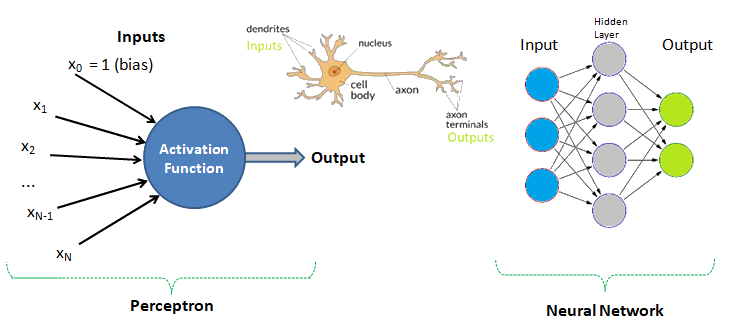Human Brain: Have you tried to search online the keywords "number of neurons in the brain"? The answer is invariably 100 billions! There is another data that we use only 1% of brain and hence do the number 1 billion neurons not astonish you? Can we build a machine and the learning algorithm to deal with similar number of neurons?
Meachine Learning Methods and Scripts

On this page, you will find working examples of most of the machine learning methods in use now-a-days!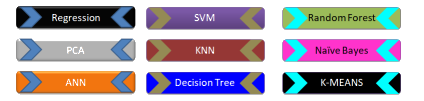Each statement is commented so that you easily connect with the code and the function of each module - remember one does not need to understand everything at the foundational level - e.g. the linear algebra behind each algorithm or optimization operations! The best way is to find a data, a working example script and fiddle with them.

Machine learning, artificial intelligence, cognitive computing, deep learning... are emerging and dominant conversations today all based on one fundamental truth - follow the data. In contrast to explicit (and somewhat static) programming, machine learning uses many algorithms that iteratively learn from data to improve, interpret the data and finally predict outcomes. In other words: machine learning is the science of getting computers to act without being explicitly programmed every time a new information is received.

An excerpt from Machine Learning For Dummies, IBM Limited Edition: "AI and machine learning algorithms aren't new. The field of AI dates back to the 1950s. Arthur Lee Samuels, an IBM researcher, developed one of the earliest machine learning programs - a self-learning program for playing checkers. In fact, he coined the term machine learning. His approach to machine learning was explained in a paper published in the IBM Journal of Research and Development in 1959". There are other topics of discussion such as Chinese Room Argument to question whether a program can give a computer a 'mind, 'understanding' and / or 'consciousness'. This is to check the validity of Turing test developed by Alan Turing in 1950. Turing test is used to determine whether or not computer (or machines) can think (intelligently) like humans.

Big Data

The technical and business newspapers/journals are full of references to "BiG Data". For business, it usually refers to the information that is capture or collected by the computer systems installed to facilitate and monitor various transactions. Online stores as well as traditional bricks-and-mortar retail stores generate wide streams of data. Big data can be and are overwhelming consisting of data table with millions of rows and hundreds if not thousands of columns. Not all transactional data are relevant though!

BiG data are not just big but very often problematic too - containing missing data, information pretending to be numbers and outliers.

Data management is art of getting useful information from raw data generated within the business process or collected from external sources. This is known as data science and/or data analytics and/or big data analysis. Paradoxically, the most powerful growth engine to deal with technology is the technology itself. The internet age has given data too much to handle and everybody seems to be drawining in it. Data may not always end up in useful information and a higher probability exists for it to become a distraction. Machine learning is related concept which deals with Logistic Regression, Support Vector Machines (SVM), k-Nearest-Neighbour (KNN) to name few methods.

Before one proceed further, let's try to recall how we were taught to make us what is designated as an 'educated or learned' person (we all have heard about literacy rate of a state, district and the country).

 Classical Learning Method Example Applicable to Machine Learning? Instructions: repetition in all 3 modes - writing, visual and verbal How alphabets and numerals look like No Rule Counting, summation, multiplication, short-cuts, facts (divisibility rules...) No Mnemonics Draw parallel from easy to comprehend subject to a tougher one: Principal (Main), Principle (Rule) Yes Analogy Comparison: human metabolic system and internal combustion engines No Inductive reasoning and inferences Algebra: sum of first n integers = n(n+1)/2, finding a next digit or alphabet in a sequence Yes Theorems Trigonometry, coordinate geometry, calculus, linear algebra, physics, statistics Yes Memorizing (mugging) Repeated speaking, writing, observing a phenomenon or words or sentences, meaning of proverbs Yes Logic and reasoning What is right (appropriate) and wrong (inappropriate), interpolation, extrapolation Yes Reward and punishment Encourage to act in a certain manner, discourage not to act in a certain manner Yes Identification, categorization and classification Telling what is what! Can a person identify a potato if whatever he has seen in his life is the French fries? Yes
Example Code: Decision Tree

This is just a demonstration (using Python and scikit-learn) of one out of many machine learning methods which let users know what to expect as someone wants to dive deeper. One need not understand every line of the code though comments have been added to make the readers grab most out of it. The data in CSV format can be downloaded from here.

```#-------------------------------------------------------------------------------
#      CLASSIFICATION: 'DECISION TREE' USING PYTHON + SCIKIT-LEARN
#-------------------------------------------------------------------------------
#On WIN10, python version 3.5
#Install scikit-learn: C:\WINDOWS\system32>py.exe -m pip install -U scikit-learn
#pip install -r list.txt - install modules (1 per line) described in 'list.txt'
#-------------------------------------------------------------------------------
# Decision Tree method is a 'supervised' classification algorithm.
# Problem Statement: The task here is to predict whether a person is likely to
# become diabetic or not based on 4 attributes: Glucose, BloodPressure, BMI, Age
#-------------------------------------------------------------------------------
# Import numPy (mathematical utility) and Pandas (data management utility)
import numpy as np
import pandas as pd
import matplotlib.pyplot as plt

# Import train_test_split function from ML utility scikit-learn for Python
from sklearn.model_selection import train_test_split

#Import scikit-learn metrics module for accuracy calculation
from sklearn import metrics

#Confusion Matrix is used to understand the trained classifier behavior over the
#input or labeled or test dataset
from sklearn.metrics import confusion_matrix
from sklearn.metrics import accuracy_score
from sklearn.metrics import classification_report

from sklearn import tree
from sklearn.tree import DecisionTreeClassifier, plot_tree
from sklearn.tree.export import export_text```
# --------- STEP-1: Read the dataset and split into training and test sets------
```# Import dataset: header=0 or header =[0,1] if top 2 rows are headers

# Printing the dataset shape
print ("Dataset Length: ", len(df))
print ("Dataset Shape: ", df.shape)
print (df.columns[0:3])
# Printing the dataset observations

# Split the dataset after separating the target variable
# Feature matrix
X = df.values[:, 0:4] #Integer slicing: note columns 1 ~ 4 only (5 is excluded)
#To get columns C to E (unlike integer slicing, 'E' is included in the columns)

# Target variable (known output - note that it is a supervised algorithm)
Y = df.values[:, 4]

# Splitting the dataset into train and test
X_trn, X_tst, Y_trn, Y_tst = train_test_split(X, Y, test_size = 0.20,
random_state = 10)
#random_state: If int, random_state is the seed used by random number generator
#print(X_tst)

#test_size: if 'float', should be between 0.0 and 1.0 and represents proportion
#of the dataset to include in the test split. If 'int', represents the absolute
#number of test samples.  If 'None',  the value is set to the complement of the
#train size. If train_size is also 'None', it will be set to 0.25. ```
# --------- STEP-2: Train the algorithm----------------------------------------
```# Perform training with giniIndex.  Gini Index is a metric to measure how often
# a randomly chosen element would be incorrectly identified (analogous to false
# positive and false negative outcomes).

# First step: #Create Decision Tree classifier object named clf_gini
clf_gini = DecisionTreeClassifier(criterion = "gini", random_state=100,
max_leaf_nodes=3, max_depth=None, min_samples_leaf=3)

#'max_leaf_nodes': Grow a tree with max_leaf_nodes in best-first fashion. Best
#nodes are defined as relative reduction in impurity. If 'None' then unlimited
#number of leaf nodes.

#max_depth = maximum depth of the tree. If None, then nodes are expanded until
#all leaves are pure or until all leaves contain < min_samples_split samples.

#min_samples_leaf = minimum number of samples required to be at a leaf node. A
#split point at any depth will only be considered if it leaves at least
#min_samples_leaf training samples in each of the left and right branches.

# Second step: train the model (fit training data) and create model gini_clf
gini_clf = clf_gini.fit(X_trn, Y_trn)

# Perform training with entropy, a measure of uncertainty of a random variable.
# It characterizes the impurity of an arbitrary collection of examples. The
# higher the entropy the more the information content.
clf_entropy = DecisionTreeClassifier(criterion="entropy", random_state=100,
max_depth=3, min_samples_leaf=5)
entropy_clf = clf_entropy.fit(X_trn, Y_trn) ```
# --------- STEP-3: Make prediction and check accuracy--------------------------
```# Make predictions with criteria as giniIndex or entropy and calculate accuracy
Y_prd = clf_gini.predict(X_tst)

#y_pred = clf_entropy.predict(X_tst)

#-------Print predicted value for debugging purposes----------------------------
#print("Predicted values:")
#print(Y_prd)

print("Confusion Matrix for BINARY classification as per sciKit-Learn")
print("    TN   |    FP   ")
print("-------------------")
print("    FN   |    TP   ")
print(confusion_matrix(Y_tst, Y_prd))

# Print accuracy of the classification = [TP + TN] / [TP+TN+FP+FN]
print("Accuracy = {0:8.2f}".format(accuracy_score(Y_tst, Y_prd)*100))

print("Classfication Report format for BINARY classifications")
#                           P           R           F           S
#                       Precision     Recall      fl-Score    Support
#       Negatives (0)   TN/[TN+FN]    TN/[TN+FP]  2RP/[R+P]   size-0 = TN + FP
#       Positives (1)   TP/[TP+FP]    TP/[TP+FN]  2RP/[R+P]   size-1 = FN + TP
# F-Score = harmonic mean of precision and recall - also known as the Sorensen–
# Dice coefficient or Dice similarity coefficient (DSC).
# Support = class support size (number of elements in each class).
print("Report: ", classification_report(Y_tst, Y_prd))

''' ---- some warning messages---------------------------------------------------
UndefinedMetricWarning: Precision and F-score are ill-defined and being set to
0.0 in labels with no predicted samples.
- Method used to get the F score is from the "Classification" part of sklearn
- thus it is talking about "labels".  This means that there is no "F-score" to
calculate for some label(s) and F-score for this case is considered to be 0.0.
'''

#from matplotlib.pyplot import figure
#figure(num=None, figsize=(11, 8), dpi=80, facecolor='w', edgecolor='k')
#figure(figsize=(1,1)) would create an 1x1 in image = 80x80 pixels as per given
#dpi argument.

plt.figure()
fig = plt.gcf()
fig.set_size_inches(15, 10)

clf = DecisionTreeClassifier().fit(X_tst, Y_tst)
plot_tree(clf, filled=True)

fig.savefig('./decisionTreeGraph.png', dpi=100)
#plt.show()
#------------------------------------------------------------------------------
#Alternate method to plot the decision tree is to use GraphViz module
#Install graphviz in Pyhton- C:\WINDOWS\system32>py.exe -m pip install graphviz
#Install graphviz in Anaconda: conda install -c conda-forge python-graphviz
#------------------------------------------------------------------------------```
Output from the program:As you can see, machine learning algorithms are different from other algorithms. With most algorithms, a program asks user to feed input and then the algorithm produces the output. However, with machine learning the process is reversed, the data itself creates the model. The more data that is added to the algorithm, the more robust and accurate the algorithm becomes.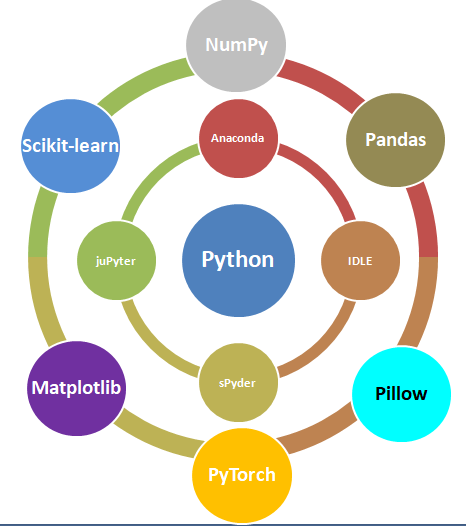Data management is the method and technology of getting useful information from raw data generated within the business process or collected from external sources. Have you noticed that when you search for a book-shelf or school-shoes for your kid on Amazon, you start getting google-ads related to these products when you browse any other website? Your browsing history is being tracked and being exploited to remind you that you were planning to purchase a particular type of product! How is this done? Is this right or wrong? How long shall I get such 'relevant' ads? Will I get these ads even after I have already made the purchase?

The answer to all these questions lies in the way "data analytics" system has been designed and the extent to which it can access user information. For example, are such system allowed to track credit card purchase frequency and amount?

Related fields are data science, big data analytics or simply data analytics. 'Data' is the 'Oil' of 21st century and machine learning is the 'electricity'! This is a theme floating around in every organization, be it a new or a century old well-established company. Hence, a proper "management of life-cycle" of the data is as important as any other activities necessary for the smooth functioning of the organization. When we say 'life-cycle', we mean the 'generation', 'classification', "storage and distribution", "interpretation and decision making" and finally marking them 'obsolete'.

Due to sheer importance and size of such activities, there are many themes such as "Big Data Analytics". However, the organizations need not jump directly to a large scale analytics unless they test and validate a "small data analytics" to develop a robust and simple method of data collection system and processes which later complements the "Big Data Analytics". We also rely on smaller databases using tools which users are most comfortable with such as MS-Excel. This helps expedite the learning curve and sometimes even no new learning is required to get started.

Data - Information - Report

Before proceeding further, let's go back to the basic. What do we really mean by the word 'data'? How is it different from words such as 'information' and 'report'? Data or a dataset is a collection of numbers, labels and symbols along with context of those values. For the information in a dataset to be relevant, one must know the context of the numbers and text it holds. Data is summarized in a table consisting of rows (horizontal entries) and columns (vertical entries). The rows are often called observations or cases.

Columns in a data table are called variables as different values are recorded in same column. Thus, columns of a dataset or data table descripes the common attribute shared by the items or observations.

Let's understand the meaning and difference using an example. Suppose you received an e-mail from your manager requesting for a 'data' on certain topic. What is your common reply? Is it "Please find attached the data!" or is it "Please find attached the report for your kind information!"? Very likely the later one! Here the author is trying to convey the message that I have 'read', 'interpreted' and 'summarized' the 'data' and produced a 'report or document' containing short and actionable 'information'.

The 'data' is a category for 'information' useful for a particular situation and purpose. No 'information' is either "the most relevant" or "the most irrelevant" in absolute sense. It is the information seeker who defines the importance of any piece of information and then it becomes 'data'. The representation of data in a human-friendly manner is called 'reporting'. At the same time, there is neither any unique way of extracting useful information nor any unique information that can be extracted from a given set of data. Data analytics can be applied to any field of the universe encompassing behaviour of voters, correlation between number of car parking tickets issued on sales volume, daily / weekly trade data on projected movement of stock price...

Types of Documents

 Structured Semi-Structured Unstructured The texts, fonts and overall layout remains fixed The texts, fonts and overall layout varies but have some internal structure The texts, fonts and overall layout are randomly distributed Examples are application forms such as Tax Return, Insurance Policies Examples are Invoices, Medical est reports E-mails, Reports, Theses, Sign-boards, Product Labels

Types of Data

Computers understand data in a certain format whereas the nature of data can be numbers as well as words or phrases which cannot be quantified. For example, the difference in "positive and neutral" ratings cannot be quantified and will not be same as difference in "neutral and negative" ratings. There are many ways to describe the type of data we encounter in daily life such as (binary: either 0 or 1), ordered list (e.g. roll number or grade)...Note that even integers can be classified in the context they are used. This is demonstrated from following two examples.
 Nominal Ordinal What is your preferred mode of travel? How will you rate our services? 1 Flights 1 Satisfied 2 Trains 2 Neutral 3 Drive 3 Dissatisfied

While in the first case, digits 1, 2 and 3 are just variable labels [nominal scale] whereas in the second example, the same numbers (digits) indicate an order [ordinal scale]. Similarly, phone numbers and pin (zip) codes are 'numbers' but they form categorical variables as no mathematical operations normally performed on 'numbers' are applicable to them.

Machine Learning

Data Analytics, Data Science, Machine Learning, Artificial Intelligence, Neural Network and Deep Learning are some of the specialized applications dealing with data. There is no well-defined boundaries as they necessarily overlap and the technology itself is evolving at rapid pace. Among these themes, Artificial Neural Network (ANN) is a technology inspired by neurons in human brains and ANN is the technology behind artificial intelligence where attempts are being made to copy how human brain works. 'Data' in itself may not have 'desired' or 'expected' value and the user of the data need to find 'features' to make machine learning algorithms works as most of them expect numerical feature vectors with a fixed size. This is also known as "feature engineering".

• Artificial Intelligence (AI): Self-driving car (autonomous vehicles), speech recognition
• Machine Learning (ML): Given a picture - identify steering angle of a car, Google translate, Face recognition, Identify hand-written letters.
Note that deep learning is a sub-set of machine learning which itself is a sub-set of AI. Following analogies can help distinguish them a bit.
 Artificial Intelligence Machine Learning Deep Learning Engineer Researcher Scientist B. Tech. degree Master's degree PhD
Machine Learning: Techniques and Algorithms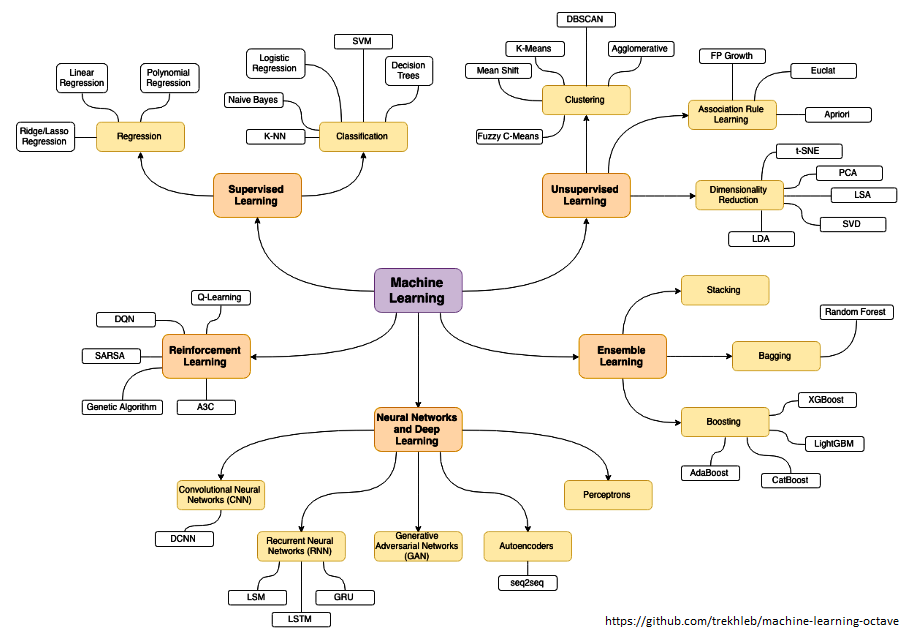The category of supervised and unsupervised learning can be demonstrated as per the chart below. The example applications of each type of the machine learning method helps find a clear distinction among those methods. The methods are nothing new and we do it very often in our daily life. For example, ratings in terms of [poor, average, good, excellent] or [hot, warm, cold] or [below expectations, meets expectations, exceeds expectations, substantially exceeds expectations] can be based on different numerical values. Refer the customer loyalty rating (also known as Net Promoters Score) where a rating below 7 on scale of 10 is considered 'detractors', score between '7 - 8' is rated 'passive' and score only above 8 is considered 'promoter'. This highlights the fact that no uniform scale is needed for classifications.All the algorithms and methods mentioned above has some "optimization objective" which minimizes a "cost function".

Selection of machine learning algorithms: reference e-book "Introducing Machine Learning" by MathWorks.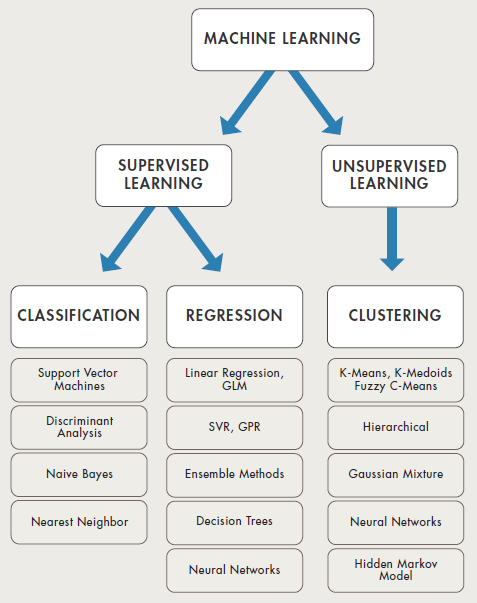Terms with high frequency usage in ML

Machine learning is all about data and data is all about row and column vectors. Each instance of a data or observation is usually represented by a row vector where the first or the last element may be the 'variable or category desired to be predicted'. Thus, there are two broad division of a data set: features and labels (as well as levels of the labels).

• Features: This refers to key characteristics of a dataset or entity. In other words, features are properties that describe each instance of data. Each instance is a point in feature space. For example, a car may have color, type (compact, sedan, SUV), drive-type (AWD, 4WD, FWD, RWD) ... This is also known as predictors, inputs or attributes.
• Label: This is the final identifier such as price category of a car. It is also known as the target, response or output of a feature vector.

As in any textbook, there are solved examples to demonstrate the theory explained in words, equations and figures. And then there are examples (with or without known answers) to readers to solve and check their learnings. The two sets of question can be classified as "training questions" and "evaluation questions" respectively. Similarly in machine learning, we may have a group of datasets where output or label is known and another datasets where labels may not be known.

Training set is an input data where for every predefined set of features 'xi' we have a correct classification y. It is represented as tuples [(x1, y1), (x2, y2), (x3, y3) ... (xk, yk)] which represents 'k' rows in the dataset. Rows of 'x' correspond to observations and columns correspond to variables or attributes or labels. In other words, feature vector 'x' can be represented in matrix notation as: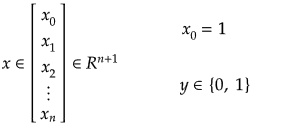Hypothesis (the Machine Learning Model): It is equation that gets features and parameters as an input and predicts the value as an output (i.e. predict if the email is spam or not based on some email characteristics). hθ(x) = g(θTx) where 'T' refers to transpose operation, θ are the (unknown) parameters evaluated during the learning process and 'g' is the Sigmoid function g(u) = [1+e-u]-1 which is plotted below.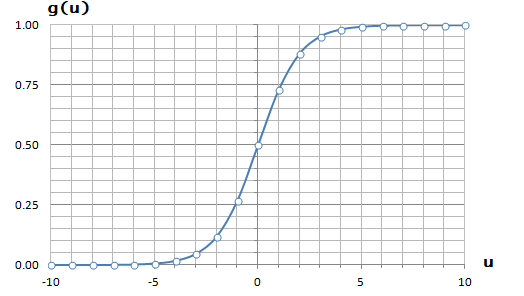hθ(x) = θ0 x0 + θ1 x1 + θ2 x2 + ... + xn θ0 = θTx where 'θ' is a row-vector = [θ0 θ1 θ2 .. θn] and 'x' is a column vector = [x0 x1 x2 ... xn]T. Thus: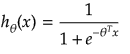Activation Function: The hypothesis for a linear regression can be in the form y = m·x + c or y = a + b·log(c·x). The objective function is to estimate value of 'm' and 'c' by minimizing the square error as described in cost function.

The objective function of a logistic regression can be described as:
• Predict y = 0 if hθ(x) < 0.5 which is true if θTx ≥ 0.
• Predict y = 1 if hθ(x) > 0.5 which is true if θTx < 0. θi are called parameters of the model.

Note that the Sigmoid function looks similar to classical error function and cumulative normal distribution function with mean zero.Another activation function widely used in ML is Rectified Linear Unit (ReLU) function defined as R(z) = max(z, 0) with output in the range 0 ~ ∞. Tanh(z) is yet another activation function which is sigmoidal with output in the range -1 ~ +1. Cost Function: This is the function that shows how accurate the predictions of the hypothesis are with current set of parameters. Cost function also denoted as J(θ) is explained below.

Linear regression: cost function also known as "square error function" is expressed as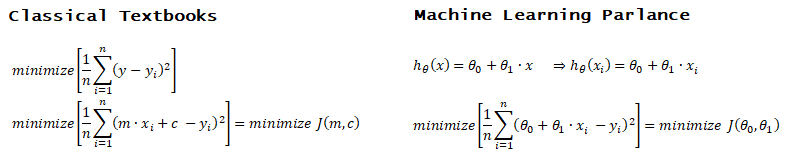Logistic regressionNote that the output 'y' can be either '0' or '1'. Thus, cost function can also be expressed as

Cost(θ) = - y × log[hθ(x)] - (1-y) × [1 - log(hθ(x))]

In other words: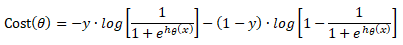Cost function with regularization: Regularization helps keep all the features and reduces magnitude ot θj. It helps avoid over-fitting (high variance) which refers to the situation where output is "too good to be true" - output looks so good that it cannot be deemed true.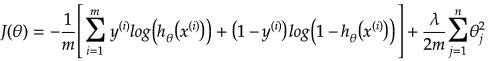Batch Gradient Descent: This is an iterative optimization algorithm for finding the minimum of a cost function described above. To find a local minimum of a function using gradient descent, one takes steps proportional to the negative of the gradient (or approximate gradient) of the function at the current point. When the term "batch" is used for gradient descent it means that each step of gradient descent uses 'all' the training data. There α is an acceleration factor known as "learning rate" in machine learning, analogous to under-relaxation and/or over-relaxation factor. Gradient descent is a multi-variant and generic version of Newtons-Raphson method used to find roots of a polynomial equation.Gradient-Descent with two parameters such as in linear regression:Gradient-Descent with > two parameters such as in logistic regression:Gradient descent method is an iterative method and is not always required for simple problems especially number of parameters are ≤ 2. Feature Scaling: In general features (attributes) of a data set can vary in magnitude where some data would be in fractions and some large integers. Features scaling is used to bring all the features on similar scales, analogous to normalization technique in numerical analyses. It is recommended to bring all features in the range -k ≤ x ≤ +k where 'k' can be 1 or 5 or 10...

Additional method adopted is the "mean normalization" where all the features are displacement such that their means are closer to 0. These two scalings of the features make the gradient descent method faster and ensures convergence.

Normal Equation

This refers to the analytical method to solver for θ. If the matrix XTX is invertible, θ = (XTX)-1XTy where y is column vector of known labels (n × 1). X is features matrix of size n × (m+1) having 'n' number of datasets (rows) in training set and 'm' number of attributes.

If [X] contains any redundant feature (a feature which is dependent on other features), it is likely to be XTX non-invertible.

Logistic Regression in OCTAVE

An implementation of logistic regression in OCTAVE is available on the web. One of these available in GitHub follow the structure shown below.

An explanation of the function add_polynomial_feature.m is described below.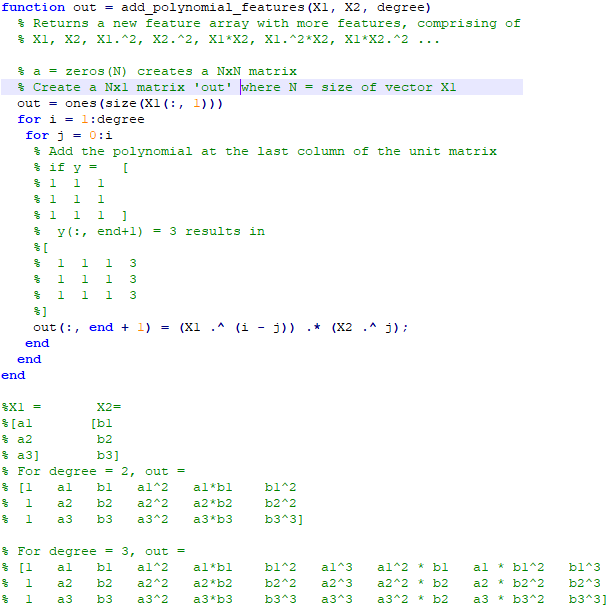Getting the training data: The evaluation of machine learning algorithm requires set of authentic data where the inputs and labels are correctly specified. However, 'make_blobs' module in scikit-learn is a way to generate (pseudo)random dataset which can be further used to train the ML algorithm. Following piece of code available from Python Data Science Handbook by Jake VanderPlas is a great way to start with.

```import matplotlib.pyplot as plt
from sklearn.datasets.samples_generator import make_blobs
X, y = make_blobs(n_samples=400, centers=4, cluster_std=0.60, random_state=0)
X = X[:, ::-1]  # flip axes for better plotting
plt.scatter(X[:, 0], X[:, 1], c=y, s=40, cmap='viridis', zorder=2)
plt.axis('equal')
plt.show()```
This generates a dataset as shown below. Note that the spread of data points can be controlled by value of argument cluster_std.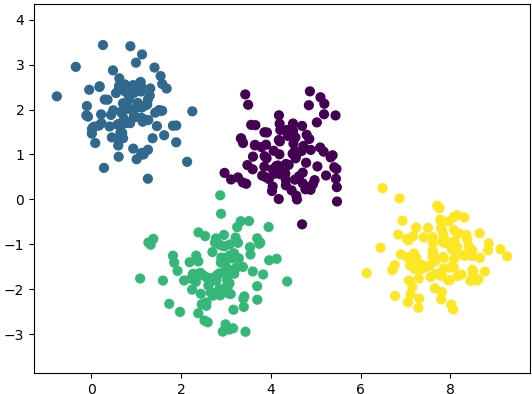Regression vs. Classification:
In regression, output variable requires input variable to be continuous in nature. In classifications, output variables require class label and discrete input values.

Underfitting:

The model is so simple that it cannot represent all the key characteristics of the dataset. In other words, underfitting is when the model had the opportunity to learn something but it didn't. It is said to have high bias and low variance. The confirmation can come from "high training error" and "high test error" values. In regression, fitting a straight line in otherwise parabolic variation of the data is underfitting. Thus, adding a higher degree feature is one of the ways to reduce underfitting. 'Bias' refers to a tendency towards something. e.g. a manager can be deemed biased if he continuously rates same employee high for many years though it may be fair and the employee could have been outperforming his colleagues. Similarly, a learning algorithm may be biased towards a feature and may 'classify' an input dataset to particular 'type' repeatedly. Variance is nothing but spread. As known in statistics, standard deviation is square root of variance. Thus, a high variance refers to the larger scattering of output as compared to mean.

Overfitting:

The model is so detailed that it represents also those characteristics of the dataset which otherwise would have been assumed irrelevant or noise. In terms of human learning, it refers to memorizing answers to questions without understanding them. It is said to have low bias and high variance. The confirmation can come from "very low training error - near perfect behaviour" and "high test error" values. Using the example of curve-fitting (regression), fitting a parabolic curve in otherwise linearly varying data is overfitting. Thus, reducing the degree feature is one of the ways to reduce overfitting. Sometime, overfitting is also described is "too good to be true". That is the model fits so well that in cannot be true.

 ML Performance If number of features increase If number of parameters increase If number of training examples increase Bias Decreases Decreases Remains constant Variance Increases Increases Decreases

Precision and Recall are two other metric used to check the fidelity of the model. In measurements, 'accuracy' refers to the closeness of a measured value to a standard or known value and 'precision' refers to the closeness of two or more measurements to each other. Precision is sometimes also referred as consistency. Following graphics explains the different between accuracy and precision or consistency.In machine learning, 'precision' refers ability of a model to present only 'relevant' information or data. That is, precision = [number of relevant examples selected by model] / [total number of relevant examples presented to the model]. Recall on the other hand refers ability of a model to present all relevant examples. That is, recall = [number of relevant examples selected by model] / [total number of relevant examples available in the dataset].
##### Pickling

Run the program of learning or training the datasets once and use its parameters every time the code is run again - this process is called pickling (analogous to classical pickles we eat)! In scikit-learn, save the classifier to disk (after training):

from sklearn.externals import joblib

joblib.dump(clf, 'pickledData.pkl')

Dimensionality Reduction

It is the process of reducing the number of attributes or labels or random variables by obtaining a set of 'unique' or "linearly independent" or "most relevant" or 'principal' variables. For example, if length, width and area are used as 'label' to describe a house, the area can be a redundant variable which equals length × width. The technique involves two steps: Feature identification/selection and Feature extraction. The dimensionality reduction can also be accomplished by finding a smaller set of new variables, each being a combination of the input variables, containing essentially the same information as the input variables. For example, a cylinder under few circumstances can be represented just by a disk where its third dimension, height or length of the cylinder is assumed to be of less important. Similarly, a cube (higher dimensional data) can be represented by a square (lower dimensional data).

• Principal Component Analysis (PCA): This method dates back to Karl Pearson in 1901, is an unsupervised algorithm that creates linear combinations of the original features. The new features are orthogonal, which means that they are linearly independent or uncorrelated. PCA projects the original samples to a low dimensional subspace, which is generated by the eigen-vectors corresponding to the largest eigen-values of the covariance matrix of all training samples. PCA aims at minimizing the mean-squared-error. [Reference: A survey of dimensionality reduction techniques C.O.S. Sorzano, J. Vargas, A. Pascual-Montano] - The key idea is to find a new coordinate system in which the input data can be expressed with many less variables without a significant error.
• Linear Discriminant Analysis (LDA): A "discriminant function analysis" is used to determine which variables discriminate (differentiate or distinguish) between two or more groups or datasets (it is used as either a hypothesis testing or exploratory method). Thus, LDA like PCA, also creates linear combinations of input features. However, LDA maximizes the separability between classes whereas PCA maximizes "explained variance". The analysis requires the data to have appropriate class labels. It is not suitable for non linear dataset.
• Generalized Discriminant Analysis (GDA): The GDA is a method designed for nonlinear classification based on a kernel function φ which transform the original space X to a new high-dimensional (linear) feature space. In cases where GDA is used for dimensionality reduction techniques, GDA projects a data matrix from a high-dimensional space into a low-dimensional space by maximizing the ratio of "between-class scatter" to "within-class scatter".
Principal Component Analysis - PCA in OCTAVE
```%------------------------------------------------------------------------------
%                                PCA
%------------------------------------------------------------------------------
%PCA: Principal component analysis using OCTAVE -  principal components similar
%to principal stress and strain in Solid Mechanics, represent the directions of
%the data that contains  maximal amount of variance. In  other words, these are
%the lines (in 2D) and planes in (3D) that capture most information of the data.
%Principal components  are less interpretable and may not have any real meaning
%since they are constructed as linear combinations of the initial variables.
%------------------------------------------------------------------------------
%Few references:
%https://www.bytefish.de/blog/pca_lda_with_gnu_octave/
%Video on YouTube by Andrew NG
%------------------------------------------------------------------------------
clc; clf; hold off;
%------------------------------------------------------------------------------
% STEP-1: Get the raw data, for demonstration sake random numbers are used
%------------------------------------------------------------------------------
%Generate an artificial data set of n x m = iR x iC size
iR = 11;  % Total number of rows or data items or training examples
iC = 2;   % Total number of features or attributes or variables or dimensions
k  = 2;   % Number of principal components to be retained out of n-dimensions

X = [2 3; 3 4; 4 5; 5 6; 5 7; 2 1; 3 2; 4 2; 4 3; 6 4; 7 6];
Y = [  1;   2;   1;   2;   1;   2;   2;   2;   1;   2;   2];
c1 = X(find(Y == 1), :);
c2 = X(find(Y == 2), :);

hold on;
subplot(211);  plot(X(:, 1), X(:, 2), "ko", "markersize", 8, "linewidth", 2);
xlim([0 10]); ylim([0 10]);
%
%------------------------------------------------------------------------------
% STEP-2: Mean normalization
%------------------------------------------------------------------------------
% mean(X, 1): MEAN of columns - a row vector {1 x iC}
% mean(X, 2): MEAN of rows - a column vector of size {iR x 1}
% mean(X, n): MEAN of  n-th dimension
mu = mean(X);

% Mean normalization and/or standardization
X1 = X - mu;
Xm = bsxfun(@minus, X, mu);
% Standardization
SD = std(X);     %SD is a row vector - stores STD. DEV. of each column of [X]
W = X - mu / SD;
%------------------------------------------------------------------------------
% STEP-3: Linear Algebra - Calculate eigen-vectors and eigen-values
%------------------------------------------------------------------------------
% Method-1: SVD function
% Calculate eigenvectors and eigenvalues of the covariance matrix. Eigenvectors
% are unit vectors and orthogonal, therefore the norm is one and inner (scalar,
% dot) product is zero. Eigen-vectors are direction of principal components and
% eigen-values are value of variance associated with each of these components.

SIGMA = (1/(iC-1)) * X1 * X1';  % a [iR x iR] matrix
% SIGMA == cov(X')

% Compute singular value decomposition of SIGMA where SIGMA = U*S*V'
[U, S, V] = svd(SIGMA);   % U is iR x iR matrix, sorted in descending order
% Calculate the data set in the new coordinate system.
Ur = U(:, 1:k);
format short G;
Z = Ur' * X1;
round(Z .* 1000) ./ 1000;
%
%------------------------------------------------------------------------------
% Method-2: EIG function
% Covariance matrix is a symmetric square matrix having variance values on the
% diagonal and covariance values off the diagonal. If X is n x m then cov(X) is
% m x m matrix. It is actually the sign of the covariance that matters :
% if positive, the two variables increase or decrease together (correlated).
% if negative, One increases when the other decreases (inversely correlated).

% Compute right eigenvectors V and eigen-values [lambda]. Eigenvalues represent
% distribution of the variance among each of the eigenvectors. Eigen-vectors in
% OCTAVE are sorted ascending, so last column is the first principal component.
[V, lambda] = eig(cov(Xm));  %solve for (cov(Xm) - lambda x [I]) = 0

% Sort eigen-vectors in descending order
[lambda, i] = sort(diag(lambda), 'descend');
V = V(:, i);
D = diag(lambda);

%P = V' * X;    % P == Z
round(V .* 1000) ./ 1000;
%
%------------------------------------------------------------------------------
% STEP-4: Calculate data along principal axis
%------------------------------------------------------------------------------
% Calculate the data set in the new coordinate system, project on PC1 = (V:,1)
x = Xm * V(:,1);
% Reconstruct it and invert mean normalization step
p = x * V(:,1)';
p = bsxfun(@plus, p, mu);  % p = p + mu

%------------------------------------------------------------------------------
% STEP-5: Plot new data along principal axis
%------------------------------------------------------------------------------
%line ([0 1], [5 10], "linestyle", "-", "color", "b");
%This will plot a straight line between x1, y1 = [0, 5] and x2, y2 = [1, 10]

%args = {"color", "b", "marker", "s"};
%line([x1(:), x2(:)], [y1(:), y2(:)], args{:});
%This will plot two curves on same plot: x1 vs. y1 and x2 vs. y2
s = 5;
a1 = mu(1)-s*V(1,1); a2 = mu(1)+s*V(1,1);
b1 = mu(2)-s*V(2,1); b2 = mu(2)+s*V(2,1);
L1 = line([a1 a2], [b1 b2]);

a3 = mu(1)-s*V(1,2); a4 = mu(1)+s*V(1,2);
b3 = mu(2)-s*V(2,2); b4 = mu(2)+s*V(2,2);
L2 = line([a3 a4], [b3 b4]);
args ={'color', [1 0 0], "linestyle", "--", "linewidth", 2};
set(L1, args{:});  %[1 0 0] = R from [R G B]
args ={'color', [0 1 0], "linestyle", "-.", "linewidth", 2};
set(L2, args{:});  %[0 1 0] = G from [R G B]

subplot(212);
plot(p(:, 1), p(:, 2), "ko", "markersize", 8, "linewidth", 2);
xlim([0 10]); ylim([0 10]);
hold off;
%-------------------------------------------------------------------------------
```
The output from this script is shown below. The two dashed lines show 2 (= dimensions of the data set) principal components and the projection over main principal component (red line) is shown in the second plot.Following sections of this page provides some sample code in Python which can be used to extract data from web pages especially stock market related information. Sample code to generate plots using matplotlib module in Python is also included.

Machine Learning Tools: OCTAVE vs. Python (NumPy)
 Usage OCTAVE Python Case sensitive Yes Yes Current working directory pwd import os; os.getcwd() Change working directory chdir F:\OF import os; os.chdir("C:\\Users") Clear screen clc import os; os.system('cls') Convert number to string num2str(123) str(123) End of statement Semi-colon Newline character String concatenation strcat('m = ', num2str(m), ' [kg]') + operator: 'm = ' + str(m) + ' [kg]' Expression list: tuple - x, y, z = 1, 2, 3 Get data type class(x) type(x) Floating points double x float x Integers single x integer x, int(x) User input prompt("x = ")   x = input(prompt) print("x = ") x = input() Floor of division floor(x/y) x // y Power x^y or x**y x**Y Remainder (modulo operator) mod(x,y): remainder(x/y) x%y: remainder(x/y) Conditional operators ==, <, >, != (~=), ≥, ≤ ==, <, >, !=, ≥, ≤ If Loop if ( x == y )  x = x + 1; endif if x == y:   x = x + 1 For Loop for i=0:10  x = i * i;  ... end for i in range(1, 10):  x = i * i Arrays x(5) 1-based x 0-based File Embedding File in same folder from file import function or import file as myFile Defining a Function function f(a, b) ...end def f(a, b):  ... Anonymous (inline) Function y = @(x) x^2; y = lambda x : x**2 Return a single random number between 0 ~ 1 rand(1) random.random() Return a integer random number between 1 and N randi(N) random.randint(1,N) Return a integer random number with seed rand('state', 5) random.seed(5)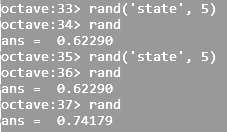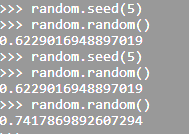Return a single random number between a and b randi([5, 13], 1) random.random(5, 13) Return a (float) random number between a and b a + (b-a)*rand(1) random.uniform(a, b) Return a (float) random number array rand(1, N) numpy.random.rand(N) Stop execution after a statement return sys.exit()

Lambda Functions, also known as anonymous functions as they do not have name. They can have any number of arguments but can only have one expression. These are good for one task that needs repetition. Lambda functions can be used inside other regular functions. In fact, this is their main advantage. f = lambda x: x**2 #Like creating a function f, y = f(5) #Execute the function.

Arrays

aRaY = [] - here aRaY refers to an empty list though this is an assignment, not a declaration. Python can refer aRaY to anything other than a list since Python is dynamically typed. The default built-in Python type is called a 'list' and not an array. It is an ordered container of arbitrary length that can hold a heterogenous collection of objects (i.e. types do not matter). This should not be confused with the array module which offers a type closer to the C array type. However, the contents must be homogenous (all of the same type), but the length is still dynamic.

Python interpreter is written in C language language and that array library is includes array of C language. A string is array of chars in C and hence an array cannot be used to stored strings such as file names.

Summary:

• Floating-point arithmetic always produces a floating-point result. Hence, modulo operator for integers and floating point numbers will yield different results.
• Python uses indentation to show block structure. Indent one level to show the beginning of a block. Out­dent one level to show the end of a block. The convention is to use four spaces (and not the tab character even if it is set to 4 spaces) for each level of indentation. As an example, the following C-style code:
```C                       Python
if (x > 0) {            if x:
if (y > 0)  {              if y:
z = x+y                 z = x+y
}                       z = x*y
z = x*y
}```
Comments: Everything after "#" on a line is ignored. Block comments starts and ends with ''' in Python.
• eye(N), ones(N) and zeros(N) creates a NxN identity matrix, NxN matrix having each element '1' and NxN matrix having each element '0' respectively.
• 'seed' number in random number generators is only a mean to generate same random number again and again. This is equivalent to rng(5) in MATLAB.
• linspace(start, end, num): num = number of points. If omitted, num = 100 in MATLAB/OCTAVE and num = 50 in numpy. Note that it is number of points which is equal to (1 + number of divisions). Hence, if you want 20 divisions, set num = 21. That is, increment Δ = (end - start) / (num - 1). Available in numpy, OCTAVE/MATLAB.
• linspace(1, 20, 20) = 1:20. And hence, reshape(1:20, 4, 5) is equivalent to reshape(linspace(1, 20, 20), 4, 5)
• Arrays: use space or commas to delimit columns, use semi-colon to delimit rows. In same ways, space can be used to stack arrays column-wise (hstack in numpy) e.g. C = [A B] and semi-colon can be used to stack arrays row-wise (vstack in numpy) e.g. C = [A; B].
• In numpy, if x is a 2D array then x[0,2] = x. NumPy arrays can be indexed with other arrays. x[np.array([3, 5, 8])] refers to the third, fifth and eighth elements of array x. Negative values can also be used which work as they do with single indices or slices. x[np.array([-3, -5, -8])] refers to third, fifth and eighth elements from the end of the vector 'x'.
• Negative indexing to call element of an array or a matrix is not allowed in OCTAVE/MATLAB. In case negative counters of a loop is present e.g. j = -n:n, use i = 1:numel(j) to access x(i).
###### Conditional and Logical Indexing
u(A < 25) = 255 replaces the elements of matrix 'u' to 255 corresponding to elements of A which is < 25. If A = reshape(1:20, 4, 5) then B = A(A>15)' will yield B = [16  17  18  19  20]. B = A; B(A < 10) = 0 will replace all those elements of matrix A which are smaller than 10 with zeros.

B = A < 9 will produce a matrix B with 0 and 1 where 1 corresponds to the elements in A which meets the criteria A(i, j) < 9. Similarly C = A < 5 | A > 15 combines two logical conditions.

Find all the rows where the elements in a column 3 is greater than 10. E.g. A = reshape(1:20, 4, 5). B = A(:, 3) > 10 finds out all the rows when values in column 3 is greater than 10. C = A(B:, :) results in the desired sub-matrix of the bigger matrix A.
##### vectorization
This refers to operation on a set of data (array) without any loop. Excerpts from OCTAVE user manual: "Vectorization is a programming technique that uses vector operations instead of element-by-element loop-based operations. To a very good first approximation, the goal in vectorization is to write code that avoids loops and uses whole-array operations". This implicit element-by-element behaviour of operations is known as broadcasting.

Summation of two matrices: C = A + B

```for i = 1:n
for j = 1:m
c(i,j) = a(i,j) + b(i,j);
endfor
endfor ```
Similarly:
```for i = 1:n-1
a(i) = b(i+1) - b(i);
endfor ```
can be simplified as a = b(2:n) - b(1:n-1)

If x = [a b c d], x .^2 = [a2 b2 c2 d2]

The vector method to avoid the two FOR loops in above approach is: C = A + B where the program (numPy or OCTAVE) delegates this operation to an underlying implementation to loop over all the elements of the matrices appropriately.

###### Slicing
This refers to the method to reference or extract selected elements of a matrix or vector. Indices may be scalars, vectors, ranges, or the special operator ':', which may be used to select entire rows or columns. ':' is known as slicing object. The basic slicing syntax is "start: stop: step" where step = increment. Note that the 'stop' value is exclusive that is rows or columns will be included only up to ('stop' - 1). In NumPy (and not in OCTAVE), any of the three arguments (start, stop, step) can be omitted. Default value of 'step' = 1 and default value of 'start' is first row or column. In NumPy (not in OCTAVE) an ellipsis '...' can be used to represent one or more ':'. In other words, an Ellipsis object expands to zero or more full slice objects (':') so that the total number of dimensions in the slicing tuple matches the number of dimensions in the array. Thus, for A[8, 13, 21, 34], the slicing tuple A[3 :, ..., 8] is equivalent to A[3 :, :, :, 8] while the slicing tuple A[..., 13] is equivalent to A[:, :, :, 13].

Slicing against column: B = A(3:2:end, :) will will slice rows starting third row and considering every other row thereafter until the end of the rows is reached. In numpy, B = A[:, : : 2] will slice columns starting from first column and selecting every other column thereafter. Note that the option ': : 2' as slicing index is not available in OCTAVE.

Let's create a dummy matrix A = reshape(1:20, 4, 5) and do some slicing such as B = A(:, 1:2:end).Concatenation of select few rows or columns of two matrices. Create a matrix by repeating columns of a given matrix 'B': C = B(:, [1; 1] * (1:size(B,2)) ).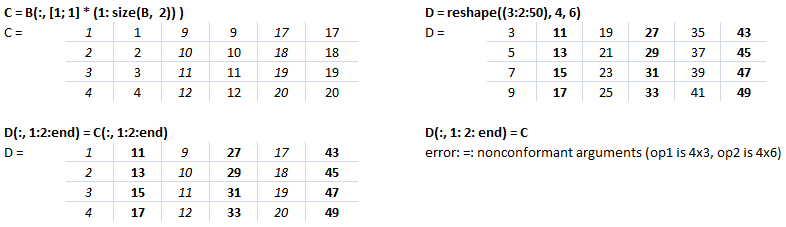Arrays: Example syntax and comparison between OCTAVE and NumPy

 Usage GNU OCTAVE Python / NumPy Definition A = reshape(0:19, 4, 5)' A = numpy.arange(20).reshape(5, 4) Reshape exampleA(3) Scalar - single element - A Not defined Same as A[3, :]: 4th row of matrix/array Special arrays zeros(5, 8), ones(3,5,"int16") np.zeros( (5, 8) ), np.ones( (3, 5), dtype = np.int16) Create array from txt files data = dlmread (fileName, ".", startRow, startCol) np.genfromtxt(fileName, delimiter=",")

3D arrays: widely used in operations on imagesFollowing OCTAVE script can be used to convert the background colour of an image from black to white.
```clc; clear; clear all; [x, map, alpha] = imread ("Img.png"); [nR nC nZ] = size(x);

A = x(:, :, 1); B = x(:, :, 2); C = x(:, :, 3); i = 40; u = A; v = B; w = C;
u(A<i & B<i & C<i) = 255; v(A<i & B<i & C<i) = 255; w(A<i & B<i & C<i) = 255;

z = cat(3, u, v, w); imwrite(z, "newImg.png"); imshow(z); ```LINEAR ALGEBRA

Linear algebra deals with system of linear algebraic equations where the coefficients of independent variables {x} are stored as a matrix [A] and the constant terms on the right hand side of equations are stored as a column vector {b}.

 Usage OTAVE Python (NumPy) Array Index 1-indexed 0-indexed Inverse of a square matrices (a 2D array in numPy) inv(A) inv(A) Find the solution to the linear equation [A].{x} = {b} x = linsolve (A, b) or x = A \ b or x = mldivide (A, b) solve(A, b) Eigen-values (V) and eigen vectors (λ): [A].{x} = λ{x} [V, lambda] = eig (A) eigvals(A): only eigen-values, eig(A): both eigen-values & eigen-vectors Determinant of an array: product of singular values of the array det(A) det(A) Generalized pseudo-inverse of A which is same as the inverse for invertible matrices pinv(A, tol) pinv(A) The rank of a matrix is the number of linearly independent rows or columns and determines how many particular solutions exist to a system of equations. OCTAVE compute the rank of matrix A using the singular value decomposition. Rank: number of singular values of A > specified tolerance tol rank(A, tol) (x, resids, rank, s) = lstsq (A, b, tol) Cholesky decomposition, L of A such that A = LLH chol(A, "lower") cholesky (A): by default it computes lower triangular matrix

#### Statistics

This topics includes basic descriptive statistics, probability distribution functions, hypothesis tests, design-of-experiments (DOE), random number generation ... Descriptive statistics refers to the methods to represent the essence of a large data set concisely such as the mean (average of all the values), median (the value dividing the dataset in two halves), mode (most frequently occurring value in a dataset), range (the difference between the maximum and the minimum of the input data)... functions which all summarize a data set with just a single number corresponding to the central tendency of the data.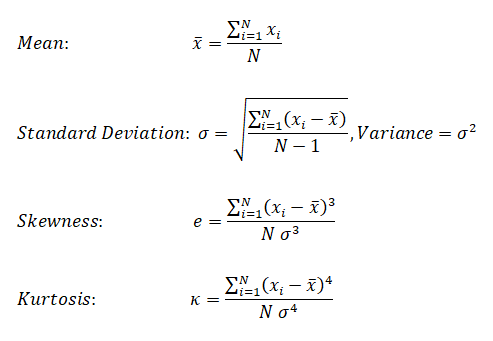Median is the 50 percentile, the value that falls in the middle when the observations are sorted in ascending of descending order. While standard deviation is a measure of central tendency, skewness is the measure of assymetry (skew or bias in the data). Kurtosis is measure of deviation from normal distribution.

 Evaluation parameter OTAVE Python (numPy) Mean (average) mean(x) mean(x) Median (value that divides dataset) median(x) median(x) Mode (most frequently occurring value) mode(x) mode(x) Range range(x) ptp(x) Mean of squares meansq(x) - Variance var(x) var(x) Standard deviation std(x) std(x) Skewness skewness(x) skew(x)* Kurtosis kurtosis(x) kurtosis(x)* All-in-one statistics (x) describe(x)
* from scipy.stats import kurtosis, skew

statistics (x): OCTAVE returns a vector with the minimum, first quartile, median, third quartile, maximum, mean, standard deviation, skewness, and kurtosis of the elements of the vector x.A correlation coefficient value lies between [-1.0, 1.0]. While the sign indicates positive and negative correlation, the absolute value indicates strength of the correlation. Correlation coefficient 1.0 means there is a perfect positive relationship between the two variables, for a increase in one variable, there is also an increase in second variable and vice versa. value of -1.0 refers a perfect negative relationship between the two variables, that is the variables move in opposite directions. For an increase in one variable, there is a decrease in the second variable and vice versa.
##### Cheatsheet: R-programming
• Get help on any specific named function, for example solve, use: help(solve). An alternative is: > ?solve
• R is an expression language and is case sensitive
• Commands are separated either by a semi-colon (‘;'), or by a newline. Elementary commands can be grouped together into one compound expression by braces ‘{' and ‘}'.
• Comments can be put almost anywhere, starting with a hashmark (‘#'), everything to the end of the line is a comment.
• If a command is not complete at the end of a line, R will give a different prompt, by default '+' on second and subsequent lines and continue to read input until the command is syntactically complete.
• If commands are stored in an external file, say commands.r in present working directory: source("commands.r")
• The function sink("record.lis") will divert all subsequent output from the console to an external file, record.lis
• The command sink() restores it to the console once again
• Variable assignment is by either of 3 operators: equal operator '=', leftward operator "<-", rightward operator "->"
• Arrays: c() function which means to combine the elements into a vector. fruits <- c("banana", "apple", "mango"). In R - matrix is a two-dimensional rectangular data set, arrays can be of any number of dimensions set by dim attribute.
• Colon operator - Similar to OCTAVE, ':' creates a series of numbers in sequence for a vector. e.g. v <- 1:5 will create v = (1 2 3 4 5)
• Loops: the syntax for Loops in R is similar to that in C.
• Plots: the plot command in R is similar to that in OCTAVE or MATLAB - plot(x, y). Method to add color and title are different than OCTAVE: color = "green", main = "Chart Tile" is used to specify color of plot and title of the plot respectively.
• In OCTAVE, subplot(231) creates an array of 2x3 = 6 plots. The third digit specifies location of image in 2x3 matrix. In R, mfcol = c(3, 2) sets multiple figure environment and mfg = c(3, 2, 1, 1) specified position of the current figure in a multiple figure environment.

Optimization and regression using Octave - click to get the GNU OCTAVE scripts. Linear Least Square (LSS) is a way to estimate the curve-fit parameters. A linear regression is fitting a straight line y = a0 + a1x where {x} is the independent variable and y is the dependent variable. Since there are two unknowns a0 and a1, we need two equations to solve for them. If there are N data points: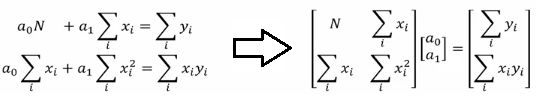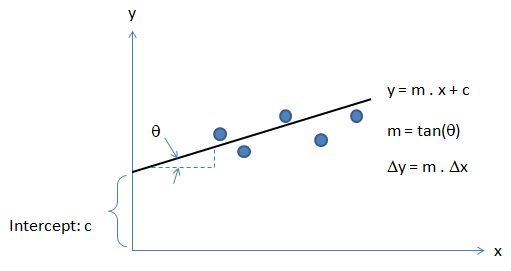As evident from the chart above, the [change in output, Δy] = m × [change in input variable, Δx] in case of linear regression.

Similarly, if dependent variable y is function of more than 1 independent variables, it is called multi-variable linear regression where y = f(x1, x2...xn). The curve fit equations is written as y = a0 + a1x1 + a2x2 + ... + anxn + ε where ε is the curve-fit error. Here xp can be any higher order value of xjk and/or interaction term (xi xj).

Following Python script performs linear regression and plots the discrete data, linear equation from curve-fitting operation and annotates the linear equation on the plot.```import numpy as np

#Specifiy coefficient matrix: independent variable values
x = np.array([0.0, 1.0, 2.0, 3.0, 2.5, 5.0, 4.0])

#Specify ordinate or "dependent variable" values
y = np.array([0.2, 0.3, 0.5, 1.1, 0.8, 2.0, 2.1])

#Create coefficient matrix
A = np.vstack([x, np.ones(len(x))]).T

#least square regression: rcond = cut-off ratio for small singular values of a
#Solves the equation [A]{x} = {b} by computing a vector x that minimizes the
#squared Euclidean 2-norm | b - {A}.{x}|^2
m, c = np.linalg.lstsq(A, y, rcond=None)

print("\n Slope = {0:8.3f}".format(m))
print("\n Intercept = {0:8.3f}".format(c))

import matplotlib.pyplot as plt
_ = plt.plot(x, y, 'o', label='Discrete data', markersize=8)
_ = plt.plot(x, m*x + c, 'r', label='Linear Regression')
_ = plt.legend()

if (c > 0):
eqn = "y ="+str("{0:6.3f}".format(m))+' * x  + '+str("{0:6.3f}".format(c))
else:
eqn = "y ="+str("{0:6.3f}".format(m))+' * x  - '+str("{0:6.3f}".format(abs(c)))

print('\n', eqn)
#Write equation on the plot
# text is right-aligned
plt.text(min(x)*1.2, max(y)*0.8, eqn, horizontalalignment='left')
plt.show()```

If the equation used to fit has exponent of x > 1, it is called a polynomical regression. A quadratic regression uses polynomical of degree 2 (y = a0 + a1x + a2x2 + ε), a cubic regression uses polynomical of degree 3 (y = a0 + a1x + a2x2 + a3x3 + ε) and so on. Since the coefficients are constant, a polynomial regression in one variable can be deemed a multi-variable linear regression where x1 = x, x2 = x2, x3 = x3 ... In scikit-learn, PolynomialFeatures(degree = N, interaction_only = False, include_bias = True, order = 'C') generates a new feature matrix consisting of all polynomial combinations of the features with degree less than or equal to the specified degree 'N'. E.g. poly = PolynomialFeatures(degree=2), Xp = poly.fit_transform(X, y) will transform [x1, x2] to [1, x1, x2, x1*x1, x1*x2, x2*x2]. Argument option "interaction_only = True" can be used to create only the interaction terms. Bias column (added as first column) is the feature in which all polynomial powers are zero (i.e. a column of ones - acts as an intercept term in a linear model).

Polynomial regression in single variable - Uni-Variate Polynomial Regression: The Polynomial Regression can be perform using two different methods: the normal equation and gradient descent. The normal equation method uses the closed form solution to linear regression and requires matrix inversion which may not require iterative computations or feature scaling. Gradient descent is an iterative approach that increments theta according to the direction of the gradient (slope) of the cost function and requires initial guess as well.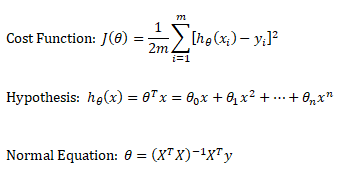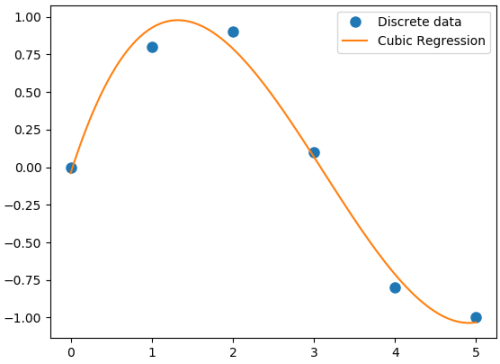```#-------------------------------------------------------------------------------
#Least squares polynomial fit: N = degree of the polynomial
#-------------------------------------------------------------------------------
#Returns a vector of coefficients that minimises the squared error in the order
#N, N-1, N-2 … 0. Thus, the last coefficient is the constant term, and the first
#coefficient is the multiplier to the highest degree term, x^N
#-------------------------------------------------------------------------------
import warnings; import numpy as np
x = np.array([0.0, 1.0, 2.0, 3.0,  4.0,  5.0])
y = np.array([0.0, 0.8, 0.9, 0.1, -0.8, -1.0])
#
N = 3
#full=True: diagnostic information from SVD is also returned
coeff = np.polyfit(x, y, N, rcond=None, full=True, w=None, cov=False)

np.set_printoptions(formatter={'float': '{: 8.4f}'.format})
print("Coefficients: ", coeff)
print("Residuals:", coeff)
print("Rank:", coeff)
print("Singular Values:", coeff)
print("Condition number of the fit: {0:8.2e}".format(coeff))

#poly1D: A 1D polynomial class  e.g. p = np.poly1d([3, 5, 8]) = 3x^2 + 5x + 8
p = np.poly1d(coeff)

xp = np.linspace(x.min(), x.max(),100)
import matplotlib.pyplot as plt
_ = plt.plot(x, y, 'o', label='Discrete data', markersize=8)
_ = plt.plot(xp, p(xp), '-', label='Cubic Regression', markevery=10)
_ = plt.legend()

plt.rcParams['path.simplify'] = True
plt.rcParams['path.simplify_threshold'] = 0.0
plt.show()
#-------------------------------------------------------------------------------```
Output from the above code is:
```Coefficients:  [0.0870  -0.8135   1.6931  -0.0397]
Residuals: [ 0.0397]
Rank: 4
Singular Values: [1.8829   0.6471   0.1878   0.0271]
Condition number of the fit: 1.33e-15```
In addition to 'poly1d' to estimate a polynomial, 'polyval' and 'polyvalm' can be used to evaluate a polynomial at a given x and in the matrix sense respectively. ppval(pp, xi) evaluate the piecewise polynomial structure 'pp' at the points 'xi' where 'pp' can be thought as short form of piecewise polynomial.

Similarly, a non-linear regression in exponential functions such as y = c × ekx can be converted into a linear regression with semi-log transformation such as ln(y) = ln(c) + k.x. It is called semi-log transformation as log function is effectively applied only to dependent variable. A non-linear regression in power functions such as y = c × xk can be converted into a linear regression with log-log transformation such as ln(y) = ln(c) + k.ln(x). It is called log-log transformation as log function is applied to both the independent and dependent variables.

A general second order model is expressed as described below. Note the variable 'k' has different meaning as compared to the one described in previous paragraph. Here k is total number of independent variables and n is number of rows (data in the dataset).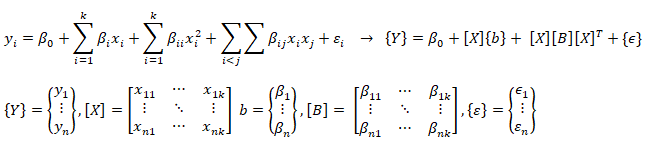For a smaller dataset, linear regression can easily be performed in MS-Excel as shown below. Note that as on version 2016 of MS office, it allows upto 16 independent variables.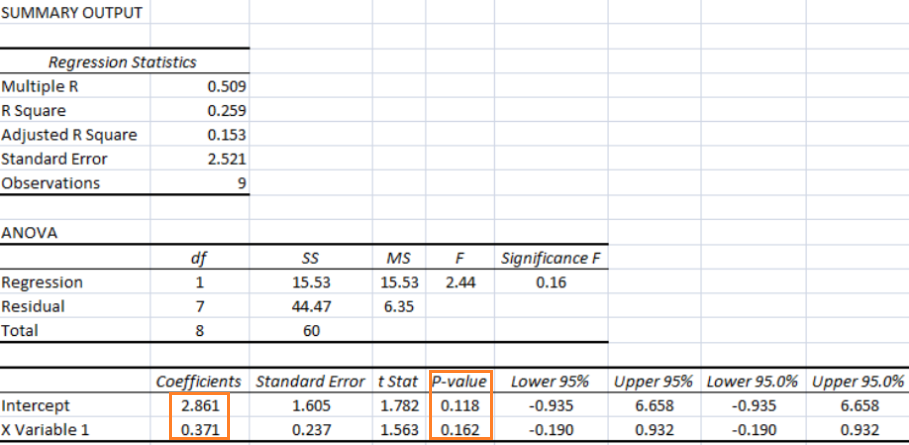By selecting more than 1 columns or rows of independent variables, multi-variable regression can also be performed. Typically, p-value > 0.05 signifies no strong correlation (statistically insignificant) and the column(s) can be ignored. This can be very easily confirmed with scatter plots. The utility correlation and covariance can be used to check multi-collinearity in multi-variable regressions. Multi-collinearity refers to the situation where two independent variables are strongly correlated and one of them can be treated as redundant (a non-contributing factor). For example, dataset on number of bedrooms and total carpet area of houses can be collinear.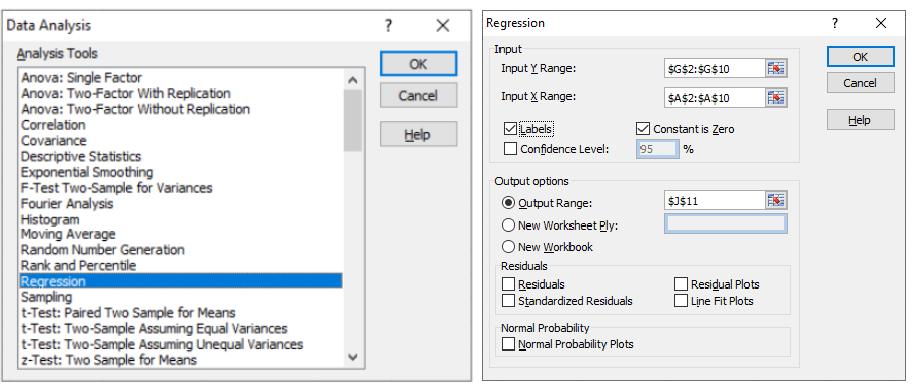Excepts from WWW: Multiple regression (or multi-variable regression) pertains to one dependent variable and multiple independent variables. In multivariate regression there are more than one dependent variable. The purpose of regression is to predict Y on the basis of X or to describe how Y depends on X (regression line/curve). The Xi (X1, X2, ... , Xk) is defined as predictor, explanatory or independent variable, while Y is defined as dependent, response or outcome variable.

As per MathWorks: "The multivariate linear regression model is distinct from the multiple linear regression model, which models a univariate continuous response as a linear combination of exogenous terms plus an independent and identically distributed error term." Note that endogenous and exogenous variables are similar but not same as dependent and independent variables. For example, the curve fit coefficients of a linear regression are variable (since they are based on x and y), they are called endogenous variables - values that are determined by other variables in the system. An exogenous variable is a variable that is not affected by other variables in the system. In contrast, an endogenous variable is one that is influenced by other factors in the system. Here the 'system' may refer to the "regression algorithm".

In summary, categorization of regression types: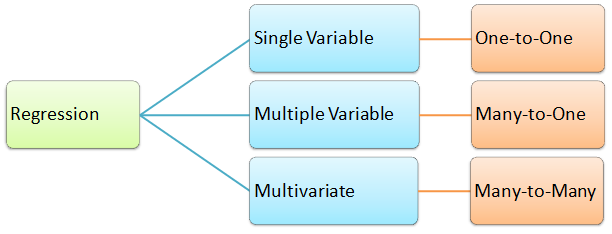3 different approached to generate regression coefficients in Python are described below. Note that the equivalent utility or function in MATLAB is mvregress (not available yet as on Nov-2019 in GNU OCTAVE).
```#----------------------- -------------------------- ---------------------------
import numpy as np
import pandas as pd

X = df.values[0:20, 0:3]
y = df.values[0:20, 3]

#Y = a1x1 + a2x2 + a3x3 + ... + +aNxN + c

#-------- Method-1: linalg.lstsq ---------------------- -----------------------
X = np.c_[X, np.ones(X.shape)] # add bias term
beta_hat = np.linalg.lstsq(X, y, rcond=None)
print(beta_hat)

print("\n------ Runnning Stats Model ----------------- --------\n")
#Ordinary Least Squares (OLS), Install: py -m pip -U statsmodels
from statsmodels.api import OLS
model = OLS(y, X)
result = model.fit()
print (result.summary())

#-------- Method-3: linalg.lstsq ----------------------- ----------------------
print("\n-------Runnning Linear Regression in sklearn ---------\n")
from sklearn.linear_model import LinearRegression
regressor = LinearRegression()
regressor.fit(X, y)
print(regressor.coef_)       #print curve-fit coefficients
print(regressor.intercept_)  #print intercept values
#
#print regression accuracy: coefficient of determination R^2 = (1 - u/v), where
#u is the residual sum of squares and v is the total sum of squares.
print(regressor.score(X, y))
#
#calculate y at given x_i
print(regressor.predict(np.array([[3, 5]]))) ```
Ridge Regression
If data suffers from multicollinearity (independent variables are highly correlated), the least squares estimates result in large variances which deviates the observed value far from the true value (low R-squared, R2). By adding a degree of bias to the regression estimates using a "regulariziation or shrinkage parameter", ridge regression reduces the standard errors. In scikit-learn, it is invoked by "from sklearn.linear_model import Ridge". The function is used by: reg = Ridge(alpha=0.1, fit_intercept=True, normalize=False, solver='auto', random_state=None); reg.fit(X_trn, y_trn). Regularization improves the conditioning of the problem and reduces the variance of the estimates. Larger values specify stronger regularization.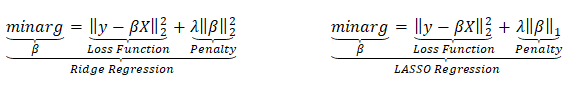##### Lasso Regression
Lasso (Least Absolute Shrinkage and Selection Operator) is similar to ridge repregression which penalizes the absolute size of the regression coefficients instead of squares in the later. Thus, ridge regression uses L2-regularization whereas as LASSO use L1-regularization. In scikit-learn, it is invoked by "from sklearn.linear_model import Lasso". The function is used by: reg = Lasso(); reg.fit(X_trn, y_trn)
##### SVR
Support Vector Machines (SVM) used for classification can be extended to solve regression problems and method is called Support Vector Regression (SVR).

Regression in two variables: example

 X1 X2 y X1 X2 y X1 X2 y X1 X2 y 5 20 100.0 First Interpolation on X2 Second Interpolation on X2 Final interpolation on X1 10 20 120.0 5 20 100.0 10 20 120.0 5 25 200.0 5 40 500.0 5 40 500.0 10 40 750.0 10 25 277.5 10 40 750.0 8 25 ? 5 25 200.0 10 25 277.5 8 25 246.5

 Description Xi1 Xi2 yi First set: Second set: X12 Third set: X11 Fourth set: X21 X32 Desired interpolation point: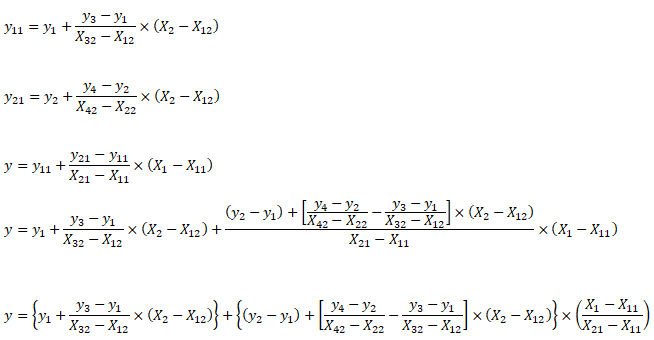POLYNOMIAL MULTI-VARIABLE REGRESSION
```from sklearn.preprocessing import PolynomialFeatures
from sklearn.linear_model import LinearRegression
from sklearn import linear_model
import numpy as np; import pandas as pd
import sys
#Degree of polynomial: note N = 1 implies linear regression
N = 3;
#--------------- DATA SET-1 -------------------- ------------------- -----------
X = np.array([[0.4, 0.6, 0.8], [0.5, 0.3, 0.2], [0.2, 0.9, 0.7]])
y = [10.1, 20.2, 15.5]
#print(np.c_[X, y])

#-------------- DATA SET-2 -------------- ------------------- ------------------
# Function importing Dataset
#Get size of the dataframe. Note that it excludes header rows
iR, iC = df.shape

# Feature matrix
nCol = 5   #Specify if not all columns of input dataset to be considered
X = df.values[:, 0:nCol]
y = df.values[:, iC-1]
#Get names of the features
#print(df.columns.values)

#Print header: check difference between df.iloc[], df.iloc, df.iloc[[0,1]]

#sys.exit()  #Use for debugging

p_reg = PolynomialFeatures(degree = N, interaction_only=False, include_bias=False)
X_poly = p_reg.fit_transform(X)
#X will transformed from [x1, x2] to [1, x1, x2, x1*x1, x1x2, x2*x2]
X_poly = p_reg.fit_transform(X)

#One may remove specific polynomial orders, e.g. 'x' component
#Xp = np.delete(Xp, (1), axis = 1)

#Generate the regression object
lin_reg = LinearRegression()

#Perform the actual regression operation: 'fit'
reg_model = lin_reg.fit(X_poly, y)

#Calculate the accuracy
np.set_printoptions(formatter={'float': '{: 6.3e}'.format})
reg_score = reg_model.score(X_poly, y)
print("\nRegression Accuracy = {0:6.2f}".format(reg_score))
#reg_model.coef_ corresponds to 'feature-1', reg_model.coef_ corresponds
#to 'feature2' and so on. Total number of coeff = 1 + N x m + mC2 + mC3 ...
print("\nRegression Coefficients =", reg_model.coef_)
print("\nRegression Intercepts = {0:6.2f}".format(reg_model.intercept_))
#
from sklearn.metrics import mean_squared_error, r2_score
# Print the mean squared error (MSE)
print("MSE: %.4f" % mean_squared_error(y, reg_model.predict(X_poly)))
# Explained variance score (R2-squared): 1.0 is perfect prediction
print('Variance score: %.4f' % r2_score(y, reg_model.predict(X_poly)))
#
#xTst is set of independent variable to be used for prediction after regression
#Note np.array([0.3, 0.5, 0.9]) will result in error. Note [[ ... ]] is required
#xTst = np.array([[0.2, 0.5]])

#Get the order of feature variables after polynomial transformation
from sklearn.pipeline import make_pipeline
model = make_pipeline(p_reg, lin_reg)
print(model.steps.get_feature_names())

#Print predicted and actual results for every 'tD' row
np.set_printoptions(formatter={'float': '{: 6.3f}'.format})
tD = 3
for i in range(1, round(iR/tD)):
tR = i*tD
xTst = [df.values[tR, 0:nCol]]
xTst_poly = p_reg.fit_transform(xTst)
y_pred = reg_model.predict(xTst_poly)
print("Prediction = ", y_pred, " actual = {0:6.3f}".format(df.values[tR, iC-1])) ```

For all regression activities, statistical analysis is a necessity to determine the quality of the fit (how well the regression model fits the data) and the stability of the model (the level of dependence of the model parameters on the particular set of data). The appropriate indicators for such studies are the residual plot (for quality of the fit) and 95% confidence intervals (for stability of the model).

A web-based application for "Multivariate Polynomial Regression (MPR) for Response Surface Analysis" can be found at TaylorFit-RSA. A dataset to test a multivariable regression model is available at UCI Machine Learning Repository contributed by I-Cheng Yeh, "Modeling of strength of high performance concrete using artificial neural networks", Cement and Concrete Research, Vol. 28, No. 12, pp. 1797-1808 (1998). The actual concrete compressive strength [MPa] for a given mixture under a specific age [days] was determined from laboratory. Data is in raw form (not scaled) having 1030 observations with 8 input variables and 1 output variable.

In general, it is difficult to visualize plots beyond three-dimension. However, the relation between output and two variables at a time can be visualized using 3D plot functionality available both in OCTAVE and MATPLOTLIB.

Following plots are generated in GNU OCTAVE script described later.```%Examples of 3D plots
%-------------------- -------------------------- ------------------------------
%  3D Somerero Plot
%-------------------- -------------------------- ------------------------------
figure ();
%-------------------- -------------------------- ------------------------------
subplot (1,2,1);
tx = ty = linspace(-8, 8, 41)';
[xx, yy] = meshgrid(tx, ty);
r = sqrt(xx .^ 2 + yy .^ 2) + eps;
tz = sin(r) ./ r;

mesh(tx, ty, tz);
xlabel("tx"); ylabel("ty"); zlabel("tz");
title("3-D Sombrero plot");

% Format X-, Y- and Z-axis ticks
xtick = get(gca,"xtick"); ytick = get(gca,"ytick");  ztick = get(gca,"ztick");
xticklabel = strsplit (sprintf ("%.1f\n", xtick), "\n", true);
set (gca, "xticklabel", xticklabel)
yticklabel = strsplit (sprintf ("%.1f\n", ytick), "\n", true);
set (gca, "yticklabel", yticklabel);
zticklabel = strsplit (sprintf ("%.1f\n", ztick), "\n", true);
set (gca, "zticklabel", zticklabel);
%-------------------- -------------------------- ------------------------------
%  3D Helix
%-------------------- -------------------------- ------------------------------
subplot(1,2,2);
t = 0:0.1:10*pi;
r = linspace(0, 1, numel(t));  % numel(t) = number of elements in object 't'
z = linspace(0, 1, numel(t));
plot3(r.*sin(t), r.*cos(t), z);
xlabel("r.*sin (t)"); ylabel("r.*cos (t)"); zlabel("z");
title("3-D helix");

% Format X-, Y- and Z-axis ticks
xtick = get(gca,"xtick"); ytick = get(gca,"ytick");  ztick = get(gca,"ztick");
xticklabel = strsplit (sprintf ("%.1f\n", xtick), "\n", true);
set (gca, "xticklabel", xticklabel)
yticklabel = strsplit (sprintf ("%.1f\n", ytick), "\n", true);
set (gca, "yticklabel", yticklabel);
zticklabel = strsplit (sprintf ("%.1f\n", ztick), "\n", true); ```

The Python code to generate the 3D Helix is as follows.

```import matplotlib as mpl; import matplotlib.pyplot as plt
from mpl_toolkits.mplot3d import Axes3D; import numpy as np
#-------------------- -------------------------- ------------------------------
mpl.rcParams['legend.fontsize'] = 10

fig = plt.figure(); ax = fig.gca(projection='3d')
t = np.linspace(0, 10 * np.pi, 100); r = np.linspace(0, 1, np.size(t));
z = np.linspace(0, 1, np.size(t));   x = r * np.sin(t); y = r * np.cos(t)
#-------------------- -------------------------- ------------------------------
ax.plot(x, y, z, label='3D Helix');  ax.legend(); plt.show()
```

The Python code to generate the 3D Somerero Plot is as follows.

```from mpl_toolkits.mplot3d import Axes3D; import numpy as np
import matplotlib.pyplot as plt; from matplotlib import cm
from matplotlib.ticker import LinearLocator, FormatStrFormatter
#-------------------- -------------------------- ------------------------------
fig = plt.figure(); ax = fig.gca(projection='3d')

tx = np.arange(-8, 8, 1/40);  ty = np.arange(-8, 8, 1/40)
xx, yy = np.meshgrid(tx, ty); r = np.sqrt(xx**2 + yy**2)
tz = np.sin(r) / r
#-------------------- -------------------------- ------------------------------
# Plot the surface
sf = ax.plot_surface(xx,yy,tz, cmap=cm.coolwarm, linewidth=0, antialiased=False)

# Customize the z axis
ax.set_zlim(-1.01, 1.01); ax.zaxis.set_major_locator(LinearLocator(10))
ax.zaxis.set_major_formatter(FormatStrFormatter('%.02f'))

# Add a color bar which maps values to colors
fig.colorbar(sf, shrink=0.5, aspect=5); plt.show()```

Machine Learning: Classification - KNN using Python + sciKit-Learn

KNN is classified as non-parametric method because it does not make any assumption regarding the underlying data distribution. It is part of a "lazy learning technique" because it memorizes the data during training time and computes the distance during testing. It is part of algorithms known as Instance-based Algorithm as the method categorize new data points based on similarities to training data. This set of algorithms are sometimes also referred to as lazy learners because there is no training phase. Lack of training phase does not mean it is an unsupervised method, instead instance-based algorithms simply match new data with training data and categorize the new data points based on similarity to the training data.

```# --------------- --------------------------- ----------------------------------
#        KNN            K-Nearest-Neighbour    Python/scikit-learn
# ------------------------------------------------------------------------------
# Implement K-nearest neighbors (KNN) algorithm: supervised classfication method
# It is a non-parametric learning algorithm, which implies it does not assume
# any pattern (uniformity, Gaussian distribution ...) in training or test data
# --------------- STEP-1 ------------------------- -----------------------------
# Import libraries for maths, reading data and plotting
import numpy as np
import matplotlib.pyplot as plt   #from matplotlib import pyplot as plt
from matplotlib.colors import ListedColormap
import pandas as pd
from sklearn.model_selection import train_test_split
from sklearn.neighbors import NearestNeighbors
#Import classifier implementing the k-nearest neighbors vote.
from sklearn.neighbors import KNeighborsClassifier
#Import to evaluate the algorithm using confusion matrix
from sklearn.metrics import classification_report, confusion_matrix
# --------------- STEP-2 ------------------------ ------------------------------
# Import iris data, assign names to columns and read in Pandas dataframe
# url = "https://archive.ics.uci.edu/ml/machine-learning-databases/iris/iris.data"
header = ['sepal-length', 'sepal-width', 'petal-length', 'petal-width', 'Class']

# Check content of dataset by print top 5 rows

A = dataset.iloc[:, :2].values    # Attributes = X
L = dataset.iloc[:, 4].values     # Labels = y

# Split the dataset into 75% training data and remainder as test data
A_trn, A_tst, L_trn, L_tst = train_test_split(A, L, test_size=0.25)
#test_size: if float, should be between 0.0 and 1.0 and represents proportion
#of the dataset to include in the test split. If int, represents the absolute
#number of test samples. If 'None', the value is set to the complement of the
#train size. If train_size is also 'None', it will be set to 0.25.

# ----------------STEP-3 -------------------------------------------------------
# Performs feature scaling
from sklearn.preprocessing import StandardScaler
scaler = StandardScaler()
scaler.fit(A_trn)
A_trn = scaler.transform(A_trn)
A_tst = scaler.transform(A_tst)

# ----------------STEP-4 -------------------------------------------------------
n_neighbors = 10
#initialize with a parameter: # of neighbors to use for kneighbors queries.
classifier = KNeighborsClassifier(n_neighbors, weights='uniform', algorithm='auto')
# algorithm = 'auto', 'ball_tree', 'kd_tree', 'brute'

#Fit the model using X [A_trn] as training data and y [L_trn] as target values
clf = classifier.fit(A_trn, L_trn)

#Make prediction on provided data [A_tst] (check test_size in train_test_split)
L_pred = classifier.predict(A_tst)

#Return probability estimates for the test data [A_tst]
print(classifier.predict_proba(A_tst))

#Return the mean accuracy on the given test data and labels.
print("\nClassifier Score:")
print(classifier.score(A_tst, L_tst, sample_weight=None))

#Compute confusion matrix to evaluate the accuracy of a classification. By
#definition a confusion matrix C is such that Cij is equal to the number of
#observations known to be in group 'i' but predicted to be in group 'j'. Thus
# in binary classification, the count of true negatives is C(0,0), false
#negatives is C(1,0), true positives is C(1,1) and false positives is C(0,1).
print("\nConfusion matrix:")
print(confusion_matrix(L_tst, L_pred))

#Print the text report showing the main classification metrics
#L_tst: correct target values, L_pred: estimated targets returned by classifier
print(classification_report(L_tst, L_pred))

# ----------------STEP-5 ------------------------ ------------------------------
# Calculating error for some K values, note initialization value was 5
error = []
n1 = 2
n2 = 10
for i in range(n1, n2):
knn = KNeighborsClassifier(n_neighbors=i)
knn.fit(A_trn, L_trn)
pred_i = knn.predict(A_tst)
error.append(np.mean(pred_i != L_tst))

#Plot the error values against K values
plt.figure(figsize=(8, 5))
plt.plot(range(n1, n2), error, color='red', linestyle='dashed', marker='o',
markerfacecolor='blue', markersize=10)
plt.title('Error Rate K Value')
plt.xlabel('K Value')
plt.ylabel('Mean Error')

# ----------------STEP-6 ---------------------------- --------------------------
h = 0.025  #Step size in x-y grid
clf = classifier.fit(A, L)
# Create color maps
cmap_light = ListedColormap(['#009688', '#E0F2F1', 'violet'])
cmap_bold = ListedColormap(['#FF0000', '#00FF00', '#0000FF'])

# Plot the decision boundary and assign a color to each point in the mesh
# [x1, x2]x[y1, y2].
x1, x2 = A[:, 0].min() - 1, A[:, 0].max() + 1
y1, y2 = A[:, 1].min() - 1, A[:, 1].max() + 1
xx, yy = np.meshgrid(np.arange(x1, x2, h), np.arange(y1, y2, h))
Z = clf.predict(np.c_[xx.ravel(), yy.ravel()])

# Put the result into a color plot
Z = Z.reshape(xx.shape)
plt.figure()

plt.pcolormesh(xx, yy, Z, cmap=cmap_light)
# Plot also the training points
plt.scatter(A[:, 0], A[:, 1], c=L, cmap=cmap_bold, edgecolor='k', s=20)
plt.xlim(xx.min(), xx.max())
plt.ylim(yy.min(), yy.max())
plt.title("KNN (k = %i, weights = '%s')" %(n_neighbors, 'uniform'))

plt.show()                #pyplot doesn't show the plot by default```
Outputs from this program are: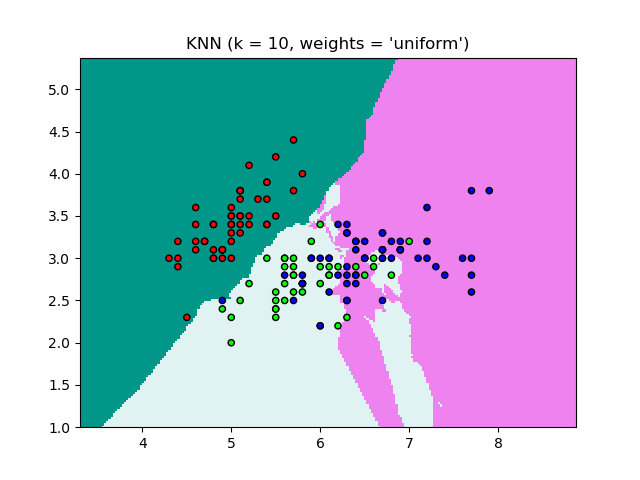The value of k in a KNN output can be judged by smoothness of the boundaries: smoother the boundaries, higher the value of 'k'. Also, there is no unique value of 'k' where an increase in value of 'k' leads to mixing of data in neighbouring classes. A lower value of 'k' gets influenced by outliers (the noise).Machine Learning: Classification by SVM using Python + sciKit-Learn
[x1, x2, x3 ... xm] are known values. [y1, y2 ... yp] are known labels. Equation of hyperplane is f(x) = xTβ + b = 0. Any classification task usually involves following steps:
1. separate data into training and testing sets where each instance in the training set contains one target value or desired output (i.e. the class labels) and several attributes (i.e. the features or observed variables)
2. produce a model (based only on the training data) which predicts the target values
3. check the model on the test data given only the test data attributes

Support vector machines (SVM) were originally designed for binary (type-1 or type-2) classification. Some other methods known for multi-class classification are "one-against-all or one-vs-all", "one-against-one" and Directed Acyclic Graph Support Vector Machines (DAGSVM). SVM requires that each data set is represented as a vector of real numbers as shown below. Each column is known as class or category and each row is an observation (training data).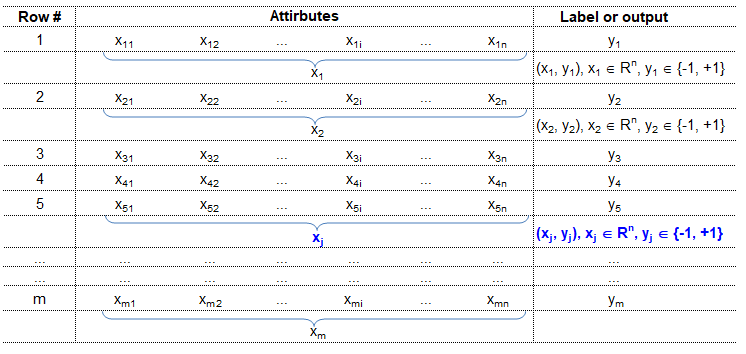"One-against-all or one-vs-all" is also kwnon as One-vs-the-rest (OvR) multiclass / multilabel strategy in scikit-learn.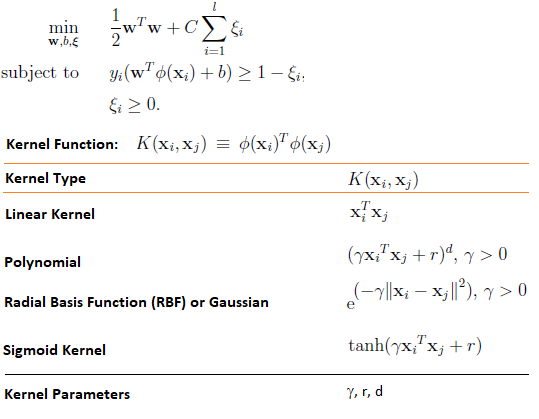Reference: A Practical Guide to Support Vector Classfication by Chih-Wei Hsu, Chih-Chung Chang and Chih-Jen Lin, Department of Computer Science, National Taiwan University, Taipei 106, Taiwan

Scaling before applying SVM is very important. The main advantage of scaling is to avoid attributes in greater numeric ranges dominating those in smaller numeric ranges. Another advantage is to avoid numerical difficulties during the calculation. Because kernel values usually depend on the inner products of feature vectors, e.g. the linear kernel and the polynomial kernel, large attribute values might cause numerical problems. We recommend linearly scaling each attribute to the range [-1; +1] or [0; 1].

In general, the RBF kernel is a reasonable first choice. This kernel nonlinearly maps samples into a higher dimensional space so it can handle the case when the relation between class labels and attributes is nonlinear. If the number of features is large, one may not need to map data to a higher dimensional space. That is, the nonlinear mapping does not improve the performance. Using the linear kernel is good enough, and one only searches for the parameter C.

There are two parameters for an RBF kernel: C and γ. It is not known beforehand which C and γ are best for a given problem; consequently some kind of model selection (parameter search) must be done. The goal is to identify good (C, γ) so that the classifier can accurately predict unknown data (i.e. testing data). If the number of features is large, one may not need to map data to a higher dimensional space. That is, the nonlinear mapping does not improve the performance. Using the linear kernel is good enough, and one only searches for the parameter C.

Support Vector Machines (clustering algorithm) tested for iris.data.```#        ssssssss       v           v       M            M
#        ss              v         v        M  M       M M
#        ss               v       v         M    M   M   M
#        ssssssss          v     v          M      M     M
#              ss           v   v           M            M
#              ss            v v            M            M
#        ssssssss             v             M            M
#
# SVM: "Support Vector Machine" (SVM) is a supervised ML algorithm which can be
#      used for both (multi-class) classification and/or (logistic) regression.
# Support vectors: vectors formed by observations w.r.t. origin
# Support Vector Machine is a separator which best segregates the two or more
# classes (hyperplanes or lines).
# ------------------------------ ---------------------------------------------
from sklearn import svm
import matplotlib.pyplot as plt
import numpy as np
import pandas as pd
#from sklearn import datasets	   # Get pre-defined datasets e.g. iris dataset

# importing scikit learn with make_blobs
from sklearn.datasets.samples_generator import make_blobs

# creating datasets X containing n_samples, Y containing two classes
x, y = make_blobs(n_samples=500, centers=2, random_state=0, cluster_std=0.40)

#Generate scatter plot
#plt.scatter(x[:, 0], x[:, 1], c=y, s=50, cmap='spring')

'''
X = dat.drop('Class', axis=1)   #drop() method  drops the "Class" column
y = dat['Class']
'''
A = df.iloc[:, 2:4].values   # Use the last two features: note 2:4 slice
#To get columns C to E (unlike integer slicing, 'E' is included in the columns)
#df.loc[:, 'C':'E']

L = df.iloc[:, 4].values     # Labels: last column of input data

from sklearn.model_selection import train_test_split
X_trn, X_test, Y_trn, Y_test = train_test_split(A, L, test_size = 0.25)

#plt.scatter(x[:, 0], x[:, 1], c=y, s=50, cmap='spring')
plt.scatter(X_trn[:, 0], X_trn[:, 1], c=Y_trn, cmap=plt.cm.coolwarm)
plt.show()                         #By default, pyplot does not show the plots

#------------------ Specify SVM parameters ------------------------------------
# Specify penalty or regularization parameter 'C'
C = 1.0

# Carry out SVM calculation using kernel 'linear', 'rbf -  Gaussian kernel'
# 'poly', 'sigmoid'. Here rbf, poly -> non-linear hyper-planes
# rbf = Radial Basis Function Kernel
# gamma: Kernel coefficient for 'rbf', 'poly' and 'sigmoid'.
# Higher value of gamma tries to exact fit the training data -> over-fitting

# 'linear' -> classify linearly separable data
'''
from sklearn.svm import SVC
svcLin = SVC(kernel='linear', C=1, gamma='auto')
svcPoly = SVC(kernel='poly', degree=8)
svcc.fit(X_trn, Y_trn)
'''
# Following line of code is equivalent to the 3 short lines described above
svcLin1 = svm.SVC(kernel='linear', C=1.0, gamma='scale').fit(X_trn, Y_trn)
svcRBF = svm.SVC(kernel='rbf', C=1.0, gamma='scale').fit(X_trn, Y_trn)
svcPoly3 = svm.SVC(kernel='poly', C=1.0, degree=3).fit(X_trn, Y_trn)
svcLin2 = svm.LinearSVC(C=1.0, max_iter=10000).fit(X_trn, Y_trn)
# --------------- Create x-y grid to generate a plot --------------------------
#Calculate x- and y-limits
x_min, x_max = X_trn[:, 0].min() - 1, X_trn[:, 0].max() + 1
y_min, y_max = X_trn[:, 1].min() - 1, X_trn[:, 1].max() + 1
#Calculate grid size on x- and y-axis
h = (x_max - x_min)/100
xx, yy = np.meshgrid(np.arange(x_min, x_max, h), np.arange(y_min, y_max, h))

# ----------------- Generate the plot ------------------------------------------
# title for the plots
titles = ['SVC-No-Kernel ', 'SVC-RBF', 'SVC-poly-3', 'LinearSVC']
for i, classifier in enumerate((svcLin1, svcRBF, svcPoly3, svcLin2)):
# Plot the decision boundary and assign a color to each point
plt.subplot(2, 2, i + 1)

#numpy.c_: Translates slice objects to concatenation along the second axis
#numpy.ravel: returns a contiguous flattened array
Z = classifier.predict(np.c_[xx.ravel(), yy.ravel()])

#Put the result into a color plot
Z = Z.reshape(xx.shape)
plt.contourf(xx, yy, Z, cmap=plt.cm.gray, alpha=0.8)

#Plot also the training points
plt.scatter(X_trn[:,0], X_trn[:,1], c=Y_trn, facecolors='none', edgecolors='k')
plt.xlabel('X1')
plt.ylabel('X2')
plt.xlim(xx.min(), xx.max())
plt.ylim(yy.min(), yy.max())
plt.xticks(())
plt.yticks(())
plt.title(titles[i])
plt.show()                        #By default, pyplot does not show the plots
```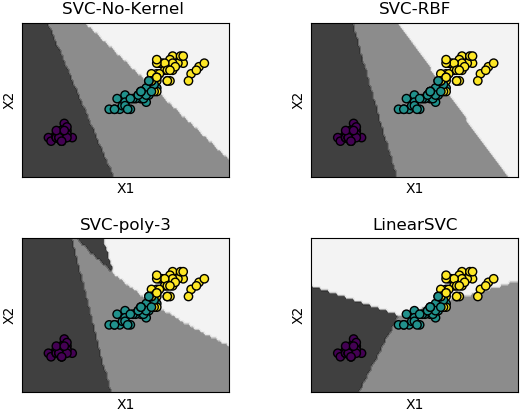Kernels are also known as "similarity function". The "Linear Kernel" option is also called no kernel case. Gaussian kernel is recommended when large set of data is available for training the model.
Machine Learning: Clustering by K-means in GNU OCTAVE

It is an unsupervised clustering algorithm where user needs to specify number of clusters - based on certain insights or even the "later purpose such as number of market segments". Though the number of clusters may not be known a-priori, a practically 'optimum' value can be estimated by "elbow method". It is a plot of cost function (grand total of distances between the cluster centroid and the observations) vs. number of clusters. Very often but not always, the curve looks like a "bent human hand" and the elbow represents the point where the curve has noticeable change in slope - the optimal value of 'K'.

• K-Means is also known as "hard clustering" technique where each input data point is associated with a unique cluster and thus lack flexibility in shape of clusters.
• There is no "probability function" or uncertainty associated with clustering to describe how close or appropriate this classification is.
• k-means inherently assumes that the cluster models must be circular and has no built-in way of accounting for oblong or elliptical clusters.
• Enhancements comes in the form of a GMM - Gaussian Mixture Model. Excerpt from scikit-learn: GMM is a probabilistic model that assumes all the data points are generated from a mixture of a finite number of Gaussian distributions with unknown parameters. This is a generalizing k-means clustering to incorporate information about the covariance structure of the data as well as the centers of the latent Gaussians.
• Thus, GMM is a 'soft' version of k-means.
```# ----Ref: github.com/trekhleb/machine-learning-octave/tree/master/k-means-----
% K-means is an example of unsupervised learning, an iterative method over
% entire data. K-means is a clustering method and not classification method.
% Input is a set of unlabeled data and output from k-means is a set or sub-
% set of coherent data. It is not same as K-Nearest-Neighbours [KNN].
%
% Initialization
clear; close all; clc;

% ------------------------------ Clustering -----------------------------------

%Plot the data.
subplot(2, 2, 1);
plot(X(:, 1), X(:, 2), 'k+','LineWidth', 1, 'MarkerSize', 7);
title('Training Set');

%Train K-Means: The first step is to randomly initialize K centroids.
%Number of centroids: how many clusters are to be defined
K = 3;

%How many iterations needed to find optimal centroids positions 'mu'
max_iter = 100;
% Initialize some useful variables.
[m n] = size(X);

%-------------------------------------- ---------------------------------------
%Step-1: Generate random centroids based on training set. Randomly reorder the
%indices of examples: get a row vector containing a random permutation of 1:n
random_ids = randperm(size(X, 1));

% Take the first K randomly picked examples from training set as centroids
mu = X(random_ids(1:K), :);

%-------------------------------------- ---------------------------------------
%Run K-Means.
for i=1:max_iter
% Step-2a: Find the closest mu for training examples.
%------------------------------------ ---------------------------------------
% Set m
m = size(X, 1);

% Set K
K = size(mu, 1);

% We need to return the following variables correctly.
closest_centroids_ids = zeros(m, 1);

%Go over every example, find its closest centroid, and store
%the index inside closest_centroids_ids at the appropriate location.
%Concretely, closest_centroids_ids(i) should contain the index of centroid
%closest to example i. Hence, it should be a value in therange 1..K
for i = 1:m
d = zeros(K, 1);
for j = 1:K
d(j) = sum((X(i, :) - mu(j, :)) .^ 2);
end
[min_distance, mu_id] = min(d);
closest_centroids_ids(i) = mu_id;
end
%------------------------------------ ---------------------------------------
%Step-2b: Compute means based on closest centroids found in previous step
[m n] = size(X);

%Return the following variables correctly
mu = zeros(K, n);

%Go over every centroid and compute mean of all points that belong to it.
%Concretely, the row vector centroids(i, :) should contain the mean of the
%data points assigned to centroid i.
for mu_id = 1:K
mu(mu_id, :) = mean(X(closest_centroids_ids == mu_id, :));
end
end
%------------------------------------- ----------------------------------------

% Plotting clustered data
subplot(2, 2, 2);
for k=1:K
% Plot the cluster - this is the input data marked as subsets or groups
cluster_x = X(closest_centroids_ids == k, :);
plot(cluster_x(:, 1), cluster_x(:, 2), '+');
hold on;

% Plot the centroid estimated by clustering algorithm
centroid = mu(k, :);
plot(centroid(:, 1), centroid(:, 2), 'ko', 'MarkerFaceColor', 'r');
hold on;
end
title('Clustered Set');
hold off;
%------------------------------------- ----------------------------------------
```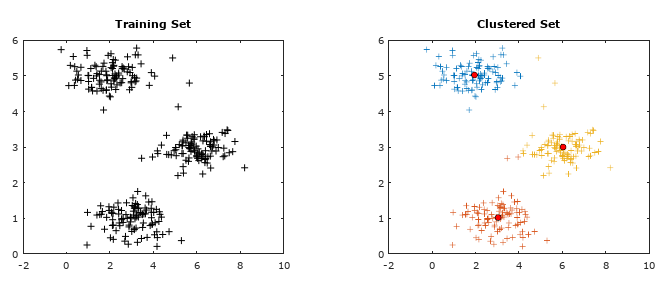Machine Learning: Hierarchical Clustering

This type of clustering method is used on a relatively smaller datasets as the number of computation is proportional to N3 which is computationally expensive on big datasets and may not fit into memory.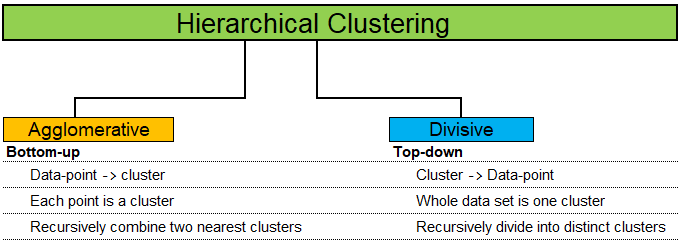Random Forest Algorithm with Python and Scikit-Learn

Random Forest is a supervised method which can be used for regression and classfication though it is mostly used for the later due to inherent limitations in the former. As a forest comprised of trees, a Random Forest method use mutiple Decision Trees to arrive at the classification. Due to multiple trees, it is less prone to overfitting and can handle relatively larger dataset having higher dimensionlity (higher number of features). It is also known as Ensemble Machine Learning algorithm where many weak learning algorithms (the decision trees) are used to generate a majority vote (the stronger team). Bagging and boosting are two methods used in Random Forest learning algorithm to improve its performance: reduce bias and variance, increase accuracy.

Bagging: bootstrap aggregation - where bootstring refers to training samples generated at random but with replacements. e.g. k samples out of N training data.Thus, the rows in each training samples may contain repeated values.

Boosting: it is an iterative approach by adjusting the probability of an instance to be part of subsequent training dataset if it is not correctly classified. The method starts with assigning equal probability to each instance to be part of first training set T1. The classifier C1 is trained on T1. It is then used to predict instances [xi, yi, i = 1, 2, 3 ... N]. If instances xm, xp and xz are not correctly classified, a higher probability will be assigned to these instances to be part on next training set T2. Since the selection of dataset is random, there are rows of dataset which may not make it to the any training set. They are known as out-of-bag dataset. A practically useful boosting algorithm is AdaBoost (which is a shorthand for Adaptive Boosting). The AdaBoost algorithm outputs a hypothesis that is a linear combination of simple hypotheses where an efficient weak learner is 'boosted' into an efficient strong learner.

Following example demonstrates use of Python and sciKit-Learn for classification. Problem Statement: The task here is to predict whether a person is likely to become diabetic or not based on four attributes: Glucose, BloodPressure, BMI, Age.

```import pandas as pd
import numpy as np

# --------- STEP-1: Read the dataset -------------------------------------------

X = dataset.iloc[:, 0:4].values
y = dataset.iloc[:, 4].values

# --------- STEP-2: Split the data into training and test sets -----------------
#Divide data into attributes and labels
from sklearn.model_selection import train_test_split
X_tr, X_ts, y_tr, y_ts = train_test_split(X, y, test_size=0.3, random_state=0)

#test_size: if float, should be between 0.0 and 1.0 and represents proportion
#of the dataset to include in the test split. If int, represents the absolute
#number of test samples. If 'None', the value is set to the complement of the
#train size. If train_size is also 'None', it will be set to 0.25.

# --------- STEP3: Scale the features ------------------------------------------
from sklearn.preprocessing import StandardScaler
sc = StandardScaler()
X_tr = sc.fit_transform(X_tr)
X_ts = sc.transform(X_ts)

# --------- STEP-4: Train the algorithm ----------------------------------------
from sklearn.ensemble import RandomForestClassifier
clf = RandomForestClassifier(n_estimators=20, random_state=0)
clf.fit(X_tr, y_tr)
y_pred = clf.predict(X_ts)
#
# --------- STEP-5: Evaluate the Algorithm -------------------------------------
from sklearn.metrics import classification_report, confusion_matrix
from sklearn.metrics import accuracy_score
#
#scikit-learn.org/stable/modules/generated/sklearn.metrics.confusion_matrix.html
#Compute confusion matrix to evaluate the accuracy of a classification. By
#definition a confusion matrix C is such that Cij is equal to the number of
#observations known to be in group 'i' but predicted to be in group 'j'. Thus
#in binary classification, the count of true negatives is C(0,0), false
#negatives is C(1,0), true positives is C(1,1) and false positives is C(0,1).
#
#In sciKit-learn: By definition, entry (i, j) in a confusion matrix is number of
#observations actually in group 'i', but predicted to be in group 'j'.  Diagonal
#elements represent the number of points for  which the predicted label is equal
#to the true label, while off-diagonal elements are those that are mislabeled by
#the classifier.  Higher the diagonal values of the confusion matrix the better,
#indicating many correct predictions. i = 0, j = 0 -> TN, i = 0, j = 1 -> FP
#
print("Confusion Matrix as per sciKit-Learn")
print("    TN   |    FP   ")
print("-------------------")
print("    FN   |    TP   ")
print(confusion_matrix(y_ts,y_pred))
#
#------------------------------------- -----------------------------------------
#    Confusion matrix in other programs and examples
#
#                                  Actual Values
#                      .----------------------,---------------------.
#   P                  !                      |                     !
#   r   Positives (1)  !  True Positives (TP) | False Positives (FP)!
#   e                  !  Predicted = Actual  | (Type-1 Error)      !
#   d                  !                      |                     !
#   i                  !----------------------|---------------------!
#   c                  !                      |                     !
#   t   Negatives (0)  !  False Negatives (FN)| True Negatives (TN) !
#   e                  !  (Type-II Error)     | Predicted = Actual  !
#   d                  !                      |                     !
#   Value              !......................!.....................|
#
#-------------------------------------- ----------------------------------------
print("Classfication Report format for BINARY classifications")
#                           P           R           F           S
#                       Precision     Recall      fl-Score    Support
#       Negatives (0)   TN/[TN+FN]    TN/[TN+FP]  2RP/[R+P]   size-0 = TN + FP
#       Positives (1)   TP/[TP+FP]    TP/[TP+FN]  2RP/[R+P]   size-1 = FN + TP
#
# F-Score = harmonic mean of precision and recall - also known as the Sorensen–
# Dice coefficient or Dice similarity coefficient (DSC).
# Support = class support size (number of elements in each class).
#
print(classification_report(y_ts, y_prd))
#
# Print accuracy of the classification = [TP + TN] / [TP+TN+FP+FN]
print("Classifier Accuracy = {0:8.4f}".format(accuracy_score(y_ts, y_prd)))
#
# --------- STEP-6: Refine the Algorithm ---------------------------------------
```

Recall: How many relevant items are selected?

Precision: How many selected items are relevant?

Decision Tree: This is a supervised classification method. Refer to the following decision making chart.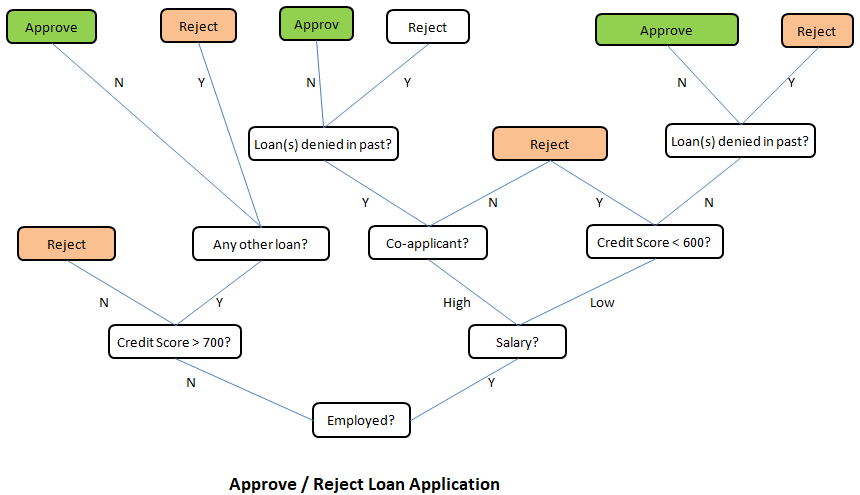Does the above image look like following sketch of a tree?The method "decision tree" inherits this name from the structure of a 'tree' and the final intent to arrive at a 'decision' after going through a set of steps. As evident from the layout, the process starts with a "main node" known as "root node" and branches into other "leaf nodes" like a tree. In machine learning algorithms, following key points need to be addressed: Which attribute to select as root node? How to split attributes? When to stop? Before one gets to the answers, following concepts related to this machine learning methods needs to be understood:
• Entropy: This is a measure of impurity, uncertainty and information content. In thermodynamics and statistical mechanics, entropy is measure of disorder or randomness. In other words, entropy is a measure of lack of order. Entropy = Σ(-pi . log2pi). Entropy concepts have been adopted from information theory. The higher the entropy the more the information content or impurity.
• Information Gain: This is a measure of reduction in uncertainties and hence value lies between 0 and 1. Information gain tells us how important a given attribute of the feature vectors is and hence how useful it is for discriminating between the classes to be learned. Information gain = ENTROPYPARENT - average(ENTROPYCHILDREN).
Q&A:
• What is the entropy of a group in which all examples belong to the same class?
• A: pi = 1.0, Entropy = 0.0. This is not considered a good training set for learning.
• What is the entropy of a group with 50% in either class?
• A: pi = 0.5, Entropy = 1.0. This is considered a good training set for learning.
Example: 3 features and 2 classes:
 Salaried Married Owns a house Invests in Stocks? Low Y 2BHK 1 Low N 2BHK 1 Low Y 3BHK 0 High N 3BHK 0
How can we distinguish class '1' (those who invests in stock market) from class '0'? Since there are two types of labels, let's use following notations:

p+ = fraction of positive examples = 2/4 = 0.5

p- = fraction of negative examples = 2/4 = 0.5

Thus: entropy of parent = Σ(-pi . log2pi) = -p+ log2(p+) - p- log2(p-) = 1.0.

Split on feature 'Salaried'

 Salaried Invests in Stocks? Low 1 Low 1 Low 0 High 0
There are 3 instances of 'Low' resulting in 2 positive label (class). p+,LOW = 2/3. Hence, p-, LOW = 1 - 2/3 = 1/3. Entropy at child node: ELOW = -p+, LOW log2(p+, LOW) - p-, LOW log2(p-, LOW) = -2/3 × log2(2/3) - 1/3 × log2(1/3) = log23 - 2/3.

Similarly, there is 1 instance of 'High' resulting in 1 negative label (class). p+,HIGH = 0. Hence, p-, HIGH = 1 - 0 = 1. Entropy at child node: EHIGH = -p+, HIGH log2(p+, HIGH) - p-, HIGH log2(p-, HIGH) = -0 × log2(0) - 1 × log2(1) = 0.

Information gain = EPARENT - pLOW × ELOW - pHIGH × EHIGH = 1.0 - 3/4 × (log23 - 2/3) - 1/4 × 0 = 1.5 - 3/4×log2(3) =0.3112.

Split on feature 'Married'

 Married Invests in Stocks? Y 1 N 1 Y 0 N 0
There are 2 instances of 'Y' resulting in 1 positive label (class) and 1 negative class. p+,Y = 1/2. Hence, p-, Y = 1.0 - 1/2 = 1/2. Entropy at child node: EY = -p+, Y log2(p+, Y) - p-, Y log2(p-, Y) = -1/2 × log2(1/2) - 1/2 × log2(1/2) = 1.0.

Similarly, there are 2 instances of 'N' resulting in 1 positive label (class) and 1 negative class. p+,N = 1/2. Hence, p-, N = 1.0 - 1/2 = 1/2. Entropy at child node: EN = -p+, N log2(p+, N) - p-, N log2(p-, N) = -1/2 × log2(1/2) - 1/2 × log2(1/2) = 1.0.

Information gain = EPARENT - pY × EY - pN × EN = 1.0 - 2/4 × 1.0 - 2/4 × 1.0 = 0.0.

Split on feature "Owns a House"

 Owns a House Invests in Stocks? 2BHK 1 2BHK 1 3BHK 0 3BHK 0
There are 2 instances of '2BHK' resulting in 2 positive label (class). p+,2BHK = 2/2 =1.0. Hence, p-, 2BHK = 1.0 - 1.0 = 0.0. Entropy at child node: E2BHK = -p+, 2BHK log2(p+, 2BHK) - p-, 2BHK log2(p-, 2BHK) = -1.0 × log2(1.0) - 0.0 × log2(0.0) = 0.0.

Similarly, there are 2 instances of '3BHK' resulting in 2 negative label (class). p-,3HBK = 2/2 = 1.0. Hence, p+, 3BHK = 1.0 - 1.0 = 0.0. Entropy at child node: E3BHK = -p+, 3BHK log2(p+, 3BHK) - p-, 3BHK log2(p-, 3BHK) = -0.0 × log2(0.0) - 1.0 × log2(1.0) = 0.0.

Information gain = EPARENT - p2BHK × E2BHK - p3BHK × E3BHK = 1.0 - 2/4 × 0.0 - 2/4 × 0.0 = 1.0.

Thus splitting on attribute (feature) "Owns a House" is best.

##### Probability in Machine Learning
Bayes theorem is a basis of a popular algorithm known as Naive Bayes Classification. This is widely used in text filtering such as classfication of an e-mail as genuine or spam, categorization of a news article or user comment as fair or abusing / derogatory and the famous Monty Hall problem. However, it gets a bit complicated as the term 'odds' is also used to express the likelyhood of an event - where 'odds' is not same as 'probability'. The odds of an event [E] vs. event "not E" or event [E'] are the ratio of their probabilities. For a standard dice, the odds of rolling digit 3 is [1/6] / [5/6] = 1:5, that the odds are "1 to 5 for" or "5 to 1 against" rolling a 3. The adjective 'Naive' refers to the "Naive assumption" of conditional independence between every pair of a feature.

Bayes theorem is based on conditional probability that is based on some background (prior) information. For example, every year approximately 75 districts in India faces drought situation. There are 725 districts in India. Thus, the proabability that any randomly chosen district will face rain deficit in next year is 75/725 = 10.3%. This value when expressed as ratio will be termed prior odds. However, there are other geological factors that governs the rainfall and the chances of actual deficit in rainfall may be higher or lower than the national average.

Suppose section A of class 8 has 13 boys and 21 girls. Section B of the same class has 18 boys and 11 girls. You randomly calls a student by selecting a section randomly and it turns out to be a girl. What is the probability that the girl is from section A? Let:
• H = event that the student selected is from section 'A'
• G = event that the student selected is a 'girl'
• In terms of conditional probability (it is already known that the student picked is a girl), p(H|G) refers to the probability that the event 'H' occurred given that 'G' is known to have occurred. There is no straightforward way to estimate this value!
• However, it is very easy to calculate the the probability of student picked to be a girl if it is knwon that section chosen is A. That is, p(G|H) = 21/34.
• Bayes theorem is used to estimate p(H|G). Here p(H|G) = p(H)/p(G) × p(G|H). Thus, p(H/G) = [1/2] / [33/63] × 21/34 = 11/68.
• prior probability = p(H) = probability of the hypothesis before we see the data (girl being picked)
• posterior probability = p(H|G) = probability of the hypothesis (girl is from section A) after we see the data (girl is being picked) and the the value one is interested to compute.
• p(G|H) = probability of the data (girl) under the hypothesis (section A), called the likelihood.

In english translation, the meaning or synonyms of 'odds' are 'chances', 'probability', 'likelyhood'. However, 'odds' is distinguished from probability in the sense that the former is always a ratio of two integers where the later is a fraction which can be represented in %. By odds, for example 3:2 (three to 2), we convey that we expect that for every three cases of an outcome (such as a profitable trade), there are two cases of the opposite outcome (not a profitable trade). In other words, chances of a profitable trade are 3/[3+2] = 3/5 or probability of 60%.

If the metrological department of the country announces that it is 80% probability of a normal monsoon this year and it turns out to be a drought. Can we conclude that the weather forecast was wrong. No! The forecast said it is going to be a normal monsoon with 80% probability, which means it may turn out to be drought with 10% probability or 1 out of 5 years. This year turned out to be the 1 in 5 event. Can we conclude that the probability 80% was correct? No! By the same argument one could conclude that 75% chance of normal monsoon was also correct and both cannot be true at the same time.

Likelihood ratio: The ratio used in example above (4 times higher chance of normal monsoon than not a normal monsoon) is called the likelihood ratio. In other words, likelihood ratio is the probability of the observation in case the event of interest (normal monsoon), divided by the probability of the observation in case of no event (drought). The Bayes rule for converting prior odds into posterior odds is:

posterior odds = likelihood ratio × prior odds or posterior odds = Bayes factor × prior odds.

• If "likelihood ratio" > 1, the posterior odds are greater than the prior odds and the data provides evidence in favour of hypothesis.
• If "likelihood ratio" < 1, the posterior odds are smaller than the prior odds and the data provides evidence against the hypothesis.
• If "likelihood ratio" = 1, the posterior odds are greater than the prior odds and the data provides evidence in favour of hypothesis.

Gaussian Naive Bayes on iris data using Python and scikit-learn

```# --------------------------------- --------------------------------------------
# --- Gaussian Naive Bayes on IRIS data,  print confusion matrix as Heat Map ---
# -------------------------------------- ---------------------------------------
import numpy as np
import matplotlib
import matplotlib.pyplot as plt

#There are many built-in data sets. E.g. breast_cancer, iris flower type

#Load the iris dataset which is built into scikit-learn

#This object is  a dictionary and contains a description, features and targets:
#print(iris.keys())
#dict_keys(['target','target_names','data','feature_names','DESCR','filename'])

#Split matrix [iris] into feature matrix [X] and response vector {y}
X = iris.data           # X = iris['data'] - access data by key name
y = iris.target         # y = iris['target']

# ------------------------------------- ----------------------------------------
A = iris.target_names   # A = iris['target_names']
#print(A)
#['setosa' 'versicolor' 'virginica']

F = iris.feature_names  # F = iris['feature_names']
#print(F)
#['sepal length (cm)', 'sepal width (cm)', 'petal length (cm)', 'petal width (cm)']

L = np.array(['Label'])
#print(np.r_[[np.r_[F, L], np.c_[X, y]]])

#-------------------------------------- ---------------------------------------
#Split X and y into training and testing sets
from sklearn.model_selection import train_test_split
X_trn,X_test, y_trn,y_test = train_test_split(X,y, test_size=0.4,random_state=1)

#Train the model on training set
from sklearn.naive_bayes import GaussianNB
gnb = GaussianNB()
clf = gnb.fit(X_trn, y_trn)

#Make predictions on test data
y_pred = gnb.predict(X_test)

#Compare actual response values (y_test) with predicted response values (y_pred)
from sklearn import metrics
from sklearn.metrics import classification_report, confusion_matrix
from sklearn.metrics import accuracy_score

GNBmetric = metrics.accuracy_score(y_test, y_pred)*100
print("Gaussian Naive Bayes model accuracy (in %): {0:8.1f}".format(GNBmetric))

#-------------------------------------- ----------------------------------------
#2D list or array which defines the data to color code in Heat Map
XY = confusion_matrix(y_test, y_pred)
print(XY)

fig, ax = plt.subplots()
#The heatmap is an imshow plot with the labels set to categories defined by user
from matplotlib.colors import ListedColormap
clr = ListedColormap(['red', 'yellow', 'green'])
im = ax.imshow(XY, cmap=clr)

#Define tick marks which are just the ascending integer numbers
ax.set_xticks(np.arange(len(A)))
ax.set_yticks(np.arange(len(A)))

#Ticklabels are the labels to show - the target_names of iris data = vector {A}
ax.set_xticklabels(iris.target_names)
ax.set_yticklabels(iris.target_names)

#Rotate the tick labels and set their alignment.
plt.setp(ax.get_xticklabels(), rotation=45, ha="right", rotation_mode="anchor")

#Loop over the entries in confusion matrix [XY] and create text annotations
for i in range(len(A)):
for j in range(len(A)):
text = ax.text(j, i, XY[i, j], ha="center", va="center", color="w")

ax.set_title("Naive Bayes: Confusion Matrix as Heat Map")
fig.tight_layout()
plt.show()
#-------------------------------------- ----------------------------------------```
This generates the following plot.##### Monty-Hall Problem
Assume that in a TV show the candidate is given the choice between three doors. Behind two of the doors there is a pencil and behind one there is the grand prize, a car. The candidate chooses one door. After that, the showmaster opens another door behind which there is a pencil. Should the candidate switch doors after that? What is the probability of winning the car?
##### Credibility of an Eyewitness
Assume that an eyewitness is 90% certain that a given person was involved in an accident. Moreover, assume that there were 20 people around the parking lot at the time of the crime. What is the posterior probability of the person actually have committed the crime?
##### ANN: Artificial Neural Network
Multi-Layer Perceptron is a supervised learning algorithm which can learn a non-linear function approximator for either classification or regression. It differs from a logistic regression algorithm in construction where between the input and the output layer, there are one or more non-linear (hidden) layers. The simplest neural network consists of only one neuron and is called a perceptron. The perceptron is simply a witty name for the simple neuron model with the step activation function. A method for learning the weights of the perceptron from data called the Perceptron algorithm was introduced by the psychologist Frank Rosenblatt in 1957. It is just about as simple as the nearest neighbor classifier. The basic principle is to feed the network training data one example at a time. Each incorrect classification leads to an update in the weights and biases.

In scikit-learn, MLP is implemented as following classes:

• MLPRegressor: this is a multi-layer perceptron (MLP) class that trains using backpropagation with no activation function in the output layer, which can also be seen as using the identity function as activation function. It uses the sum of square error (SSE) as the loss function and generates output as continuous values.
• MLPClassifier: a multi-layer perceptron (MLP) algorithm that trains using back-propagation that is using some form of gradient descent and the gradients are calculated using back-propagation.
• Many types of activation functions are available such as step function, sigmoid function, relu function and tanh function. In general, (rectified linear unit) ReLU function is used in the hidden layer neurons and sigmoid function is used for the output layer neuron.

Tensors

Tensors are a generalization of matrices. A constant or scalar is 0-dimensional tensor, a vector is a 1-dimensional tensor, a 2×2 matrix is a 2-dimensional tensor, a 3×3 matrix is a 3-dimensional tensor and so on. The fundamental data structure for neural networks are tensors. In summary, arrays, vectors, matrices and tensors are closely related concepts and differ only in the dimensions. All of these are a representation of a set of data with indices to locate and retrieve them.

Steps to create a simple artificial neural network (ANN)

• Step-0: Read the data and manipulate it as per requirements of machine learning algorithms. For example, the labels may be categorical such as 'Rare', 'Low', 'Medium', 'High'. Neural networks (and most machine learning algorithms) work better with numerical data [0, 1, 2...]. Convert the categorical values to numerical values using LabelEncoder class from scikit-learn. Use y.Class.unique() to find unique categories in label vector.
• Step-1: Define the input layer, hidden layer(s) and the output layer. Chose activation function for every hidden layer - Sigmoid activation function is the most widely used one. Multi-layer Perceptron is sensitive to feature scaling, so it is highly recommended to scale the data - StandardScaler from scikit-learn can be used for standardization.
• Step-2: Feedforward or forward propagation: assumed weights [W] and biases [b] and calculate the predicted output y. Estimate accuracy of predictions using a Loss Function such as sum of squares error (SSE).
• Step-3: Back propagation: adjust weights and biases and calculate the predicted output y using gradient decent method which is based on derivative of the loss function with respect to the weights and biases.
• Step-4: Train the model
• Step-5: Test the model
```# -------------------------------- ---------------------------------------------
# --- ANN - Multi-layer Perceptron,  print confusion matrix as Heat Map ---
# ------------------------------------- ----------------------------------------
import numpy as np
import matplotlib
import matplotlib.pyplot as plt

#There are many built-in data sets. E.g. breast_cancer, iris flower type

#Load the iris dataset which is built into scikit-learn

#This object is  a dictionary and contains a description, features and targets:
#print(df.keys())
#dict_keys(['target','target_names','data','feature_names','DESCR','filename'])

#Split matrix [df] into feature matrix [X] and response vector {y}
X = df.data           # X = df['data'] - access data by key name
y = df.target         # y = df['target']

# ------------------------------------- ----------------------------------------
A = df.target_names   # A = df['target_names']
#print(A)
#['setosa' 'versicolor' 'virginica']

F = df.feature_names  # F = df['feature_names']
#print(F)
#['sepal length (cm)', 'sepal width (cm)', 'petal length (cm)', 'petal width (cm)']

L = np.array(['Label'])
#print(np.r_[[np.r_[F, L], np.c_[X, y]]])

#-------------------------------------- ---------------------------------------
# splitting X and y into training and testing sets
from sklearn.model_selection import train_test_split
X_trn,X_test, y_trn,y_test = train_test_split(X,y, test_size=0.4,random_state=1)

#Scale or normalize the data
from sklearn.preprocessing import StandardScaler
#StandardScaler(copy=True, with_mean=True, with_std=True)
scaleDF = StandardScaler()
#Fit to the training data
scaleDF.fit(X_trn)
#Apply transformations to the data
X_trn = scaleDF.transform(X_trn)
X_test = scaleDF.transform(X_test)

#-------------------------------------- ---------------------------------------
#Train the model on training set
from sklearn.neural_network import MLPClassifier
ann_mlp = MLPClassifier(solver='lbfgs', alpha=1e-5,
hidden_layer_sizes=(5, 3), random_state=1)
clf = ann_mlp.fit(X_trn, y_trn)

#hidden_layer_sizes=(5, 3) - two layers having 5 and 3 nodes each
#max_iter = number of cycle of "feed-forward and back propagation" phase.

#Make predictions on the testing set
y_pred = ann_mlp.predict(X_test)

#Compare actual response (y_test) with predicted response (y_pred)
from sklearn import metrics
from sklearn.metrics import classification_report, confusion_matrix
from sklearn.metrics import accuracy_score

MLPmetric = metrics.accuracy_score(y_test, y_pred)*100
print("MLP accuracy(in %): {0:8.1f}".format(MLPmetric))

#-------------------------------------- ----------------------------------------
#2D list or array which defines the data to color code in Heat Map
XY = confusion_matrix(y_test, y_pred)
print(XY)
print(classification_report(y_test, y_pred))

fig, ax = plt.subplots()
#The heatmap is an imshow plot with the labels set to categories defined by user
from matplotlib.colors import ListedColormap
clr = ListedColormap(['grey', 'yellow', 'green'])
im = ax.imshow(XY, cmap=clr)

#Define the tick marks which are just the ascending integer numbers
ax.set_xticks(np.arange(len(A)))
ax.set_yticks(np.arange(len(A)))

#ticklabels are the labels to show - the target_names of iris data = vector {A}
ax.set_xticklabels(df.target_names)
ax.set_yticklabels(df.target_names)

#Rotate the tick labels and set their alignment.
plt.setp(ax.get_xticklabels(), rotation=45, ha="right", rotation_mode="anchor")

#Loop over the entries in confusion matrix [XY] and create text annotations
for i in range(len(A)):
for j in range(len(A)):
text = ax.text(j, i, XY[i, j], ha="center", va="center", color="w")

ax.set_title("ANN - Multi-layer Perceptron: Confusion Matrix")
fig.tight_layout()
plt.show()
#-------------------------------------- ----------------------------------------```
Output of the program - MLP accuracy(in %): 70.0. Note that the lesser accuracy generated by the program does not highlight any deficiency in the algorithm or solver. This is only to show that there is no unique way of chosing the ANN parameters and optimal values need to be worked out by trial-and-error.CNN

Convolutional Neural Networks (ConvNet or CNN) are special type of neural networks that handle image understanding and classification tasks by operating directly on the pixel intensities of input images. Thus, there is no need to explicitly perform any feature extraction operation.
##### Digit Recognition
Excerpts from MathWorks Inc: "Object classification is an important task in many computer vision applications, including surveillance, automotive safety, and image retrieval. For example, in an automotive safety application, you may need to classify nearby objects as pedestrians or vehicles." The hand-written characters come in various shapes, size and colour. The correct classification of digits is one of the key aspects or capabilities of any ANN algorithm. This section describes the ANN implemented in MATLAB or GNU OCTAVE. The process starts with a dataset of digits (MNIST database or any similar ones) stored in this CSV file in Zip format.

The information contained therein can be visualized using following script.

```% ------ Handwritten digits classification ------------------------------------
%clear;
close all; clc;
colormap(gray);  % Use gray image colourmap
%------------------------------------------------------------------------------
% Every row in X is a squared image reshaped into vector, width of each image
% is square root of total number of columns - 1 . The last column represents
% the actual digit hidden in those pictures. There are 500 examples each of 0,
% 1, 2, 3 ... 9.

X =  A(:, 1:end-1);          %All columns except last one are pixels
Y = A(:, end);               %Last column has labels: 10 for digit '0'

% Randomly select N data points: required to split the dataset into training
% and test data. If N > 1, it is number. If it is a fraction, it is % of total
N_trn = 3000;
%------------------------------------------------------------------------------
%
m = size(X, 1);        %Number of rows of X = no. of digits stored in  dataset
n = size(X, 2);        %Number of columns of X
nP = round(sqrt(n));   %Number of pixels rows,columns to represent each digit

% First row:
%   * * * * *  @ @ @ @ @  # # # # #      ```      \$ \$ \$ \$ \$  [1 x n] vector
%
%   * * * * *
%   @ @ @ @ @
%   # # # # #
%      ...
%   \$ \$ \$ \$ \$
%   D(1) = [nP x nP] matrix

% Second row:
%   * * * * *  @ @ @ @ @  # # # # #      ```      \$ \$ \$ \$ \$  [1 x n] vector
%
%   * * * * *
%   @ @ @ @ @
%   # # # # #
%      ...
%   \$ \$ \$ \$ \$
%   D(2) = [nP x nP] matrix
%------------------------------------------------------------------------------
%Set padding: gap (shown as black background) between two consecutive images
pad = 2; ii = 25; jj = 20;
digit = -ones(iR, iC);

for s = 1: 10
% Copy each example into a [nP x nP] square block in the display array digit()
for i = 1:ii
k = (i-1)*jj + 1 + (s-1)*ii*jj;
for j = 1:jj
% Get the max value of current row
max_val = max(abs(X(k, :)));

dR = pad + (i - 1) * (nP + pad) + (1:nP);
dC = pad + (j - 1) * (nP + pad) + (1:nP);

digit(dR, dC) = reshape(X(k, :), nP, nP) / max_val;
k = k + 1;
end
end

%imagesc(img) = display a scaled version of the matrix 'img' as a color image
%Colormap is scaled so that entries of the matrix occupy the entire colormap.
h = imagesc(digit, [-1 1]);    % Display Image
axis image off;        % Do not show axes
%------------------------------------------------------------------------------
% Update figure windows and their children. Only figures that are modified
% will be updated. The refresh function can also be used to cause an update of
% the current figure, even if it is not modified.
drawnow;
str = sprintf(num2str(s-1));
saveas(h, str, 'png');

end```

Rasterize and Vectorize: these are two frequently occuring terms in image handling programs. 'Rasterize' refers to converting an objects / images into pixels (though it is counter-intuitive as images are stored as pixels). Vectorization is a process of converting pixel information into geometry or outline information. The difference can be easily understood when texts are stored as 'non-selectable' images in a PDF (raster form) and same text are stored as 'selectable' objects in a PDF document (vector form).

The images generated for digits 0, 3, 5 and 8 are shown below. The images for digit '1', digit '2', digit '3', digit '4', digit '5', digit '6', digit '7', digit '8' and digit '9' are under the respective hyperlinks.```% ------ Handwritten digits classification -------------------------------------
clear; close all; clc;

%Load training data, display randomly selected 100 data: X is the input matrix
[m n] = size(X);   %Matrix [5000, 400], 1-500: 0, 501-1000: 1, 1001-1500: 2....

%Create random permutation: a column vector of size = size of input [X]
random_digits_indices = randperm(m);

%Select first 100 entries from the random permutation generated earlier
random_digits_indices = random_digits_indices(1:100);

%Display the 100 images stored in 100 rows as [10x10] layout of digits
%display_data(X(random_digits_indices, :));

%------------------------------------------------------------------------------
% Setup the parameters you will use for this part of the exercise
% Specify number of input images of digits.
nD = 30;
input_layer_size = nD*nD;

% 1 <= Number of labels of digits =< 10, (note "0" mapped to label 10)
num_labels = 10;

fprintf('Training One-vs-All Logistic Regression...\n')
lambda = 0.01;
n_iter = 50;  %try 50, 100, 200 and check training set accuracy

% Train the model and predict theta [q] - the label 0 to 9
[all_theta] = one_vs_all_train(X, y, num_labels, lambda, n_iter);

%------------------------------------------------------------------------------
fprintf('Predict for One-Vs-All...\n')
[iR iC] = size(X);
accu = ones(num_labels, 1);
for i = 1: num_labels
if (i == 10)
pred = one_vs_all_predict(all_theta, X(1:500, :));
accu(i) = mean(double(pred == y(1:500))) * 100;
fprintf('\nTraining accuracy for digit 0 = %5.2f [%%]\n', accu(i));
else
j = i * iR/10 + 1;
k = (i+1) * iR/10;
pred = one_vs_all_predict(all_theta, X(j:k, :));
accu(i) = mean(double(pred == y(j:k))) * 100;
fprintf('\nTraining accuracy for digit %d = %5.2f [%%]', i, accu(i));
endif
end
%pred = one_vs_all_predict(all_theta, X);
fprintf('\nOverall training accuracy for all digits: %5.2f [%%]\n', mean(accu));```
Output:
```Training One-vs-All Logistic Regression...
Iteration    50 | Cost: 1.308000e-02
Iteration    50 | Cost: 5.430655e-02
Iteration    50 | Cost: 6.180966e-02
Iteration    50 | Cost: 3.590961e-02
Iteration    50 | Cost: 5.840313e-02
Iteration    50 | Cost: 1.669806e-02
Iteration    50 | Cost: 3.502962e-02
Iteration    50 | Cost: 8.498925e-02
Iteration    50 | Cost: 8.042173e-02
Iteration    50 | Cost: 6.046901e-03
Predict for One-Vs-All...

Training accuracy for digit 1 = 98.40 [%]
Training accuracy for digit 2 = 93.20 [%]
Training accuracy for digit 3 = 91.80 [%]
Training accuracy for digit 4 = 96.00 [%]
Training accuracy for digit 5 = 91.80 [%]
Training accuracy for digit 6 = 98.40 [%]
Training accuracy for digit 7 = 95.20 [%]
Training accuracy for digit 8 = 92.40 [%]
Training accuracy for digit 9 = 92.60 [%]
Training accuracy for digit 0 = 99.80 [%]

Oaverall training accuracy for all digits: 94.96 [%]```
```%-------------- FUNCTION: one_vs_all_train --------------------------------
% Trains logistic regression model each of which recognizes specific number
% starting from 0 to 9. Trains multiple logistic regression classifiers and
% returns all the classifiers in a matrix all_theta, where the i-th row of
% all_theta corresponds to the classifier for label i.
%--------------------------------- --------------------------------------------
function [all_theta] = one_vs_all_train(X, y, num_labels, lambda, num_iter)

[m n] = size(X);
all_theta = zeros(num_labels, n + 1);

% Add column of ones to the X data matrix.
X = [ones(m, 1) X];

for class_index = 1:num_labels
% Convert scalar y to vector with related bit being set to 1.
y_vector = (y == class_index);

% Set options for fminunc
options = optimset('GradObj', 'on', 'MaxIter', num_iter);

% Set initial thetas to zeros.
q0 = zeros(n + 1, 1);

% Train the model for current class.

% Add theta for current class to the list of thetas.
theta = theta';
all_theta(class_index, :) = theta;
end
end```
```% ------ Testing: Make predictions with new images ----------------------------
% Predicts the digit based on one-vs-all logistic regression approach.
% Predict the label for a trained one-vs-all classifier. The labels
% are in the range 1..K, where K = size(all_theta, 1)
function p = one_vs_all_predict(all_theta, X)
m = size(X, 1); num_labels = size(all_theta, 1);

% We need to return the following variables correctly.
p = zeros(m, 1);

% Add ones to the X data matrix
X = [ones(m, 1) X];

% Calculate probabilities of each number for each input example.
% Each row relates to the input image and each column is a probability that
% this example is 1 or 2 or 3...
z = X * all_theta';
h = 1 ./ (1 + exp(-z));

%Now let's find the highest predicted probability for each row: 'p_val'.
%Also find out the row index 'p' with highest probability since the index
%is the number we're trying to predict. The MAX utility is describe below.

%For a vector argument, return the maximum value.  For a matrix argument,
%return a row vector with the maximum value of each column. max (max (X))
%returns the largest element of the 2-D matrix X.  If the optional third
%argument DIM is present then operate along this dimension.  In this case
%the second argument is ignored and should be set to the empty matrix. If
%called with one input and two output arguments, 'max' also returns the
%first index of the maximum value(s).  [x, ix] = max ([1, 3, 5, 2, 5])
%  x = 5, ix = 3

[p_vals, p] = max(h, [], 2);
end ```
Limitations of this script in its current format and structure:
• Any input image has to be in a row format of size [1 x 400] only.
• The image has to be in grayscale and exactly of size 20px × 20px only
• The intensities of pixels must be supplied as signed double precision numbers.
• The background of images has to be dark (black) or gray only with font in white colour. The images with white background and black font will not get recognized.
• The scale of pixel intensities has no unique upper and lower bounds. In the entire dataset, the lowest and the highest values are -0.13196 and 10.0 respectively.For the 4 images shown above, the output from the following lines of code is as follows:
```%------------------------------------------------------------------------------
%Predict one digit at a time: digit from new set
fprintf('-----------------------------------------------------------------\n');
digit = 5; filename = [num2str(digit), ".png"];
Z = vec(im2double(dgt), 2);
%vec: return vector obtained by stacking the columns of the matrix X one above
%other. Without dim this is equivalent to X(:). If dim is supplied, dimensions
%of Z are set to dim with all elements along last dimension. This is equivalent
% to shiftdim(X(:), 1-dim).

pred = one_vs_all_predict(all_theta, Z);
fprintf('\nInput digit = %d, predicted digit = %d \n', digit, pred);
%------------------------------------------------------------------------------```

Input digit = 1, predicted digit = 5

Input digit = 3, predicted digit = 3

Input digit = 4, predicted digit = 4

Input digit = 5, predicted digit = 3
`%------------------------------------------------------------------------------`

Running the program repeatedly, correct prediction for digit 5 was obtained. However, the prediction for digit 1 remained as 5!

Further improvization is possible by writing the answer on the right hand side or bottom of the image. Image is a matrix indexed by row and column values. The plotting system is, however, based on the traditional (x y) system. To minimize the difference between the two systems Octave places the origin of the coordinate system in the point corresponding to the pixel at (1; 1). So, to plot points given by row and column values on top of an image, one should simply call plot with the column values as the first argument and the row values as the second argument.
```%----------------- Example of PLOT over an IMAGE ------------------------------
I = rand (20, 20);              %Generate a 2D matrix of random numbers
[nR, nC] = find (I > 0.95);     %Find intensities greater than 0.95
hold ("on"); imshow (I);        %Show image
plot(nC,nR,"ro"); hold ("off"); %Plot over the image ```
The output will look like: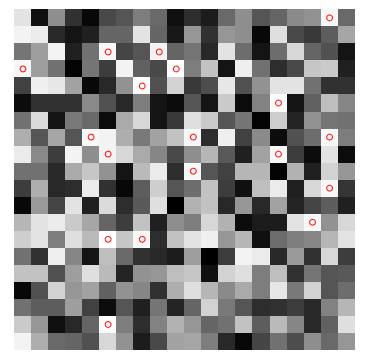##### Digit Classification in Python
```# ------------------------------------------------------------------------------
# --- Random Forest Classifier for Hand-written Digits                       ---
# ------------------------------------------------------------------------------
import matplotlib.pyplot as plt
import pylab as pl

#Load hand-written digits from scikit-learn built-in database

#Use a grayscale image
#pl.gray()
#pl.matshow(digits.images)
#pl.show()
#Check how digits are stored
print("Total digits in dataset are ", len(digits.images))
# ------------------------------------------------------------------------------
#Visualize few images in n x n matrix
n = 10
df = list(zip(digits.images, digits.target))
plt.figure(figsize = [5, 5])
for index, (image, label) in enumerate(df[:n*n]):
plt.subplot(n, n, index+1)
plt.axis('off')
plt.imshow(image, cmap=plt.cm.gray_r, interpolation='nearest')
#plt.title('%i' % label)
plt.show()
# ------------------------------------------------------------------------------
import random
from sklearn import ensemble, metrics
from sklearn.metrics import classification_report, confusion_matrix
from sklearn.metrics import accuracy_score

#Find out the number of digits, store label as variable y
nTest = len(digits.images)
x = digits.images.reshape(nTest, -1)
y = digits.target

#Create random indices to select training images, f = training set fraction
#The method used here is a longer version of train_test_split utility in sklearn
f = 0.20
idxTrain = random.sample(range(len(x)), round(len(x) * f))
idxTest = [i for i in range(len(x)) if i not in idxTrain]

#Sample and validation images
imgTrain = [x[i] for i in idxTrain]
imgTest = [x[i] for i in idxTest]

#Sample and validation targets
yTrain = [y[i] for i in idxTrain]
yTest = [y[i] for i in idxTest]

#Call random forest classifier
clf = ensemble.RandomForestClassifier(n_estimators=20, random_state=0)

#Fit model with training data
clf.fit(imgTrain, yTrain)
# ------------------------------------------------------------------------------
#Test classifier using validation images
score = clf.score(imgTest, yTest)
print("Random Forest Classifier: trained on ", len(imgTrain), "samples")
print("Score = {0:8.4f}". format(score))
#
yPred = clf.predict(imgTest)
XY = confusion_matrix(yTest, yPred)
print(XY)
# ------------------------------------------------------------------------------```
Outputs from this Python code are:```Total digits in dataset are  1797
Random Forest Classifier: trained on  359 samples
Score =   0.9075
[[138   0   0   0   2   0   1   0   1   0]
[  0 134   0   1   0   1   0   0   1   5]
[  1   3 127   6   1   0   1   0   3   1]
[  0   1   5 127   0   0   0   2  10   3]
[  3   2   0   0 141   1   1   4   0   0]
[  1   0   0   3   0 128   1   1   0   5]
[  1   1   0   0   2   0 141   0   0   0]
[  0   0   0   0   1   1   0 136   1   0]
[  0   9   3   2   0   2   2   3 117   0]
[  0   2   0   7   2   5   1  12   5 116]] ```

CBIR: Content Based Image Retrieval systems: method to find similar images to a query image among an image dataset. Example CBIR system is the search of similar images in Google search. Convolutional denoising autoencoder [feed forward neural network] - class of unsupervised deep learning.

##### Computer Vision: OpenCV
Image processing is an integral part of computer vision. OpenCV (Open-source Computer Vision Library) is an open source computer vision and machine learning software library. It integrates itself seamlessly with Python and numPy. In can be installed in Python 3.5 for Windows by command: C:\Windows\System32>py.exe -m pip install -U opencv-python. One can check the version by statement "print(cv2.__version__)" in Python. Python also has a library for image processing called 'Pillow'. Big difference between skimage imread and opencv is that it reads images as BGR instead of RGB (skimage).
• cv2.IMREAD_COLOR: Loads a color image. Any transparency of image will be neglected. It is the default flag. Same as cv2.imread("image_01.png", 1)
• Instead of these three flags, you can simply pass integers 1, 0 or -1 respectively as shown above
• OpenCV and skimage directly store imported images as numpy arrays
• Maintain output window until user presses a key or 1000 ms (1s): cv2.waitKey(0)
• Destroys all windows created: cv2.destroyAllWindows()
Remove Alpha Channel from an Image: imgRGB = cv2.cvtColor(imgRGBA, cv2.COLOR_RGBA2RGB). cv2.COLOR_RGB2RGBA adds alpha channel to the image. img.shape == 4 can be used to check is the image contains alpah channel or not. The conversion from a RGB image to gray: bwsrc = cv.cvtColor(src, 'RGB2GRAY'). Keywords or arguments 'BGR2HSV', 'RGB2HSV', 'HSV2BGR', 'HSV2RGB' can be used as the names suggest. BGR2RGBA, RGB2BGRA, RGBA2BGR, BGRA2RGB, BGR2RGB, RGB2BGR, BGRA2RGBA, RGBA2BGRA: convert between RGB and BGR color spaces (with or without alpha channel) BGR2GRAY, RGB2GRAY, GRAY2BGR, GRAY2RGB, GRAY2BGRA, GRAY2RGBA, BGRA2GRAY, RGBA2GRAY: convert between RGB/BGR and grayscale. Similarly, there are option to convert between RGB/BGR and CIE Lab, convert between RGB/BGR and CIE Luv and many more. NumPy and SciPy arrays of image objects store information as (H, W, D) order (also designated as axis=0, axis=1 and axis=2 respectively. The values can be transposed as img = transpose(-1, 0, 1) = (D, W, H) = transpose(2, 0, 1). Here, (H, W, D) can be access either by (0, 1, 2) or (-3, -2, -1).
 S. No. Operation OpenCV Syntax 01 Open or read Image im = cv2.imread("img/bigData.png", 1) 02 Save or write Image cv2.imwrite("Scaled Image", imgScaled) 03 Show or display Image: First argument is window name, second argument is image cv2.imshow("Original image is", im) 04 Resize or scale Images imgScaled = cv2.resize(im, None, fx=2, fy=2, interpolation = cv2.INTER_CUBIC) 05 Convert images from BGR to RGB imgRGB = cv2.cvtColor(im, cv2.COLOR_BGR2RGB)* 06 Show only blue channel of Image bc = im[:, :, 0]; cv2.imshow("Blue Channel", bc) 07 Show only green channel of Image gc = im[:, :, 1]; cv2.imshow("Green Channel", gc) 08 Show only red channel of Image rc = im[:, :, 2]; cv2.imshow("Red Channel", rc) 09 Split all channel at once bc,gc,rc = cv2.split(im) 10 Merge channels of the Image imgMrg = cv2.merge([bc, gc, rc]) 11 Apply Gaussian Smoothing (Filter) imgGauss = cv2.GaussianBlur(im, (3,3), 0, borderType = cv2.BORDER_CONSTANT) 12 Edge detection imgEdges = cv2.Canny(img, 100, 200) where 100 and 200 are minimum and maximum values 13 Median Blur imgMedBlur = cv2.medianBlur(img, 3) 14 Get dimensions of an image height, width, channels = img.shape

* hsvImg = cv2.cvtColor(im, cv2.COLOR_BGR2HSV); h, s, v = cv2.split(hsvImg) and labImg = cv2.cvtColor(im, cv2.COLOR_BGR2LAB); L, A, B = cv2.split(labImg). Here, HSV stands for Hue, Saturation, Value and LAB - Lightness, A (Green to red), B (Blue to Yellow).

To read-write images: from skimage import io, to apply filters: from skimage import filters or from skimage.filters import gaussian, sobel.

 S. No. Operation skimage Syntax 01 Open or read Image im = io.imread("img/bigData.png", as_gray=False) 02 Save or write Image io.imsave("Scaled Image", imgScaled) 03 Show or display Image io.imshow(im) 04 Resize or scale Images imgScaled = rescale(img, 2.0, anti_aliasing = False), imgSized = resize(img, (500, 600), anti_aliasing = True) 05 Convert images from BGR to RGB imgRGB = cv2.cvtColor(im, cv2.COLOR_BGR2RGB)* 06 Show only blue channel of Image bc = im[:, :, 0]; cv2.imshow("Blue Channel", bc) 07 Show only green channel of Image gc = im[:, :, 1]; cv2.imshow("Green Channel", gc) 08 Show only red channel of Image rc = im[:, :, 2]; cv2.imshow("Red Channel", rc) 09 Split all channel at once bc,gc,rc = cv2.split(im) 10 Merge channels of the Image imgMrg = cv2.merge([bc,gc,rc]) 11 Apply Gaussian Smoothing (Filter)** imgGauss = filters.gaussian(im, sigma=1, mode='constant', cval=0.0) 13 Median Blur*** imgMedBlur = median(img, disk(3), mode='constant', cval=0.0) 14 Get dimensions of an image w = img.size, h = img.size
Note that the arguments can be stored as a variable: For example ---- ai= cv2.imread("bigData.png"); ci = cv2.cvtColor(ai, cv2.COLOR_BGR2RGB); cv2.imwrite("img/imgGauss" + str(i) + ".png", ci)

** 'sigma' defines the std dev of the gaussian kernel, different from cv2

**** from skimage.morphology import disk

##### Image and Video Enhancement - Convolution

Before proceeding to Enhancement, let's explore the image basics first: Brightness, Contrast, Alpha, Gamma, Transparency, Hue, Saturation... are few of the terms which should be clearly understood to follow the techniques used for image enhancements. Brightness: it refers to depth (or energy or intensity) of colour with respect to some reference value. Contrast: the difference between maximum and minimum pixel intensity in an image. The contrast makes certain portion of an image distinguishable with the remaining.

Convolution: This is special type of matrix operation defined below. Convolution is the most widely used method in computer vision problems and algorithms dealing with image enhancements. There matrix 'f' is known as convolution filter or kernel, which is usually 'odd' in size. Strictly speaking the method explained here is cross-correlation. However, this definition is widely used as convolution in machine language applications.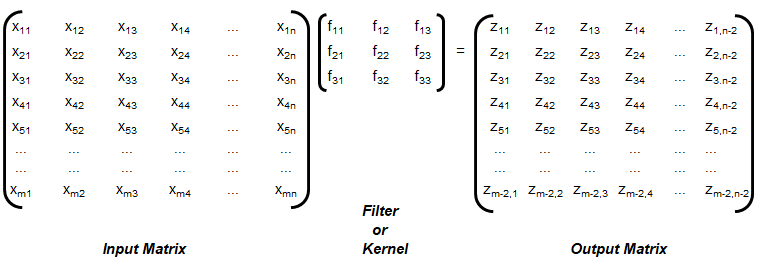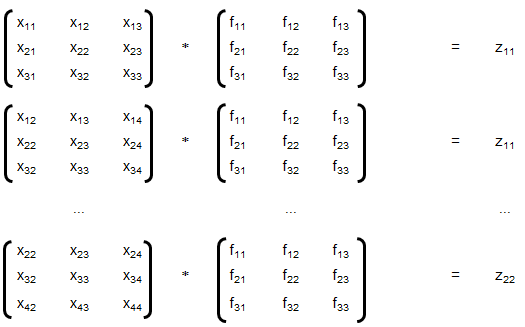The convolution explained above is known as 'valid', without padding. Note that the size of output matrix has reduced by 2 in each dimension. Sometimes, padding is used where elements or layers of pixels are added all around, that is p rows and p columns are added to the input matrix with (conventionally) zeros. This helps get the output matrix of same size as that of input matrix. This is known as 'same' convolution. Similarly, the "strided convolution" use matrix multiplications in 'strides' or 'steps' where more than 1 rows and columns are stepped in the calculation of zij.

Convolution is a general method to create filter effect for images where a matrix is applied to an image matrix and a mathematical operation (generally) comprising of integers. The output after convolution is a new modified filtered image with a slight blur, Gaussian blur, edge detection... The smaller matrix of numbers or fractions that is used in image convolutions is called a Kernel. Though the size of a kernel can be arbitrary, a 3 × 3 is often used. Some examples of filters are:Following OCTAVE script produces 7 different type of images for a given coloured image as input.

The Sobel kernel may not be effective at all for images which do not have sharp edges. The GNU OCTAVE script used to generate these image enhancements and convolutions is described here.
```%In general Octave supports four different kinds of images
%       grayscale images|RGB images            |binary images  | indexed images
%       [M x N] matrix  |[M x N x 3] array     |[M x N] matrix | [M x N] matrix
%class: double          |double, uint8, uint16 |class: logical | class: integer

%The actual meaning of the value of a pixel in a grayscale or RGB image depends
%on the class of the matrix. If the matrix is of class double pixel intensities
%are between 0 and 1, if it is of class uint8 intensities are between 0 and 255,
%and if it is of class uint16 intensities are between 0 and 65535.

%A binary image is an M-by-N matrix of class logical. A pixel in a binary image
%is black if it is false and white if it is true.

%An indexed image consists of an M-by-N matrix of integers and a Cx3 color map.
%Each integer corresponds to an index in the color map and each row in  color
%map corresponds to an RGB color. Color map must be of class double with values
%between 0 and 1.
%------------------------------------------------------------------------------```
Some standard colours and combination of RGB values are described below. These values can be easily studied and created using MS-Paint, edit colours option.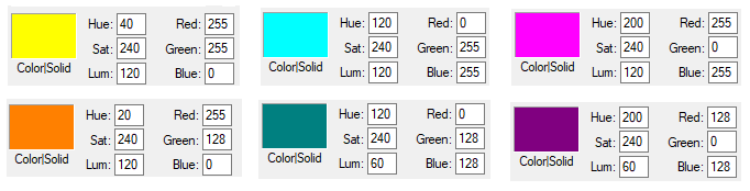Note that the RGB = [255 255 255] refers to 'white' colour and RGB = [0 0 0] denotes a perfectly 'black' colour. The dark gray colour has RGB value of [128 128 128].

As explained above, images are stored as pixels which are nothing but square boxes of size typicall 1/72 x 1/72 [in2] with colour intensity defined as RGB combination. However, the dimensions of a pixel are not fixed and is controlled by Pixels per Inch (PPI) of the device. Thus, size of pixel = physical size [inches] of the diplay / PPI of the display. Following pictures demonstrate the concept of pixels used in computer through an analogy of colour boxes used by the artist in MS-Excel.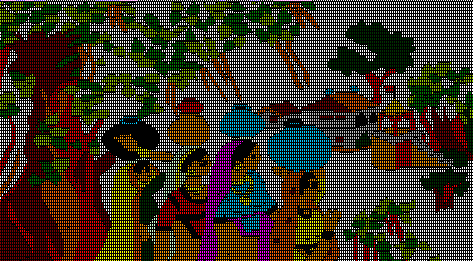The size of image can be checked either in "Microsoft Office Picture Manager" or MS-Paint. For example, the size of the following image is 577 x 76 [width x height]. The close-up view of the image is shown with all pixels clearly distinguished. The top-left 11 x 3 pixels have been converted to white colour manually.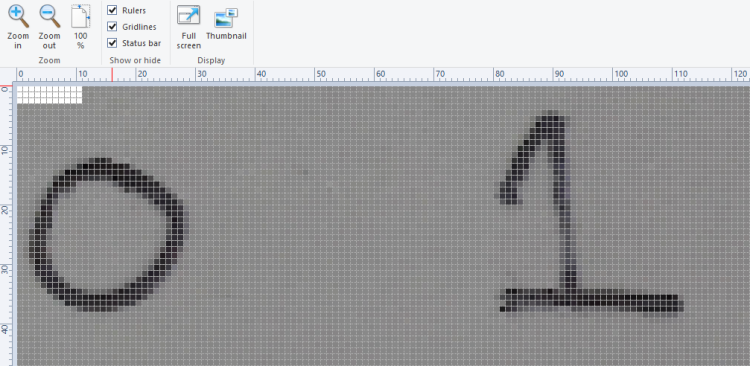The size of image reported in MS-Paint is shown below:NumPy and SciPy arrays of image objects store information as (H, W, D) order (also designated as axis=0, axis=1 and axis=2 respectively. The values can be transposed as img = transpose(-1, 0, 1) = (D, W, H) = transpose(2, 0, 1). Here, (H, W, D) can be access either by (0, 1, 2) or (-3, -2, -1). The EGBA format of image adds alpha channel to decribe opacity: α = 255 implies fully opaque image and α = 0 refers to fully transparent image. On a grayscape image, NumPy slicing operation img[:, 10:] = [0, 0] can be used to set 10 pixels on the right side of image to '0' or 'black'. img[:, :10] = [0, 0] sets 10 pixels on the left column to '0'.

The images when read in OCTAVE and pixel intensities converted into a text file results in following information. Note that the pixel intensity in text file is arranged by walking though the columns, that is the first 76 entries are pixels in first column in vertical direction.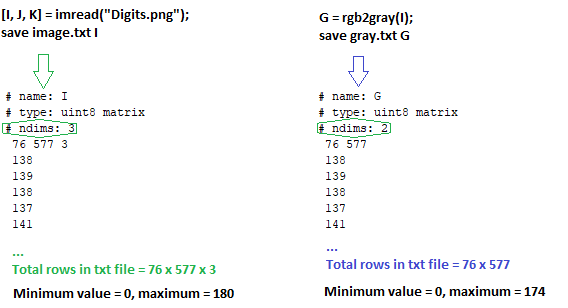Even though the text file contains one pixel intensity per row, the variables I and G are matrices of size 76 x 577 x 3 and 76 x 577 respectively. The rows with entries "76 577 3" and "76 577" are used to identify the size of the matrices.

As explained earlier, type uint8 stands for unsigned (non-negative) integers of size 8 bit and hence intensities are between 0 and 255. The image can be read back from text file using commands: load("image.txt"); imshow(I); Note the text file generated by this method contains few empty lines at the end of the file and should not be deleted. The text file should have at least one empty line to indicate EOF else it will result in error and the image will not be read successfully.
```warning: imshow: only showing real part of complex image
warning: called from
imshow at line 177 column 5```
Now, if the pixel intensities above 100 are changed to 255, it results in cleaned digits with sharp edges and white background. In OCTAVE, it is accomplished by statement x(x > 100) = 255. In Numpy, it is x[x > 100] = 255. You can also use & (and) and | (or) operator for more flexibility, e.g. for values between 50 and 100: OCTAVE: A((A > 50) & (A < 100)) = 255, Numpy: A[(A > 50) & (A < 100)] = 255.The image resulting with similar adjustment with lower cut-off intensity of 50: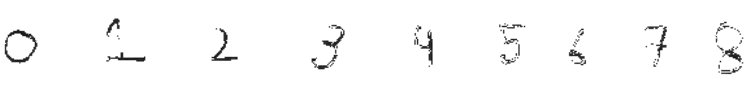The image resulting with similar adjustment with lower cut-off intensity of 128:Attributes of Image Data

The images has two set of attributes: data stored in a file and how it is displayed on a device such as projectors. There are terms such as DPI (Dots per Inch), PPI (Pixels per Inch), Resolution, Brightness, Contrast, Gamma, Saturation... This PDF document summarizes the concept of DPI and image size. An explanation of the content presented in the PDF document can be viewed in this video file.

The video can be viewed here.

Image File Types: PNG-8, PNG-24, JPG, GIF. PNG is a lossless format with option to have transparency (alpha channel). JPG files are lossy format and quality can be adjusted between 0 to 100%. JPG file cannot have transparency (alpha channel). PNG-8 or 8-bit version of PNG is similar to GIF format which can accomodate 256 colours and this format is suitable for graphics with few colours and solid areas having discrete-toned variation of colours. PNG-24 is equivalent to JPG and is suited for continous-toned images with number of colours > 256. In effect, a JPG file shall have lesser size (disk space) than PNG with nearly equal or acceptable quality of the image. Screenshots should be saved as PNG format as it will reproduce the image pixel-by-pixel as it appeared originally on the screen.

#### Image Filtering and Denoising

Image filtering is also known as Image Masking. The masking process is similar to a 'mask' or 'voer' we use for our body parts such as face. There are various types of noises that gets into scanned images or even images from digital camera. Gaussian, salt-and-pepper... are few of the names assigned to the type of noises.

Denoising using skimage, OpenCV: This Python code uses Total Variance method to denoise a image. This method works well for random Gaussian noises but may not yield good result for salt and pepper nose.

This code uses Non-Local Mean (NLM) Algorithm to denoise a image. This method works well for random Gaussian noises but may not yield good result for salt and pepper nose.

This Python script uses Median Blur and Histogram Equalization to denoise a coloured image. Filters that are designed to work with gray-scale images shall not work with colour images. scikit-image provides the adapt_rgb decorator to apply filters on each channel of a coloured image.

This is another code which uses Dilation, Blurring, Subtraction and Normalization to denoise an image and make the background white. This method is compared with Adaptive Threshold option available in OpenCV.

Image Thresholding: This is a process of converting pixel value above or below a threshold to an specified value. This operation can be used to segment an image. For example, a grayscale image can be converted to black-and-white by converting all pixels having intensity value ≤ 64 to 0.

The mask operation works on an input image and a mask image with logical operator such as AND, NAND, OR, XOR and NOT. An XOR (eXclusive OR) operation is true if and only if one of the two pixels is greater than zero, but both pixels cannot be > 0. The bitwise NOT function flips pixel values that is pixels that are > 0 are set to 0, and all pixels that are equal to 0 are set to 255. RGB = [255 255 255] refers to 'white' colour and RGB = [0 0 0] denotes a perfectly 'black' colour.

• AND: A bitwise AND is true (= 1 or 255) if and only if both pixels are > 0. In other words: white + anycolor = anycolor, black + anycolor = black.
• OR: A bitwise OR is true (=1 or 255) if either of the two pixels is > 0. With bitwise_or: white + anycolour = white, black + anycolour = anycolour.
• XOR: A bitwise XOR is true (=1 or 255) if and only if one of the two pixels is > 0, but not both are zero.
• NOT: A bitwise NOT inverts the on (1 or 255) and off (0) pixels in an image.
cv2.bitwise_and(img1, imag2, mask = None) can be used to overlap-merge two images in a single image. mask: 8-bit single channel array (that means only grayscale or black-and-white images), that specifies elements of the output array to be changed.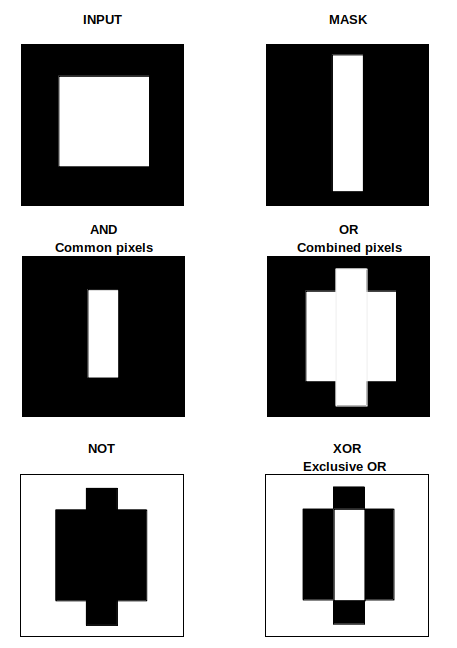Distance Masking: Determine the distance of each pixel to the nearest '0' pixel that is the black pixel.cv.add(img1, img2) is equivalent to numPy res = img1 + img2. There is a difference between OpenCV addition and Numpy addition. OpenCV addition is a saturated operation while Numpy addition is a modulo operation. cv.add(250, 25) = min(255, 275) = 255, np.add(250, 25) = mod(275, 255) = 20. Note there is no np.add function, used for demonstration purposes only.

 Input Image Mask Image Operation Outcome of Operation Binary or Grayscale Binary OR Pixels having value 0 in mask set to 0 in output, other pixels from input image retained Input Image Mask Image AND Pixels having value 0 in mask set to 1 or 255 in output, other pixels from input image retained

Circular Crop: This Python code uses OpenCV to create a circular crop of an image. The input image and cropped image are shown below.Alternatively, the image can be read into a NumPy array and pixels beyond each channel beyond the disk can be set to desired colour. This Python code uses OpenCV and NumPy array to create a circular crop of an image. The image is read using OpenCV, the BGR channels are extracted as NumPy arrays and then the pixels of each channel are set to white beyond the boundary of circular disk. Finally, BGR channels are merged to create the coloured image.

Connected Component Labeling: This Python script can be used to update an image background to white. This code uses Connected Component Labeling (CCL) method to remove the dark patches. The code customised for all images inside a folder can be found here. In case the text contains shadow in the background, the gray-scale image, the contrast has to be adjusted to accentuate the dark greys from the lighter greys - this can be achieved by imgGray = cv2.multiply(imgGray, 1.5) though the multiplier 1.5 used here needs to be worked out by trial-and-error. 1.1 is a recommended start value. Gaussian Blur and morphological operations such as erosion and dilation would be required to make the text sharper: kernel = np.ones((2, 1), np.uint8), img = cv2.erode(img, kernel, iterations=1). "Using Machine Learning to Denoise Images for Better OCR Accuracy" from pyimagesearch.com is a great article to exploit the power of ML to denoise images containing dominantly texts and noises.

Pixel Multiplication

Also known as Graylevel scaling (and not same as geometrical scaling), this operation can be used to brighten (scaling factor > 1) or darken (scaling factor < 1) an image. If the calculate value of pixel after multiplcation is > maximum allowed value, it is either truncated to the maximum value or wrapped-around the minimum allowed pixel value. For example, a pixel value of '200' when scaled by a fator 1.3, the new value of 260 shall get truncated to 255 or wrapped to 5 (= 260 - 255).

Morphological Operations

Image processing methods that transform images based on shapes are called Morphological Transformations. Erosion is the morphological operation that is performed to reduce the size of the foreground object. Dilaton is opposite of erosion. Thus, the thickness of fonts can be reduced using erosion and vice versa. Bright regions in an image tend to “get brighter” after Dilation, which usually results in an enhanced image. Removing noise from images is one of the application of morphological transformations. Morphological operators require Binary Images which are images whose pixels have only two possible intensity values. They are normally displayed as black and white and the two values are 0 for black, and either 1 or 255 for white.

Erosion is also known as minimum filter which replaces or removes objects smaller than the structure. Similarly, dilation is called maximum filter. A structuring element or kernel is a simple shape used to modify an image according to the shape locally fits or misses the image. Excerpt from docs.opencv.org: "During erosion a pixel in the original image (either 1 or 0) will be considered 1 only if all the pixels under the kernel is 1, otherwise it is eroded (made to zero)." Excerpt from pyimagesearch.com: "A foreground pixel in the input image will be kept only if all pixels inside the structuring element are > 0. Otherwise, the pixels are set to 0 (i.e. background)."

A key consideration while using morphological operations is the background colour of the image. Should it be white or black? Is the kernel definition dependent on whether background of image is white or black? docs.opencv.org recommends: "(Always try to keep foreground in white)". If you have an image with white background, in order to comply this recommendation, use whiteForeground = cv2.bitwise_not( blackForeground ) before erosion and then blackForeground = cv2.bitwise_not( whiteForeground ) after erosion. This short piece of code describes these steps.

```Rectangular Kernel: cv2.getStructuringElement(cv2.MORPH_RECT,(3,3))
array([[1, 1, 1],
[1, 1, 1],
[1, 1, 1]], dtype=uint8)

Elliptical Kernel: cv2.getStructuringElement(cv2.MORPH_ELLIPSE,(5,5))
array([[0, 0, 1, 0, 0],
[1, 1, 1, 1, 1],
[1, 1, 1, 1, 1],
[1, 1, 1, 1, 1],
[0, 0, 1, 0, 0]], dtype=uint8)

Cross-shaped Kernel: cv2.getStructuringElement(cv2.MORPH_CROSS,(3,3))
array([[0, 1, 0],
[1, 1, 1],
[0, 1, 0], dtype=uint8) ```

#### Animations using Python

Convert PNG to Animated GIF: Click here to get a Python script to convert a set of PNG file to animated GIF.

Animation of a sine wave. The Python code can be found here.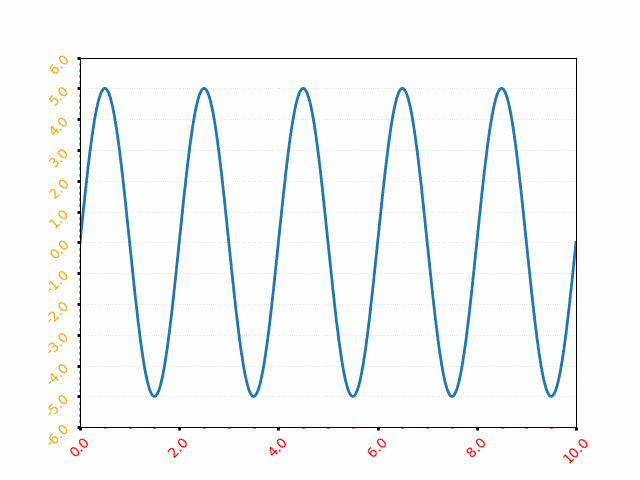###### Coordinate Transformation Matrices
If {X} is a row vector representing a 2D or 3D coordinates of a point or pixel, {X'} = [T] {X} where [T] is the transformation matrix. Thus:In general, the inverse of any pure rotation matrix, a matrix with determinant |T| = +1, is its transpose. Thus: [T]-1 = [T]Tr. Such matrices are said to be orthogonal and it is always easier to find transpose of a matrix than finding inverse of a matrix. Note that a 2D rotation in say XY plane occurs entirely in 2D plane about an axis normal to the plane (thus Z-axis in this case). However, a reflection is a 180° rotation about an axis in XY plane out into 3D space and back into 2D space. Additionally, if 2 pure reflection transformations about lines passing through the origin are applied in sequence, it will yield a pure rotation about the origin.

Rotation is assumed to be positive in right hand sense or the clockwise as one looks outward from the origin in the direction along the rotation axis. The righ hand rule of rotation is also expressed as: align the thumb of the right hand with the positive direction of the rotation axis. The natural curl of the fingers gives the positive rotation direction. Note the the x-coordinate of the position vector will not change if rotation takes place about x-axis, y-coordinate of the position vector will not change if rotation takes place about y-axis and so on.

Scaling: if a = d and b = c = 0, uniform scaling occurs. A non-uniform expansion or compression will result if a = d > 1 or a = d < 1 respectively. Scaling looks like an apparent translation because the position vectors (line connecting the points with origin) are scaled and not the points. However, if the centroid of the image or geometry is at the origin, a pure scaling without apparent translation can be obtained.

Homogeneous coordinates: in the tranformation matrices described in the table above, the origin of the coordinate system is invariant with respect to all of the transformation operations. The concept of homogenous coordinate system is used to obtain transformations about an arbitrary point. The homogenous coordinates of a nonhomogeneous position vector {X Y} are {X' Y' h} where X = X'/h and Y = Y'/h and h is any real number. Usually, h = 1 is used for convenience though there is no unique representation of a homogenous coordinate system. Thus, point (3, 5) can be represented as {6 10 2} or {9 15 3} or {30 50 10}. Thus, a general transformation matrix looks like shown below. Note that every point in a two-dimensional plane including origin can be transformation (rotated, reflected, scaled...).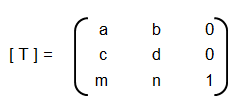A rotation about arbitray point will have the following sequence of matrix operations:The reflection about the lines that pass through origin is easy to obtain as summarized in the table above. Hoever, reflection of a point or any geometrical object about a line that does not pass through origin can be achieved by a combination of transformation operations.

[T] = [T'] [R] [R'] [R]-1 [T']-1

Thus, the steps are:
• Translate the line and the object both so that the line passes through the origin: the matrix [T'] in above concatenated matrix [T]
• Rotate the line and the object about the origin so that the line coincides with one of the coordiante axis: the matrix [R]
• Reflect the object about the line - the coordiante axis it coincides with: the matrix [R']
• Apply the inverse rotation aboue the origin using: the matrix [R]-1
• Apply the inverse translation to the original location: the marix [T]-1
Note the the transformation is obtained using matrix multiplication and hence the operation is non-commutative. That is the order of mutiplication affects the final result.
##### 3D Transformations
The three-dimensional representation and display of an object is necessary for the understanding of the shape of the object. Additionally, the abbility to translate (pan), rotate (dolly), scale (zoom), reflect and project help understand the shape of the object. Analogous to homogeneous coordinate system in 2D, a point in 3D space {X Y Z} can be represented by a 4D position vector {X' Y' Z' h} = {X Y Z 1} [T] where [T] is the transformation matrix similar to the one used in 2D case. A generalized 4 × 4 trasnformation matrix for 3D homogeneous coordiates is:Audio and Video Editing in OCTAVE, Python, FFmpeg and OpenShot
Like image, audio data is stored as matrix. The pixels and colourmap of an image is analogous to sample rate, the number of bits per sample (e.g. 8 or 16), and number of channels number (1 for mono, 2 for stereo...). Like imread, audioread reads the audio file filename and return the audio data y [the signal which can be a vector or a two-dimensional array] and sampling rate fs [Hz]. There are many terms or concepts associated with videos: keyframes, effects, transition, transform and animate to name few. Video effects is used modify the image and pixel data of a clip. As per OpenShot user guide, examples or types of 'Effects' are summarized below. Each media file that is added to the OpenShot timeline is called a clip. Transform: adjust the location, scale, rotation, and shear of a clip.
• Alpha Mask / Wipe Transition: Uses a grayscale mask image to gradually wipe / transition between 2 images. Alpha = Transarency
• Blur: Adjust the blur of the frame’s image
• Adjust the brightness and contrast of the frame’s image
• Chroma Key (Greenscreen): Replaces the color (or chroma) of the frame with transparency (i.e. keys out the color)
• Color Saturation: Adjust the color saturation
• Color Shift: Shift the colors of an image up, down, left, and right (with infinite wrapping)
• Crop: Crop out any part of your video
• Deinterlace: Remove interlacing from a video (i.e. even or odd horizontal lines)
• Hue: Adjust the hue / color of the frame’s image
• Negative: Negates the colors, producing a negative of the image
• Object Detector: Detect objects through the video
• Pixelate: Pixelate (increase or decrease) the number of visible pixels
• Shift the image up, down, left, and right (with infinite wrapping)
• Stabilizer: Stabilize video clip to remove undesired shaking and jitter
• Wave: Distort the frame’s image into a wave pattern
Similarly, transition as the name suggest indicates the method or style to switch beween one clip to another, to gradually fade (or wipe) between two clips. Thus, the most common location of a 'transition' is either at the start or end of the clip or image or keyframe. Direction of transitions adjust the alpha/transparency of the clip below it, and can either fade from opaque (dark) to transparent (default), or transparent to opaque.

Animation: the visual appearance of a video and animations are same and hence a video is an animation and an animation is a video - to human eyes. Thus, the option to animate in a Video Editing program may be confusing initially. The feature 'animation' refers to ability to change few keyframes in th clip or the video such as zoom, pan or slide.

Create a Zoom and Pan animation

• Step-1:Move the timeline where you want to begin to zoom
• Step-2: Right click on your clip, and choose Transform: this will display some interactive handles over the video preview
• Step-3: Drag the corner (while holding CTRL) to scale the image larger or smaller
• Step-4: Alternatively, use the Clip's properties to change the scale_x and scale_y, and location_x, and location_y properties
• Step-5: Drag the center circle handle to move the image
• Step-6: Move to the next position in the video, and repeat these steps.

Image Editing using ImageMagick

ImageMagick is nearly an universal tool to open any format of image and convert into another format. In MS Windows, once you have added ImageMagick installation folder location into 'Path' variable, use magick.exe mogrify -format jpg *.heic to convert all images in HEIC format to JPG format. To scale all PNG images in current folder: magick.exe mogrify -resize 540x360 "*" *.png. The option -resize 540x keeps the height in proportion to original image and -resize x360 keeps the width in proportion to original image. Option -resize 540x360 is equivalent to min(540x, x360).

ImageMagick provides two similar tools for editing and enhancing images: convert - basic image editor which works on one image at a time and mogrify - mostly used for batch image manipulation. Note that the output of both these two tools are not always the same.

##### Image and Video Editing using OpenCV

Convert images (PNG, JPG) to video (mp4, avi) - click on the link for Python script. To add the timer (time elapsed since video started playing), refer to this Python with OpenCV code. Timer can also be added using FFmpeg, scroll down for command line syntax. To add two videos side by side in width direction, refer to this Python + OpenCV code. Note that no padding (gap) between the two vidoes are added. To add two vidoes in vertical (up/down) direction, refer to this code. To add 4 videos in 2x2 box, refer to this Python + OpenCV code.

To add 3 videos in a 2x2 row with fourth video (bottom-right) as blank video (video with white background), refer this Python + OpenCV + numPy code. In case the location of fourth video needs to be replaced with an image, refer this Python + OpenCV. Note none of these codes check existence of input specified in the code. These codes can be improvised by adding checks for missing input and option to provide inputs from command line. In case you want to add partition line(s), you may use this code.

In case you are not able to play the video created after combining the 3 or 4 vidoes, try to scale down the input videos. The resultant height and width (twice the size of input videos) may not be displayed on the (laptop or computer) screen you are using.

Sometimes the frame rate per second (FPS) of the input videos needs to be adjusted to a common value. Use this Python + OpenCV code to change the FPS of a video.

Create Video by Rotating an Image: refer to this code.

##### Audio and Video Codecs

The audio data is stored as matrix with rows corresponding to audio frames and columns corresponding to channels. There are other utilities in OCTAVE such as create and use audioplayer objects, play an audio, write audio data from the matrix y to filename at sampling rate fs, create and use audiorecorder objects, scale the audio data and play it at specified sample rate to the default audio device (imagesc vs. audiosc)....

Audio Codec: The processing of audio data to encode and decode it is handled by an audio codec . Bit rate - The higher the bit rate, the higher the quality can be. Some audio codecs are: Advanced Audio Coding (AMC), MP3, Pulse Code Modulation (PCM) of Voice Frequencies (G.711)... Some terms associated with Audio data format and structure are: Sample Size, Channel, Channel Count, Audio Forms, Waveforms, Stereo (2 audio channels)

Video Encoding: In early days of digital video, video files were a collection of still photos. For a video recorded at 30 frames per second, 30 photos per second of footage has to be created and stored. Video encoding is the process of converting video files to a digital files so that they are not saved as collection of individual images but as fluid images. Some of the most popular encoding formats include: MP4, MOV, AVI, QuickTime.

Frame rate (frames per second or fps - note that the term 'rate' refers to per unit time in most of the cases) is rate at which images are updated on the screen. For videos, sample rate is number of images per second and for audios, sample rate is number of audio waves per second. Number of frames in a video = fps × duration of the video. The programs that are used for video file compression and playback are called codecs. Codec stands for coder and decoder. As in 2022, the best video codec is H.264. where other codecs available, including MPEG-2, HEVC, VP9, Quicktime, and WMV.

Programs to edit videos: FFmpeg, OpenShot, Blender, Windows Video Editor, Movie Maker (not supported beyond Windows-10). FFmpeg is a command-line tool (though few GUI do exist). As per the website ffmpeg.org: "A complete, cross-platform solution to record, convert and stream audio and video."
###### FFmpeg
Command line utilities: -i = specifies the input file stored in local disk or live audio/video source. -y = Overwrite output files without asking. ffmpeg provides the -map option for manual control of stream selection in each output file.

List of variables or aliases: in_h: height of input video, in_w: width of input video, dar = input display aspect ratio, it is the same as (w / h) * sar, line_h, lh = the height of each text line, main_h, h, H = the input height of vidoe, main_w, w, W = the input width of video, For images: iw = input width, ih = input height, ow = output width, oh = output height. n = the number of input frame, starting from 0, rand(min, max) = return a random number included between min and max, sar = The input sample aspect ratio, t = time-stamp expressed in seconds and equals NAN if the input timestamp is unknown, text_h, th = the height of the rendered text, text_w, tw = the width of the rendered text, x and y = the x and y offset coordinates where the text is drawn. These parameters allow the x and y expressions to refer to each other, so you can for example specify y=x/dar. They are relative to the top/left border of the output image. The default value of x and y is "0".

shadowx, shadowy = The x and y offsets for the text shadow position with respect to the position of the text. They can be either positive or negative values. The default value for both is "0". start_number = The starting frame number for the n/frame_num variable. The default value is "0".

• Increase audio level of a video: ffmpeg -i in.mp4 -filter:a "volume=4.0" out.mp4
• Convert video format: ffmpeg -i in.mkv -codec copy out.mp4
• Increase audio level and convert format together: ffmpeg -i in.mkv -filter:a "volume=2.0" out.mp4
• Add static text: ffmpeg -i inVid.mp4 -vf "drawtext = text ='Sampe Text':x = (w-text_w) / 2: y=0.9 * h-text_h/2: fontsize = 20: fontcolor = white" -c:a copy -y outVid.mp4 --- this adds text near the centre-left edge and overwrites any existing file created. fontsize=(h/30) can be used to make font relative to size of the video.
• Add static text: ffmpeg -i inVid.mp4 -vf "drawtext = textfile = Credit.txt :x = (w-text_w) / 2: y=0.9 * h-text_h/2: fontsize = 20: fontcolor = white: box=1: boxcolor=black@0.50: boxborderw=5" -c:a copy -y outVid.mp4 --- this adds a textbox of background color 'black' and transpareny 0.50. Note that textbox does not automatically wrap the text, subtitles filter does. In order to use textboxes with libe breaks, use a text file and add newline there.
• File Options:
• -f fmt: force format specified by 'fmt' where Input Devices are - lavfi = Libavfilter input virtual device, openal, oss = Open Sound System input device, pulse = PulseAudio input device, 'Video4Linux2' input video device, x11grab = X11 video input device ...
• -f fmt: force format specified by 'fmt' where Output Devices are - alsa = (Advanced Linux Sound Architecture) output device, opengl, oss = Open Sound System output device, pulse = PulseAudio output device ...
• -t duration: record or transcode 'duration' seconds of audio/video
• -to time_stop: record or transcode stop time
• Video options:
• -ab bitrate: audio bitrate (use -b:a)
• -b bitrate: video bitrate (use -b:v)
• -dn: disable data
• -c[:stream_specifier] - select an encoder (when used before an output file) or a decoder (when used before an input file) for one or more streams. codec is the name of a decoder/encoder or a special value copy (output only) to indicate that the stream is not to be re-encoded
• -vframes number: set the number of video frames to output
• -r rate: set frame rate (Hz value, fraction or abbreviation)
• -s size: set frame size (WxH or abbreviation)
• -aspect aspect: set aspect ratio (valid entries are "4:3", "16:9" or "1.3333", "1.7777" including double quotes) - floating point number string, or a string of the form num:den, where num and den are the numerator and denominator of the aspect ratio
• -bits_per_raw_sample: number set the number of bits per raw sample
• -vn: disable video
• -vcodec codec: force video codec ('copy' to copy stream)
• -timecode hh:mm:ss[:;.]ff set initial TimeCode value
• -pass n: select the pass number (1 to 3)
• -pix_fmt[:stream_specifier]: Set pixel format
• -vf filter_graph: set video filters where -vf and -filter:v are equal
• Audio options:
• -aframes number: set the number of audio frames to output
• -aq quality: set audio quality (codec-specific)
• -ar rate: set audio sampling rate (in Hz)
• -ac channels: set number of audio channel -> Convert stereo (not suitable for mobiles without earphones) to mono (suitable for mobiles) - ffmpeg -i in_stereo.mp4 lac -ac 1 out_mono.mp4
• -an: disable audio - ffmpeg -i inpVid.mp4 -c copy -an outVid.mp4
• -vol volume: change audio volume (256=normal)
• -af filter_graph: set audio filters
• Subtitle options: The subtitles video filter can be used to hardsub, or burn-in, the subtitles. This requires re-encoding and the subtitles become part of the video itself. Softsubs are additional streams within the file. The player simply renders them upon playback.
• -s size: set frame size (WxH or abbreviation)
• -sn: disable subtitle
• -scodec codec: force subtitle codec ('copy' to copy stream)
• -stag fourcc/tag: force subtitle tag/fourcc
• -fix_sub_duration: fix subtitles duration
• -canvas_size size: set canvas size (WxH or abbreviation)
• -spre preset: set the subtitle options to the indicated preset
• ffmpeg -i in.mp4 -vf "subtitles = subs.srt:force_style = 'FontName = Arial, FontSize=24'" -vcodec libx264 -acodec copy -q:v 0 -q:a 0 -y outSubs.mp4
• Filter options:
• The nullsrc video source filter returns unprocessed (i.e. "raw uninitialized memory") video frames. It can be used as a foundation for other filters, as the source for filters which ignore the input data.

Sometime, you may create unknowingly a video that does not play audio on mobile devices, but works fine on desktops or laptops. Sometimes the audio can be heard in mobile using earphones but sometimes not at all (even using earphones). The reason is that desktop clients use stereo (two channels), and the mobile clients use mono (single channel). Video with stereo tracks can be played in case mono track is emulated correctly. When a mono audio file is mapped to play a stereo system, it is expected to play the one channel of audio content equally through both speakers.

Extract Audio

ffmpeg -i in.mp4 -vn -acodec copy out.m4a -Check the audio codec of the video to decide the extension of the output audio (m4a here). From stackoverflow.com: If you extract only audio from a video stream, the length of the audio 'may' be shorter than the length of the video. To make sure this doesn't happen, extract both audio and video simultaneously: ffmpeg -i in.mp4 -map 0:a Audio.wav -map 0:v vidNoAudio.mp4 -As a good practice, specify "-map a" to exclude video/subtitles and only grab audio. Note that *.MP3 and *.WAV support only 1 audio stream. To create a muted video: ffmpeg -i in.mp4 -c copy -an vidNoAudio.mp4 or ffmpeg -i in.mp4 -map 0:v vidNoAudio.mp4

To create an mp3 file, re-encode audio: ffmpeg -i in.mp4 -vn -ac 2 out.mp3

Merge an audio to a video without any audio: ffmpeg -i vidNoAudio.mp4 -i Audio.wav -c:v copy -c:a aac vidWithAudio.mp4

Extract one channel from a video with stereo audio: ffmpeg -i in.mp4 -af "pan=mono|c0=c1" mono.m4a

To address the case where a video does not play audio on mobile devices but works fine on desktops, follow these steps: 1. Extract one channel from the video 2. Remove audio from the video - in order words mute the original video 3. Finally merge the audio extracted in step-1 with muted video created in step-2.

Simple Rescaling: ffmpeg -i in.mp4 -vf scale=800:450 out.mp4 --- To keep the aspect ratio, specify only one component, either width or height, and set the other component to -1: ffmpeg -i in.mp4 -vf scale=800:-1 out.mp4

Add Ripple and Wave Effects: Displace pixels of a source input by creating a displacement map specified by second and third input stream

Ripple:

ffmpeg -i in.mp4 -f lavfi -i nullsrc=s=800x450, lutrgb=128:128:128 -f lavfi -i nullsrc=s=800x450, geq='r=128 + 30*sin(2*PI*X/400+T) : g=128 + 30*sin(2*PI*X/400+T) : b=128 + 30*sin(2*PI*X/400+T)' -lavfi 'displace' -c:a copy -y outRipple.mp4 --- the size (800x450 in this case) needs to be checked in the source video and specified correctly.

Wave:

ffmpeg -i in.mp4 -f lavfi -i nullsrc=s=800x450, geq='r=128 + 80*(sin(sqrt((X-W/2) * (X-W/2)+(Y-H/2) * (Y-H/2))/220*2*PI+T)) : g=128 + 80*(sin(sqrt((X-W/2) * (X-W/2)+(Y-H/2) * (Y-H/2))/220*2*PI+T)):b=128 + 80*(sin(sqrt((X-W/2) * (X-W/2)+(Y-H/2) * (Y-H/2))/220*2*PI+T))' -lavfi 'split[x][y], [x][y]displace' -y outWave.mp4

The references, credits and other information can be added to videos using text boxes and subtitles. ffmpeg -i inVid.mp4 -vf "drawtext = textfile ='Credits.txt':x = (w-1.2*text_w): y=0.5 * h-text_h/2: fontsize = 32: fontcolor = white" -c:a copy -y outVid.mp4 --- adds a text box near the centre-right location of the video.

To add subtitles, a SubRip Text file needs to be create with each sections defined as described below:
1. A number or counter: it indicates the position of the subtitle
2. Start and end time of the subtitle separated by '–>' characters, end time can be > the total duration of the video
3. Subtitle text in one or more lines, followed by a blank line indicating the end of the subtitle
4. The formatting of SRT files is derived from HTML tags though curly braces can be used instead of <...>. Example: <b>...</b> or {b}... {/b}, 'b' can be replaced with 'i' or 'u' for italic or underlined fonts. Font Color <font color= "white"> ... </font>. Line Position {\a5} (indicates that the text should start appearing on line 5).
Example:
```1
00:00:00:00 --> 00:01:30:00
This video is about usage of FFmpeg to edit videos without any cost

```
ffmpeg -i inVid.mp4 -vf "subtitles=subs.srt:force_style='Alignment=10, FontName = Arial, FontSize=24, PrimaryColour = &H0000ff&'" -vcodec libx264 -acodec copy -q:v 0 -q:a 0 -y outSubs.mp4 --- Colour Code: H{aa}{bb}{gg}{rr} where aa refers to alpha or transparency, bb, gg and rr stands for BGR channel. The values are hexadecimal numbers: 127 = 16 x 7 + 11 = 7A, 255 = 16 x 15 + 15 = FF. Thus: &H00000000 is BLACK and &H00FFFFFF is WHITE

Subtitles in SubStation Alpha Subtitles file (ASS) format: ffmpeg -i inVid.mp4 -filter_complex "subtitles=Sample.ass" -c:a copy -y outAssSub.mp4 - Click on the link to get a sample ASS file.

Following code uses a blank image of size 360x180 and add the text in defined in Typewritter.ass file to create a video of duration 10 [s]: ffmpeg -f lavfi -i color=size=360x180:rate=30:color=white -vf "subtitles=Typewriter.ass" -t 10 -y TypewriterEffect.mp4

Typewritter Effect using OpenCV and Python: refer to this file which is well commented for users to follow the method adopted.

ffmpeg -i in.mp4 -vf "[in]drawtext=text='The': fontcolor= orange: fontsize=100: x=(w-text_w)/2+0: y=0:enable= 'between(t, 0, 5)', drawtext = text = 'Typewritter': fontcolor= orange: fontsize=100: x=(w-text_w)/2+20: y=text_h: enable='between(t, 1, 5)', drawtext = text = 'Effect': fontcolor= orange: fontsize=100:x=(w-text_w)/2+40: y=2.5*text_h:enable= 'between(t, 2, 5)' [out]" -y vidTypeWritter.mp4

Add a scrolling text from left-to-right

ffmpeg -y -i inpVid.mp4 -vcodec libx264 -b:a 192k -b:v 1400k -c:a copy -crf 18 -vf "drawtext= text=This is a sample text added to test video :expansion= normal:fontfile= foo.ttf: y=h-line_h-10:x=(5*n): fontcolor=white: fontsize=40: shadowx=2: shadowy=2" outVid.mp4 ---Note that the text is added through option -vf which stands for video-filter. no audio re-encoding as indicated by -c:a copy. The expression x=(5*n) positions the X-coordinate of text based on frame number. x=w-80*t (text scrolls from right-to-left) can be used to position the test based on time-stamp of the video. x=80*t makes the text scroll from left-to-right. For example: ffmpeg -y -i inpVid.mp4 -vcodec libx264 -b:a 192k -b:v 1400k -c:a copy -crf 18 -vf "drawtext= text=This is a sample text added to test video :expansion= normal:fontfile= Arial.ttf: y=h-line_h-10:x=80*t: fontcolor=white: fontsize=40" outVid.mp4

ffmpeg -i inVid.mp4 -vf "[in]drawtext = text ='Text on Centre-Left':x = (0.6*text_w): y=0.5 * h-text_h/2: fontsize = 32: fontcolor = black, drawtext = textfile ='Credits.txt':x = (w-1.2*text_w): y=0.5 * h-text_h/2: fontsize = 32: fontcolor = white[out]" -c:a copy -y outVid.mp4 --- Everything after the [in] tag (up to [out] tag) applies to the main source.

ffmpeg -y -i inVid.mp4 -filter_complex "split[base][text];[text]drawtext=textfile='Credits.txt': fontcolor=white: fontsize=32: x=text_w/2:y=(h-text_h)/2, format=yuva444p, fade=t=in:st=1:d=5:alpha=1, fade=t=out:st=10:d=5:alpha=1[subtitles]; [base][subtitles]overlay" -y outVid.mp4

ffmpeg -i inVid.mp4 -vf "drawtext=textfile ='Credits.txt': fontcolor=white: fontsize=32: x=w-text_w*1.1: y=(h-text_h)/2 : enable= lt(mod(n\,80)\, 75)" -y outBlink.mp4 --- To make 75 frames ON and 5 frames OFF, text should stay ON when the remainder (mod function) of frame number divided by 80 (75 + 5) is < 75. enable tells ffmpeg when to display the text

Loop: x=mod(max(t-0.5\,0)*(w+tw)/7.5\,(w+tw)) where t-0.5 indicates that scolling shall start after 0.5 [s] and 7.5 is duration taken by a character to scroll across the width. In other words, text shall scroll across the video frame in fixed number of seconds and you will not get constant speed regardless of the width of the video. As you can see, x=w-f(t,w..) makes the scrolling from right to left.

R-2-L: ffmpeg -y -i inpVid.mp4 -vcodec libx264 -b:a 192k -b:v 1400k -c:a copy -crf 18 -vf "drawtext= text = This is a sample text added to test video :expansion=normal: fontfile=Arial.ttf: y=h/2-line_h-10: x=if(eq(t\,0)\,w\, if(lt(x\,(0-tw))\,w\,x-4)): fontcolor=white: fontsize=40" outVid.mp4. x=if(eq(t\,0)\,(0-tw)\, if(gt(x\,(w+tw))\,(0-tw)\,x+4)) should be used for L-2-R.

Alternatively: x=if(gt(x\,-tw)\,w - mod(4*n\,w+tw)\,w) for R-2-L and x=if(lt(x\,w)\, mod(4*n\,w+tw)-tw\,-tw) for L-2-R can be used.

Add a scrolling text from right-to-left where text is stored in a file

ffmpeg -i in.mp4 -vf "drawtext= textfile=scroll.txt: fontfile=Arial.ttf:y=h-line_h-10:x= w-mod(w*t/25\, 2400*(w+tw)/w): fontcolor=white: fontsize=40: shadowx=2: shadowy=2" -codec:a copy output.mp4 ---Note that \, is used to add a comma in the string drawtext. The text to be scrolled are stored in the file scroll.txt, in the same folder where in.mp4 is stored. Place all lines on a single line in the file.

Merge or Concatenate Videos

Note that following examples assume that all the videos contain audio and are of same size. All video streams should have same resolution. While concatenating audios, all video inputs must be paired with an audio stream. If any video doesn't have an audio, then a dummy silent track has to be used.

Merge 2 videos: ffmpeg -i v1.mp4 -i v2.mp4 -filter_complex "[0:v:0] [0:a:0] [1:v:0] [1:a:0] concat=n=2:v=1:a=1 [v] [a]" -map [v] -map [a] cat2.mp4

Merge 3 videos: ffmpeg -i v1.mp4 -i v2.mp4 -i v3.mp4 -filter_complex "[0:v:0] [0:a:0] [1:v:0] [1:a:0] [2:v:0] [2:a:0] concat=n=3:v=1:a=1 [v] [a]" -map [v] -map [a] -y cat3.mp4. For videos without an audio: ffmpeg -i 1.mp4 -i 2.mp4 -i 3.mp4 -filter_complex "[0:v] [1:v] [2:v] concat=n=3:v=1:a=0" -y cat3.mp4

Merge 5 videos with audio:ffmpeg -i 1.mp4 -i 2.mp4 -i 3.mp4 -i 4.mp4 -i 5.mp4 -filter_complex "[0:v] [1:v] [2:v] [3:v] [4:v] concat=n=5:v=1:a=0" -y cat5.mp4

Merge 2 videos after scaling: ffmpeg -i v1.mp4 -i v2.mp4 -filter_complex "[0:v:0]scale=960:540[c1]; [1:v:0]scale=960:540[c2], [c1] [0:a:0] [c2] [1:a:0] concat=n=2:v=1:a=1 [v] [a]" -map "[v]" -map "[a]" -y scat.mp4

Merge 2 videos after scaling - the second video contains no audio: ffmpeg -i v1.mp4 -i v2.mp4 -f lavfi -t 0.01 -i anullsrc -filter_complex "[0:v:0]scale=960:540[c1]; [1:v:0]scale=960:540[c2], [c1] [0:a:0] [c2] [2:a] concat=n=2:v=1:a=1 [v] [a]" -map "[v]" -map "[a]" -y cat2.mp4 ---Note: the value of -t (in this example 0.01 second) have to be smaller or equal than the video file you want to make silence otherwise the duration of -t will be applied as the duration for the silenced video. [2:a] in this case means the second input file does not have an audio (the counter starts with zero).

Add progress time-stamp at top-right corner in HH:MM:SS format --- ffmpeg -i in.mp4 -vf "drawtext=expansion=strftime: basetime=\$(date +%s -d'2020-12-01 00:00:00')000000: text='%H\\:%M\\:%S'" -y out.mp4 where \\: is used to escape the : which would otherwise get the meaning of an option separator. strftime format is deprecated as in version 4.2.7.

Another method that require some formatting of the time is: ffmpeg -i in.mp4 -vf drawtext="fontsize=14:fontcolor=red:text='%{e\:t}':x=(w-text_w):y=(h-text_h)" -y out.mp4

Sequences of the form %{...} are expanded. The text between the braces is a function name, possibly followed by arguments separated by ':'. If the arguments contain special characters or delimiters (':' or '}'), they should be escaped such as \: to escape colon. The following functions are available:

• expr, e: The expression evaluation result.
• expr_int_format, eif: Evaluate the expression's value and output as formatted integer. The first argument is the expression to be evaluated, just as for the expr function. The second argument specifies the output format. Allowed values are 'x', 'X', 'd' and 'u'. They are treated exactly as in the printf function. The third parameter is optional and sets the number of positions taken by the output. It can be used to add padding with zeros from the left.
• pts: The timestamp of the current frame. It can take up to three arguments. The first argument is the format of the timestamp; it defaults to flt for seconds as a decimal number with microsecond accuracy; hms stands for a formatted [-]HH:MM:SS.mmm timestamp with millisecond accuracy. gmtime stands for the timestamp of the frame formatted as UTC time;

Put the time-stamp at bottom-right corner: ffmpeg -i in.mp4 -vf drawtext="fontsize=14:fontcolor=red:text='%{eif\:t\:d} \[s\] ':x=(w-text_w):y=(h-text_h)" -y out.mp4

Crop and Cut or Trim Videos

There is a difference in between Crop and Trim operations. Crop refers to spatial trimming whereas Cut or Trim refers to timestamp trimming. Following lines of code shall fail if the dimension of new video exceeds beyond the dimensions of original video. The crop filter will automatically center the crop location if starting position (x, y) are omitted.

Crop a video starting from x = 50 and y = 75 with new dimension of video as 320x180: ffmpeg -i in.mp4 -filter:v "crop=320:180:50:75" -c:a copy cropped.mp4

Crop a video starting from bottom left corner with new dimension of video as 480x270: ffmpeg -i in.mp4 -filter:v "crop=320:180:0:in_h" -c:a copy -y cropped.mp4

Crop left-half of a video: ffmpeg -i in.mp4 -filter:v "crop=in_w/2:in_h:0:in_h" -c:a copy -y cropL.mp4

Crop left-half of a video: ffmpeg -i in.mp4 -filter:v "crop=in_w/2:in_h:in_w/2:in_h" -c:a copy -y cropR.mp4

Cut a video from specified start point and duration: ffmpeg -i in.mp4 -ss 00:01:30 -t 00:02:30 -c:v copy -c:a copy trimmed.mp4 -Here '-ss' specifies the starting position and '-t' specifies the duration from the start position. As explained earlier "-c:v copy" and "-c:a copy" prevent re-encoding while copying. "-sseof 180" can be used to cut the last 180 seconds from a video.

Overlay two videos side-by-side: ffmpeg -i cropL.mp4 -i cropR.mp4 -filter_complex hstack -c:v libx264 -y overLay.mp4

Overlay two videos side-by-side creating a video larger than the combined size of input videos: ffmpeg -i cropL.mp4 -vf "movie=cropR.mp4 [in1]; [in]pad=640*2:450[in0]; [in0][in1] overlay=600:0 [out]" -y newOverlay.mp4 -Here new video has size [W x H] = 640*2:450 and the second video is placed at X = 600. Ensure that the new dimension on new video is able to contain both the videos.

Overlay a logo (image) on a video for specified duration: ffmpeg -i in.mp4 -i Logo.png -filter_complex "[0:v][1:v] overlay=W-50:25:enable='between(t,0,20)'" -pix_fmt yuv420p -c:a copy -y out.mp4 -> enable='between(t,0,20)' means the image shall be shown between second 0 and 20.

W is an FFmpeg alias for the width of the video and w is the alias for the width of the image being overlaid. Ditto for H and h. These can also be referred to as main_w (or _h) and overlay_w (or _h). "-itsoffset 10" can be used to delay all the input streams by 10 second. If the input file is 120 seconds long, the output file will be 130 seconds long. The first 10 seconds will be a still image (first frame). A negative offset advances all the input streams by specified time. This discards the last 10 seconds of input. However, if the input file is 120 seconds long, the output file will also be 120 seconds long. The last 10 seconds will be a still image (last frame). ffmpeg -i in.png -vf scale=iw*2:ih*2 out.png scales the image two-times the original dimensions.

Overlay multiple images on a video each for different time durations: ffmpeg -i in.mp4 -i Img-1.png -i Img-2.jpg -i Img-3.jpg -filter_complex "overlay= enable='between(t,0,15)':x=0:y=0[out]; [out]overlay= enable='between(t,30,60)': x=0:y=0[out]; [out]overlay= enable='between(t,75,90)': x=0:y=0[out]" -map [out] -map 0:a -acodec copy -y out.mp4 ->Make dure that the video duration is not exceeded while specifiying duration of overlay. To make the images appear on the top-right corner, replace x=0 with x=W-w.

Pillarboxing:

Reference: superuser.com/questions/547296/... Scale with pillarboxing (the empty space on the left and right sides are filled with specified colour). Letterboxing is when empty space all around the image is filled with specified colour.

Crop the excess area:

force_original_aspect_ratio = disable: Scale the video as specified and disable this feature.

ffmpeg -i in.png -vf "scale=800:450: force_original_aspect_ratio=increase, crop=800:450" -y out_crop.png

Place a still image before the first frame of a video: Reference stackoverflow.com/questions/24102336...

ffmpeg -loop 1 -framerate 25 -t 5 -i img.png -t 5 -f lavfi -i aevalsrc=0 -i in.mp4 -filter_complex "[0:0] [1:0] [2:0] [2:1] concat=n=2:v=1:a=1" -y out.mp4 -> this assumes that the size of image and video are same.

-loop 1 -framerate FPS -t DURATION -i IMAGE: this basically means: open the image, and loop over it to make it a video with DURATION seconds with FPS frames per second. The reason you need it to have the same FPS as the input video is because the concat filter we will use later has a restriction on it.

-t DURATION -f lavfi -i aevalsrc=0: this means - generate silence for DURATION (aevalsrc=0 means silence). Silence is needed to fill up the time for the splash image. This isn't needed if the original video doesn't have audio.

-filter_complex '[0:0] [1:0] [2:0] [2:1] concat=n=2:v=1:a=1': this is the best part. You open file 0 stream 0 (the image-video), file 1 stream 0 (the silence audio), file 2 streams 0 and 1 (the real input audio and video), and concatenate them together. The options n, v, and a mean that there are 2 segments, 1 output video, and 1 output audio.

Zoom-Pan Image into a Video:

The simplest version without any scaling of the input image and zoom-pan around top left corner - ffmpeg -loop 1 -i image.png -filter_complex "zoompan= z= 'zoom+0.002': x=0:y=0:d=250:fps=25[out]" -acodec aac -vcodec libx264 -map [out] -map 0:a? -pix_fmt yuv420p -r 25 -t 4 -s "800x640" -y zoopTopLeft.mp4 - The value 0.002 is zoom factor which can be increased or decreased to make the zoom effect faster or slower. d=250 is the duration (number of frames) of zooming process and -t 4 is the duration of the output video. Change x=0:y=0 to x=iw:y=ih for zoom-pan about bottom right corner. Note that zoompan, by default, scales output to hd720 that is 1280x720 (and at 25 fps).

ffmpeg -loop 1 -i image.png -vf "scale = iw*2:ih*2, zoompan=z='if(lte(mod(on,100), 50),zoom+0.002, zoom-0.002)':x ='iw/2-(iw/zoom)/2': y='ih/2-(ih/zoom)/2': d=25*5: fps=25" -c:v libx264 -r 25 -t 4 -s "800x640" -y zoomInOut.mp4 - In each 100-frame cycle, this will zoom in for first 50 frames, and zoom out during the rest. For just 1 zoom-in and zoom out event, adjust the values based on duration and frame rate per second (-t 4 and -r 25 respectively in this example). While running this you may get the message "Warning: data is not aligned! This can lead to a speed loss" though the output video shall get generated without any issue. In case you do not want to scale the video, remove -s "800x640". The option scale = iw*2:ih*2 scales the image before zoom-pan. It is recommended to set the aspect ratio of zoom-pan equal to that of the image.

The zoom-in and zoom-out operation described above can also be performed in OpenCV + Python. The sample code can be found here. The outputs shall look like shown below.

Animations like PowerPoint

This Python and OpenCV code is intended to create functions to generate the animations available in Microsoft PowerPoint. The first category of aniations are [Wipe, Split, Fly In, Float In, Rise Up, Fly Out, Float Down, Peek In, Peek Out]. All of these look similar and they differ in speed and direction of entrance. The other set is [ Shape, Wheel, Cicle, Box, Diamond ] where the image needs to be in non-orthogonal directions. The third set of animation is [Stretch, Compress, Zoom, Glow and Turn, Pin Wheel] - all of these operations are performed on entire image. The animations in PowerPoint are categories by Entrance, Emphasis and Exit.

Another example to animate the images by Split in Vertical direction is shown below. The Python + OpenCV code can be downloaed from this link.

Animations of images by split in diagonal directions are shown below.

The Python + OpenCV code can be downloaed from this link.

As you can see, the animation stops at the diagonal line starting from TOP-LEFT corner that is element [0, 0] of the arrays. This code can be used to create animation from BOTTOM-LEFT to TOP-RIGHT edge of the image and vice versa.

By design, the lower and upper triangulation is implement by considering diagonal created from top-left corner to bottom-right corner of the array. Hence, the array flip operation can be used to create animation from bottom-left to top-right corner. This Python + NumPy + OpenCV code contains 4 functions to create animations from the 4 corners of an image. Sample output is also shown in the video below.

Rotate Image in Openshot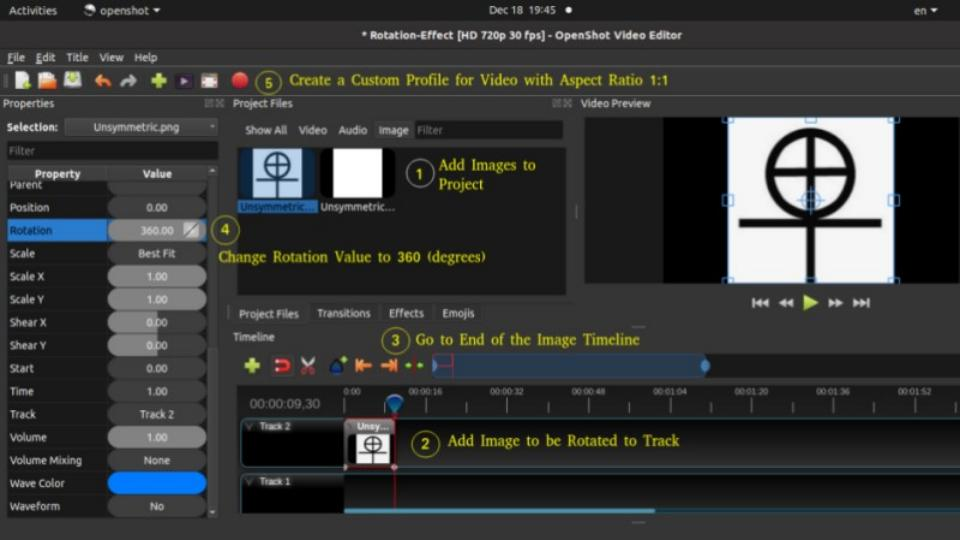Create a custom profile to create a video with aspect ratio 1:1 with following lines of statements. Note that for rotation effect to have circular symmetry, you need to have the rotation in 1:1 aspect ratio. This file needs to be save in ~/.openshot_qt/profiles folder.
```description=Aspect_ratio_1 - Name of new profile
frame_rate_num=30000       - Frame rate numerator
frame_rate_den=1000        - Frame rate denominator
width=310                  - Width of the video
height=310                 - Height of the video
progressive=1              - 1 = both even and odd rows of pixels used
sample_aspect_num=1        - Numerator of pixel shape aspect ratio
sample_aspect_den=1        - Denominator of pixel shape aspect ratio
display_aspect_num=16      - Numerator of display aspect ratio
display_aspect_den=9       - Denominator of display aspect ratio```

The output shall look like as shown below. Note that when a square a rotated, its corners shall get trimmed as maximum dimensions (the diagonal) exceeds the width of the video.

In order to remove the corner-trimming effect while rotating an image, follow the steps described in image below.If you look closely, the light gray background of square image is preventing a symmetric circular view. Hence the background of both the images should be white or of same colour. Alternatively, you can draw a white circle around the image being rotated and change the background of all the pixels out of this white circular boundary to white.

This rotation effect can also be created using this code in Python and OpenCV.

##### Markov Chain and Hidden Markov Models (HMM)

As per MathWorks Inc: Markov processes are examples of stochastic processes - processes that generate random sequences of outcomes or states according to certain probabilities.

Also known as "time series analysis", this model is in many aspects similar to Naive-Bayes model and in fact based on Bayes theorem. HMM is used to find a likely sequence of events for a given sequence of observations. Here the probability of a future event is estimated based on relative frequency of past observations of sequence of events (thus known prior probabilities). Probabilities to go from state 'i' to state 'i+1' is known as transition probability. The emission probability refers to the likelihood of of a certain observation 'y' when model is in state 's'.

Markov Chain: P(En|En-1, En-1 ... E2, E1) = probability of nth event given known outcome of past (n-1) events.

First Order Markov Assumption: P(En|En-1, En-1 ... E2, E1) = P(En|En-1) that is probability of nth event depends only of known outcome of previous event. This is also known as "memoryless process" because the next state depends only on the current state and not on the chain of events that preceded it or led the latest state. This is similar to tossing a fair coin. Even if one gets 5 or 20 successive heads, the probability of getting a head in next toss is still 0.50.

Markov first order assumption may or may not be valid depending upon the application. For example, it may not be a valid assumption in weather forecasting and movement of stock price. However, it can be a valid assumption in prediction of on-time arrival of a train or a flight.

Trellis Diagram: This is a graphical representation of likelihood calculations of HMMs.

Example calculations:

• Suppose the initial or prior probabilities of 'clear' and 'foggy' day during December-January in northern part of India are: P(C) = 0.3, P(F) = 0.7.
• The transition probabilities are: P(C|C) = 0.2, P(C|F) = 0.1, P(F|F) = 0.6, P(F|C) = 0.5
• Probability of a sequence of states in this example say P({F, F, C, C}) = P(C|C) × P(C|F) × P(F|F) × P(F) = 0.2 × 0.1 × 0.6 × 0.7 = 0.0084
Anomaly Detection

The following OCTAVE script implements a Gaussian model to detect anomalous examples in a given dataset. The Gaussian distribution is mathematically represented as follows. The data in a CSV file used for cross-validation can be downloaded from here.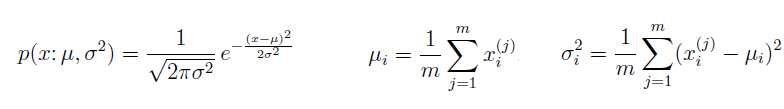```%-------------------------------------------------------------------------------
%----Ref: github.com/trekhleb/machine-learning-octave/anomaly-detection/--------
%Anomaly detection algorithm to detect anomalous behavior in server computers.
%The features measure the throughput (Mb/s) and latency (ms) of response of each
%server. m = 307 examples of how they were behaving, the unlabeled dataset. It
%is believed that majority of these data are normal or non-anomalous examples of
%the servers operating normally, but there might also be some examples of servers
%acting anomalously within this dataset. Label y = 1 corresponds to an anomalous
%example and y = 0 corresponds to a normal example.
%-------------------------------------------------------------------------------
clear; close all; clc;
%
X = [A(:, 1) A(:, 2)]; Y = A(:, 3);
%
%Estimate MEAN and VARIANCE: parameters of a Gaussian distribution
%Get number of training sets and features. size(X) returns a row vector with the
%size (number of elements) of each dimension for the object X. m=rows, n=cols
[m n] = size(X);
mu = mean(X);
s2 = (1 / m) * sum((X - mu) .^ 2);
%
%-------------------------------------------------------------------------------
%Visualize the fit
[X1, X2] = meshgrid(0 : 0.5 : 30);  U = [X1(:) X2(:)];
[m n] = size(U);
%
%Returns the density of the multivariate normal at each data point (row) of X
%Initialize probabilities matrix
Z = ones(m, 1);
%
%Go through all training examples and through all features. Returns the density
%of the multivariate normal at each data point (row) of X.
%
for i=1:m
for j=1:n
p = (1 / sqrt(2 * pi * s2(j))) * exp(-(U(i, j) - mu(j)) .^ 2 / (2 * s2(j)));
Z(i) = Z(i) * p;
end
end
Z = reshape(Z, size(X1));
%
%Visualize training data set.
plot(X(:, 1), X(:, 2),'bx'); hold on;
%
%Do not plot if there are infinities
if (sum(isinf(Z)) == 0)
contour(X1, X2, Z, 10 .^ (-20:3:0)');
end
hold off;
xlabel('Latency (ms)'); ylabel('Throughput (MB/s)');
title('Anomaly Detection: Server Computers');
%
%-------------------------------------------------------------------------------
%Returns the density of the multivariate normal at each data point (row) of X
%Initialize probabilities matrix
[m n] = size(X); prob = ones(m, 1);
%
%Go through all training examples and through all features. Returns the density
%of the multivariate normal at each data point (row) of X.
for i=1:m
for j=1:n
p = (1 / sqrt(2 * pi * s2(j))) * exp(-(X(i, j) - mu(j)) .^ 2 / (2 * s2(j)));
prob(i) = prob(i) * p;
end
end
%
%------------------------------------------------------------------------------
%Select best threshold. If an example x has a low probability p(x) < e, then it
%is considered to be an anomaly.
%
best_epsilon = 0;
best_F1 = 0;
F1 = 0;
ds = (max(prob) - min(prob)) / 1000;
prec = 0; rec = 0;
for eps = min(prob):ds:max(prob)
predictions = (prob < eps);
% The number of false positives: the ground truth label says it is not
% an anomaly, but the algorithm incorrectly classifies it as an anomaly.
fp = sum((predictions == 1) & (Y == 0));

%Number of false negatives: the ground truth label says it is an anomaly, but
%the algorithm incorrectly classifies it as not being anomalous.

%Use equality test between a vector and a single number: vectorized way rather
%than looping over all the examples.
fn = sum((predictions == 0) & (Y == 1));

%Number of true positives: the ground truth label says it is an anomaly and
%the algorithm correctly classifies it as an anomaly.
tp = sum((predictions == 1) & (Y == 1));

%Precision: total "correctly predited " positives / total "predicted" positives
if (tp + fp) > 0
prec = tp / (tp + fp);
end
%Recall: total "correctly predicted" positives / total "actual" positives
if (tp + fn) > 0
rec = tp / (tp + fn);
end
%F1: harmonic mean of precision and recall
if (prec + rec) > 0
F1 = 2 * prec * rec / (prec + rec);
end

if (F1 > best_F1)
best_F1 = F1;
best_epsilon = eps;
end
end

fprintf('Best epsilon using Cross-validation: %.4e\n', best_epsilon);
fprintf('Best F1 on Cross-validation set:  %.4f\n', best_F1);

%Find the outliers in the training set and plot them.
outliers = find(prob < best_epsilon);

%Draw a red circle around those outliers
hold on
plot(X(outliers, 1), X(outliers, 2), 'ro', 'LineWidth', 2, 'MarkerSize', 10);
legend('Training set', 'Gaussian contour', 'Anomalies');
hold off```
The output from the program is:##### Recommender Systems and Collaborative Filtering
Collaborative filtering refers to the fact that contribution by each user by providing ratings to a product, book, music, brand, movie... etc help make a better rating system and in turn get a accurate rating for the product, brand, movie... for himself / herself. Users with ratings similar to a specific user 'A' is called neighbours of 'A'. All the ratings given by the user 'A' is called a "rating vector".

Jaccard Similarity: similarity(A, B) = |rA ∪ rB| / |rA ∩ rB| where rA and rB are rating vectors for users A and B respectively. Thus: similarity(A, B) = total common ratings / total cumulative ratings. It ignores the rating values and is based solely on number of ratings by the users.

Cosine Similarity: similarity(A, B) = cos(rA, rB) which is similar to the dot product of vectors. Thus: similarity(A, B) = Σ[rA(i).rB(i)] / |rA| / |rA|. It treats the blank entries (missing values) in rating vector as zero which is counter-intuitive. If a user did not rate a product does not mean he/she strongly dislikes it.

Centred Cosine Similarity: This is very much similar to cosine similarity and is also known as Pearson Correlation. However, the rating vector for each user is "normalized about mean". Thus, r'A(i) = rA - [Σ(rA(i)]/N. similarity(A, B) = cos(r'A, r'B). It still treats the blank entries (missing values) in rating vector as zero which is average rating (note mean = 0). It handles the effect or bias introduced by "tough raters" and "easy raters" by normalizing their rating values.

Item-Item collaborative filtering refers to method of fitering based on ratings for items (books, moveis...) by all users. User-User collaborative filtering refers to method of fitering based on all ratings by a user for items (books, music, moveis...). Though both of these approach looks similar, the former performs significantly better than the later in most use cases. However, note that it is important to take care of user which has not rated any item than the item which has not got any rating. An item which has not been rated does not any way qualify for any recommendations to any user.

Example: Given the rating for 8 movies by 9 users, estimate the rating of movie 'B' by user '3'.

 Movies Users and their ratings Rating vector 1 2 3 4 5 6 7 8 9 A 3.0 4.0 1.0 2.0 3.0 5.0 rA B 2.0 ? 2.0 3.0 4.0 rB C 4.0 4.0 1.0 3.0 2.0 rC D 2.0 3.5 4.0 3.0 4.0 rD E 3.0 2.0 5.0 5.0 1.0 3.5 rE F 2.0 1.0 4.0 3.0 5.0 rF G 1.0 2.0 3.0 4.0 2.0 rG H 1.0 2.0 3.0 2.0 5.0 rH

Step-1: Normalize the ratings about mean zero and calculate centred cosine. In MS-Excel, one can use sumproduct function to calculate the dot product of two rows and columsn. Thus: rA . rB = sumproduct(A1:A9, B1:B9) / sqrt(sumproduct(A1:A9, A1:A9)) / sqrt(sumproduct(B1:B9, B1:B9)).

 User Users and their ratings after mean normalization s(X, B): X = {A, B, C ... H} 1 2 3 4 5 6 7 8 9 A 0.000 1.000 -2.000 -1.000 0.000 2.000 0.000 B -0.750 ? -0.750 0.250 1.250 1.000 C 1.200 1.200 -1.800 0.200 -0.800 0.012 D -1.300 0.200 0.700 -0.300 0.700 0.162 E -0.250 -1.250 1.750 1.750 -2.250 0.250 -0.063 F -1.000 -2.000 1.000 0.000 2.000 0.048 G -1.400 -0.400 0.600 1.600 -0.400 -0.026 H -1.600 -0.600 0.400 -0.600 2.400 0.328

Step-2: For assumed neighbourhood of 3, find the 3 movies which has been rated by user 'B' and similarity s(X,B) is the highest in s(X,B) vector. Thus, movie A, D and H which are rated by user '3' and their similarities are highest among s(X,B).

Step-3: Use similarity weights and calculate weighted average. Similarity weights: s(C,B) = 0.012, s(D,B) = 0.162, s(H,B) = 0.328. Likely rating of movie by user '3' = weighted average calculated as follows.

r(B, 3) = s(C,B) . r(C,3) + s(D,B) . r(D,3) + s(H,B) . r(H,3) / [s(C,B) + s(D,B) + s(H,B)] = (0.012 * 4.0 + 0.162 * 3.5 + 0.328 * 2.0) /(0.012 + 0.162 + 0.328) = 2.53

The following code is an improvization of GNU OCTAVE script available on gitHub. There are many versions of this scripts uploaded there. The movie rating data in CSV (zip) format can be downloaded from here. Other functions are available here: fmincg.m, collaborative filetering coefficients and movie id / name. This script is for demonstration only and not fully debugged: the predicted rating is higher than 5 which is not correct.

```% -----------------------Movie Recommender using GNU OCTAVE / MATLAB -----------
clc; clear;
%
%Load data from a CSV file: first half contains rating and later half ON/OFF key
[m2, n] =  size(A); m = m2 / 2;
%
%Split the matrix A into user rating matrix 'Y' and  1/0 matrix 'R'
Y = A([1:m], :); R = A([m+1:m2], :);
%
%Find out no. of non-zero elements (actual number of ratings) in each row
Yc = sum(Y ~= 0, 2);
fprintf('\nHighest number of ratings received for a movie: %d \n', max(Yc));
%
%-------------------------------------------------------------------------------
fid = fopen('movie_ids.txt');
g = textscan(fid,'%s','delimiter','\n');  n = length(g{1}); frewind(fid);
movieList = cell(n, 1);
for i = 1:n
line = fgets(fid); % Read line
[idx, mName] = strtok(line, ' '); %Word Index (ignored since it will be = i)
movieList{i} = strtrim(mName);   % Actual Word
end
fclose(fid);
%
%Initialize new user ratings
ratings = zeros(1682, 1);
%
%return
%
% Y = 1682x943 matrix, containing ratings (1-5) of 1682 movies by 943 users
% R = 1682x943 matrix, where R(i,j) = 1 if user j gave a rating to movie i
% q(j) = parameter vector for user j
% x(i) = feature vector for movie i
% m(j) = number of movies rated by user j
% tr(q(j)) * x(i) = predicted rating for user j and movie i
%
%-------------------------------------------------------------------------------
fprintf('\nTraining collaborative filtering...\n');
%
%Estimate mean rating ignoring zero (no rating) cells
Ym = sum(Y, 2) ./ sum(Y ~=0, 2);
%
%Mean normalization
Yn = Y - Ym .* (Y ~= 0);
%
%mean(A,2) is a column vector containing the mean of each row
%mean(A) a row vector containing mean of each column
%
%Get data size
n_users    = size(Y, 2);
n_movies   = size(Y, 1);
n_features = 10;         %e.g. Romance, comedy, action, drama, scifi...
ratings    = zeros(n_users, 1);
%
%Collaborative filtering algorithm
%Step-1: Initialize X and Q to small random values
X = randn(n_movies, n_features);
Q = randn(n_users, n_features);    %Note Q (THETA) and q (theta) are different
q0 = [X(:); Q(:)];
%
%Set options for fmincg
opt = optimset('GradObj', 'on', 'MaxIter', 100);
%
%Set regularization parameter
%Note that a low value of lambda such as L = 10 results in predicted rating > 5.
% However, a very high value say L=100 results in high ratings for those movies
% which have received only few ratings even just 1 or 2.
L = 8;
q = fmincg (@(t)(coFiCoFu(t, Yn, R, n_users, n_movies, n_features, L)), q0,opt);
%
% Unfold the returned theta matrix [q] back into X and Q
X = reshape(q(1 : n_movies * n_features), n_movies, n_features);
Q = reshape(q(n_movies * n_features + 1:end), n_users, n_features);
%
fprintf('Recommender system learning completed.\n');
%-------------------------------------------------------------------------------
%Make recommendations by computing the predictions matrix.
p = X * Q';
pred = p(:,1) + Ym;
%
[r, ix] = sort(pred, 'descend');
fprintf('\nTop rated movies:\n');
for i=1:10
j = ix(i);
fprintf('Predicting rating %.1f for %s, actual rating %.2f out of %d\n', ...
pred(j), movieList{j}, Ym(j), Yc(j));
end```

##### Reinforcement Learning - RL
It is behavioral learning model which differs from other types of supervised learning methods because the system is not trained with the any labelled data set. This is a training algorithm based on 'rewards' and 'penalties' of an action. Like a child when on falls over a slippery (wet) surface, next time when someone has to cross such surface, he/she will use his/her toes to improve the grip and increase grip as the smoothness of the surface is felt. In RL, the terms agent, environment, reward, penalty and policy are most frequently used conpcets. While in an organization, policy refers to a set of guidelines, templates, forms and workflows, in RL policy is a deep neural network (NN) which decides actions taken by the agent. The gaming program AlphaGo is based on RL algorithm. It can be applied in montion controls of a robotic arms to grasp and move items.

While training a robot to balance itself while walking and running, the RL training alogorithm cannot let it fall and learn, not only this method will damage the robot, it has to be picked and set upright everytime it falls. Reinforcement learning is also the algorithm that is being used for self-driving cars. One of the quicker ways to think about reinforcement learning is the way animals are trained to take actions based on rewards and penalties. Do you know how an elephant is trained for his acts in a circus?

Q-Learning algorithm: this is based on Bellman equation [Q(s,a) = sT.W.a, where {s} is states vector, {a} denotes actions vector and [W] is a matrix that is learned] which calculates "expected future rewards" for given current state. The associated data is Q-table which is a 2D table with 'states' and 'ations' as two axes.
##### Natural Language Processing (NLP)
Adapted from "Machine Learning For Dummies, IBM Limited Edition": NLP is the ability to train computers to understand both (hand-written and typed) text and human speech (voice). NLP techniques are needed to capture the meaning of unstructured text from documents or communication from the user. Therefore, NLP is the primary way that systems can interpret text and spoken language. NLP is also one of the fundamental technologies that allows non-technical people to interact with advanced technologies. For example, rather than needing to code, NLP can help users ask a system questions about complex data sets. Similarly, a user can develope his own website without knowing much about HTML and css. Unlike structured database information that relies on schemas to add context and meaning to the data, unstructured information must be parsed and tagged to find the meaning of the text. Tools required for NLP include categorization, ontologies, tapping, catalogs, dictionaries and language models. Old documents have many noise or unwanted features such as Stains, noise from scanning, Ink Fading, Broken Character... OCR system, the process of recognition goes through five steps:
1. Pre-processing where data is being prepared
2. Segmentation - isolating the individual characters where text is segmented into words or characters
• Line segmentation
• Word segmentation
• Character segmentation
1. Projections
2. Template matching
3. Skeletonization
4. Contour analysis
3. Feature extraction
4. Classification and
5. Postprocessing
Tesseract and its Python wrapper ocrmypdf is a handy program to make a PDF of scanned documents into searchable by adding text parts and rendering them as 'invisible'. What one sees on screen or get printed is still the original image. But when a keyowrd is searched, one gets the hits highlighted that are on the invisible text layer. Following piece of code from "stackoverflow.com/ questions/ 55704218/ how-to-check-if-pdf-is-scanned-image-or-contains-text" can be used to check is a PDF contains any text or not.
```import subprocess as sp
import re
output = sp.getoutput("ocrmypdf input.pdf output.pdf")
if not re.search("PriorOcrFoundError: page already has text!", output):
else:
print("Uploaded pdf file does not have text!") ```

Count Unique Words excluding those in a List

```import nltk
from nltk.corpus import stopwords
print(stopwords.words('english'))```
Output = {'ourselves', 'hers', 'between', 'yourself', 'but', 'again', 'there', 'about', 'once', 'during', 'out', 'very', 'having', 'with', 'they', 'own', 'an', 'be', 'some', 'for', 'do', 'its', 'yours', 'such', 'into', 'of', 'most', 'itself', 'other', 'off', 'is', 's', 'am', 'or', 'who', 'as', 'from', 'him', 'each', 'the', 'themselves', 'until', 'below', 'are', 'we', 'these', 'your', 'his', 'through', 'don', 'nor', 'me', 'were', 'her', 'more', 'himself', 'this', 'down', 'should', 'our', 'their', 'while', 'above', 'both', 'up', 'to', 'ours', 'had', 'she', 'all', 'no', 'when', 'at', 'any', 'before', 'them', 'same', 'and', 'been', 'have', 'in', 'will', 'on', 'does', 'yourselves', 'then', 'that', 'because', 'what', 'over', 'why', 'so', 'can', 'did', 'not', 'now', 'under', 'he', 'you', 'herself', 'has', 'just', 'where', 'too', 'only', 'myself', 'which', 'those', 'i', 'after', 'few', 'whom', 't', 'being', 'if', 'theirs', 'my', 'against', 'a', 'by', 'doing', 'it', 'how', 'further', 'was', 'here', 'than'}
```import string
from collections import Counter
#text = open("Input.txt", "r")
a = "Many Python programmer job and if you are looking for Python job then apply"

exList = \
["is", "it", "are", "was", "were", "he", "she", "you", "your", "we", "our","I",\
"him", "her", "their", "if", "else", "what", "who", "where","when","why","me",\
"how", "for", "which", "whose", "whom", "often", "after", "before",  "behind",\
"to", "as", "of", "from", "on", "upon", "since", "now", "then","here", "this",\
"that",  "there", "mere",  "by", "shall", "will",  "shoud", "would", "could", \
"must", "sure", "in", "out", "and","or", "other", "another", "whether", "its",\
"all", "none", "some", "first", "last", "next", "each", "few", "much", "less",\
"myself", "yourself","herself", "himself", "itself", "ourselves","yourselves",\
"themselves", "several",  "somebody", "someone", "something", "such", "both", \
"no", "yes", "may","can", "would", "these","those", "either", "neither","us", \
"they", "them", "many", "main", "except", "despite", "never", "ours", "yours",\
"theirs", "hers", "his", "mine",  "let", "whichever", "whoever",  "whomever", \
"whenever", "be", "being",  "am", "a", "an", "the", "so",  "any", "through",  \
"with", "without", "about", "far", "further", "ever", "farther", "actually",  \
"one", "two", "three", "ten", "set", "out", "has", "had", "hardly", "under",  \
"above", "own", "within", "held", "according", "accordingly", "regard", "big",\
"small", "large", "tiny", "short", "evident", "evidently", "obvious", "clear",\
"obviously",  "clearly", "proper", "properly", "particular", "particularly",  \
"most", "thus", "though", "nearly","hardly", "intensely", "new","prior","old",\
"cannot", "imply", "implies", "implied", "refer","refers", "referred", "view",\
"use", "used", "uses", "using", "go", "goes", "come", "came", "went", "going",\
"equal", "unequal", "close", "closed"]

# Remove the punctuation marks from the line
#line = line.translate(line.maketrans("", "", string.punctuation))

b = (a.upper()).split()
uprCase = [x.upper() for x in exList]
wordSet = set(b)        #print(myset)
print(len(wordSet))     # print(len(set(a.split())))
uniQ = [i for i in wordSet if i not in uprCase]
print(str(uniQ))
#------------------------------------------------------------------------------
c = Counter(uniQ)
print("There are {} unique words. They are:".format(len(c)))
print("----------------------------------------------------------------------")
for k, v in c.items():
print(k)

print("----------------------------------------------------------------------")
d = dict()
# Iterate over each word in line
for word in b:
# Check if the word is already in dictionary
if (word not in uprCase):
if (word in d):
# Increment count of word by 1
d[word] = d[word] + 1
else:
# Add the word to dictionary with count 1
d[word] = 1

# Print the contents of dictionary
for key in list(d.keys()):
print(key, ":", d[key]) ```

##### Model Selection Criteria
As evident in methods and programs outlined above, machine learning is about "selection of models and parameters". Field of information theory is used to quantify or measure the expected value of information - various goodness-of-fit tests have been developed to assess the performance of a model with respect to how well it explains the data. Akaike information criterion (AIC) is considered the first model selection criterion that is appropriate to be used in practice where the best model is the candidate model with the smallest AIC.

Another criterion, the Bayesian information criterion (BIC) was proposed by Schwarz (also referred to as the Schwarz Information Criterion - SIC or Schwarz Bayesian Information Criterion - SBIC). This is a model selection criterion based on information theory which is set within a Bayesian context. Similar to AIC, the best model is the one that provides the minimum BIC. [Reference: www.methodology.psu.edu/resources/aic-vs-bic] AIC is better in situations when a false negative finding would be considered more misleading than a false positive. BIC is better in situations where a false positive is as misleading as or more misleading than a false negative.

One of the method to validate a model is known as "k-fold cross validation" which can be described as shown in following image.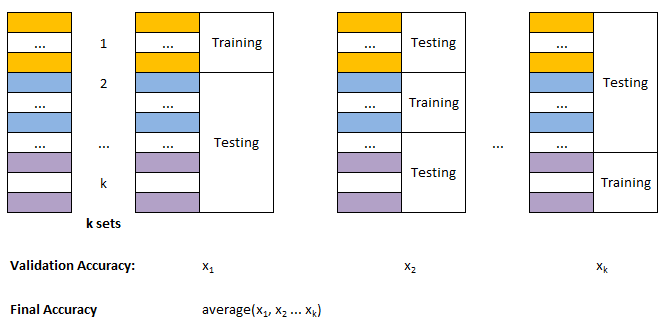Cross validation is a model evaluation methodand "Leave-one-out Cross Validation (LOOCV)" is a K-fold cross validation taken to its logical extreme, with K = N, the number of data points in the set. This method results in N loops where the model or function approximator is trained on all the data except for one point for which the prediction is made.

AI - The Unknown Beast!

I have certainly used the recommendations generated by youTube and viewed many videos based on their recommendations. Though I found them useful, there was nothing extra-ordinary in those recommendations.

One of the possible problem I see is the integrity and authenticity of data/information. I have come across many videos on youTube which are either too repetitive or fake or even factually incorrect. I have heard how AI can diagnose the disease from X-rays and CT-scans. In my opinion, an expert or experience doctor can identify the issue from naked eyes within seconds. These tools are going to make even a naive doctor look like expert! Hence, the AI may help incompetent doctors. How this capability is going to address the patient remains unanswered - will it lead to lesser consultation fee and/or lessser waiting time?

AI tools can also be considered having implications similar to "dynamite and laser". These are used for constructive purposes such as mining and medical diagnosis whereas dangerous aspects like "bomb-lasts and laser-guided missiles" are also known. Is AI going to make "forensic expert's" life easy or tough? Is it going to introduce significant biases in the virtually opaque implementations in customer segmentations?

Identity Theft: E-mail address hunting, Reverse image search, Social Media post scraping, whois service of a website: reveals complete information (phone number, e-mail ID, residential address) if privacy protection is not enabled or purchased. OSINT: Open Source INTelligence is a way to gathering information from social media using usernames.

In the name of company policy, none of the social media platform publich (and shall publish) even a partial list of rules used by them to filter and delete/ban posts on their website. This complete opaque implementation of AI tools is a lethal weapon to mobilize resources to affect public opinion and influence democratic processes. There are posts and videos on YouTube that threaten annihilation of particular community. There are videos still up (as in Dec-2022) where a preacher claims Right of Islam to kill non-muslims and especially few special categories of non-muslims. However, the AI tool is configured such that anybody posting against that video content with same level of pushback (such as non-muslims also have right to kill muslims) shall get suspended from the platform. I firmly believe that any expectation that AI can be used to make the communication balanced, open and honest is just a wishful thinking - AI has created potential to make it more biased and one-sided than traditional modes.

#### Deep Learning

Python, TensorFlow and Keras: TensorFlow derives its name from "operations on tensors" where a tensor is a multi-dimensional array. It contains deep learning libraries which include those from Keras.
##### Web Scraping in Python
The term web scraping refers to collecting information from web sites. However, the same concept can be used to check the consistency and correctness of tags and links in folder structure of a website before uploading the content on host server.

Area of research - Stock Markets

Time series forecasting tries to capture the trend and seasonality (pattern) from past data. However, price movements for a stocks have no particular trend and forecasting capability of any of the existing algorithm is low. Using fast computational speed and reducing transaction time on the exchanges (bourses or trading platform), by tracking price movement in short term (less than a hour) ML can be used for what is known as 'scalping'. Programmers are still trying to predict the price movement. As of now, the algorithm "Long Short Term Memory (LSTM)" has a forecast horizon of 1 day and hence can be used for intra-day trades.

All the codes listed here are outdated as on 2023 as NSE allows access to website www.nseindia.com only through their REST API. NSEPython (pip install nsepython) is a Python library to get data from nseindia.com and niftyindices.com sites by communicating with their REST APIs. The source code is available at github.com/aeron7/nsepython and documentation is available at unofficed.com/nse-python/documentation. As per the code owner of NSEPython, "All the functions of the two famous packages NsepY and NSETools are also migrated here with same function name." The Python module (i.e. code library) contains 59 functions (included in a single file), though not all are to scrape the data. Few common utlities are: nse_quote_ltp, nse_optionchain_ltp, nse_blockdeal...

The functions in the library could be arranged into two categoes: one used for scraping and other for internal use by other functions. payload is the most important variable in the librabry which prepares required URL to retrieve information from NSE website. There are many functions to generate 'payload' such as nse_quote(symbol) where symbol is the name of the scrip. The name of scrip (as per version available in April-2023 has to be in uppercase: for example nse_quote_ltp(RELIANCE) shall work but nse_quote_ltp(reliance) shall not work. Adding symbol = symbol.upper() in funtion nse_quote(symbol) works for both upper and lower case names of the scrips.

You can use this file: nseScraper.py in the same folder where your local code exists, in case you do not want to use "pip install nsepython". Following lines of code can be use to get Last Traded Price (LTP), however it does not work for scrips such as M&M or L&TFH.

```from nseScraper import *
scrip = "tcs"

#This works
print("LTP of " + scrip + " is " + str(nse_quote_ltp(scrip)) + "\n")
#This works
print("Option: " + str(nse_quote_ltp(scrip, "latest", "PE", 3000))) ```

##### Read .csv file and statistical calculations in Python
```# ---------Preliminary Checks--------------------------------------------------
#On WIN10, python version 3.5
#To check that the launcher is available, execute in Command Prompt: py
#To install numPy: C:\WINDOWS\system32>py.exe -m pip install numpy
#To install sciPy: C:\WINDOWS\system32>py.exe -m pip install scipy
#To install pandas: C:\WINDOWS\system32>py.exe -m pip install pandas
#To install matplotlib: C:\WINDOWS\system32>py.exe -m pip install matplotlib
#To get list of installed packages: C:\WINDOWS\system32>py.exe -m pip freeze
# -----------------------------------------------------------------------------

import numpy as npy    # Remember: NumPy is zero-indexed

#import pandas as pnd
#from pandas import Series, DataFrame

import scipy
from scipy import stats

import matplotlib.pyplot as plt

# ----------Read data from TXT/CSV/XLSX formats-------------------------------
# Get data from a SIMPLE txt fie. loadtxt does not work with mixed data type
# rawData = npy.loadtxt('statsData.txt', delimiter=' ', skiprows=1, dtype=str)

# Useful for COLUMNS with STRINGS and missing data
#rawData = npy.genfromtxt("statsData.txt", dtype=None, delimiter=" ", skip_header=1, names=True)

# Get data from a CSV file
# -----------------------------------------------------------------------------
npy.set_printoptions(precision=3)    #Precision for floats, suppresses end zeros
npy.set_printoptions(suppress=True)  #No scientific notation for small numbers

#Alternatively use formatter option, it will not suppress end ZEROS
npy.set_printoptions(formatter={'float': '{: 8.2f}'.format})

mean = npy.mean(dataRaw, axis = 0)  # axis keyword: 0 -> columns, 1 -> rows
print("Mean:     ", mean)
medn = npy.median(dataRaw, axis = 0)
print("Median:   ", medn)
sdev = npy.std(dataRaw, axis = 0)
print("SD:       ", sdev)

# Generate plot
n = dataRaw[:,1].size               #Python arrays are 0-based
x = npy.arange(1, n+1)
y = dataRaw[:, 1]                   #Read first column of the input data
plt.title("Crude Price")
plt.xlabel("Day")
plt.ylabel("Crude Price in US\$")
plt.plot(x,y)
plt.show()```
The file statsCSV.txt can be found here.
 Input: stockLists.txt Output: boardMeetDates.txt ```AMARAJABAT BATAINDIA BEML BHARTIARTL CANFINHOME RELIANCE HDFCBANK INFY LUPIN TCS``` ```Symbol BoardMeetingDate AMARAJABAT 09-Nov-2018 BATAINDIA 02-Nov-2018 BHARTIARTL 25-Oct-2018 CANFINHOME 22-Oct-2018 HDFCBANK 20-Oct-2018 JSWENERGY 02-Nov-2018 LUPIN 31-Oct-2018```
Some of the scrips are skipped as board-meeting dates are not available. Also note that the output is sorted in ascending order irrespective of the order of scrip codes in input file.

The following graph describes how only a fraction of shares traded as compared to available tradeable share (free-float shares) cause large price movements.The data has been scraped through NSE website using a Python code. This code requires list of NIFTY stocks stored in a text file, sample can be found here.
##### Python: PDF and File Utility Scripts

This Python code renames all the files of a specific type (say pdf or txt) in a folder by stripping specified number of characters at the start of the file name with new prefix. The code has to be placed in the folder containing the files. In case you incorrectly specified the new prefix, you can adjust number of characters to be stripped at the start of the file names.

In case you want to compress just one PDF file, one can use this code.

Few pages of files can be extracted using Ghostview with command: gswin64 -sDEVICE=pdfwrite -dNOPAUSE -dBATCH -dSAFER -dFirstPage=14 -dLastPage=17 -sOutputFile=OUT.pdf In.pdf -dNOPAUSE option disables the prompt and pause after each page is processed, -dBATCH option causes Ghostscript to exit when processing of the file specified in the command has finished.

-sPageList=pagenumber There are three possible values for this; even, odd or a list of pages to be processed. A list can include single pages or ranges of pages. Ranges of pages use the minus sign '-', individual pages and ranges of pages are separated by commas ','. A trailing minus '-' means process all remaining pages. For example:

-sPageList=1,3,5 indicates that pages 1, 3 and 5 should be processed. -sPageList=even to refer all even-numbered pages. -sPageList=odd refers to all odd-numbered pages.

-sPageList=5-10 indicates that pages 5, 6, 7, 8, 9 and 10 should be processed

-sPageList=1,5-10,12- indicates that pages 1, 5, 6, 7, 8, 9, 10 and 12 onwards should be processed

Be aware that using the '%d' syntax for OutputFile does not reflect the page number in the original document. If you chose (for example) to process even pages by using -sPageList=even, then the output of -sOutputFile=out%d.png would still be out0.png, out1.png, out2.png ...

To rasterize [to convert an image described in a vector graphics format (shapes) into image with a series of pixels, dots or lines] all of the text in a PDF: Step-1) Convert the PDF into TIFF using ghostview -- gswin64 -sDEVICE=tiffg4 -o Out.tif Inp.pdf -> Step-2) Convert the TIFF to PDF using "tiff2pdf -z -f -F -pA4 -o New.pdf Out.tif". Alternatively: "gswin64c -dNOPAUSE -dBATCH -dTextAlphaBits=4 -sDEVICE=ps2write -sOutputFile=Out.ps Inp.pdf" then "gswin64c -dNOPAUSE -dBATCH -sDEVICE=pdfwrite -sOutputFile=New.pdf Out.ps". Options -dTextAlphaBits=4 is used for font antialiasing and works only if text in PDF file is in pixel format else error message "Can't set GraphicsAlphaBits or TextAlphaBits with a vector device." gets printed in console [anti-aliasing is used to describe the effect of making the edges of graphics objects or fonts smoother], The subsampling box size should be 4 for optimum output, but smaller values can be used for faster rendering. Antialiasing is enabled separately for text and graphics content. Allowed values are 1, 2 or 4. Reoslution is set by -r300 for 300 dpi

In imaging, alias refers to stair-stepping of lines. Anti-aliasing refers to the reduction in stair-stepping. Aliasing also refers to sampling of a single at low rate.

Following command can be used to create a PNG files for each page of a PDF file. The command line option '-sDEVICE=device' selects which output device Ghostscript should use. If device option isn't given the default device (usually a display device) is used. Ghostscript's built-in help message (gswin64 -h) lists the available output devices.

C:\Users\XYZ>gswin64 -dSAFER -dBATCH -dNOPAUSE -sDEVICE=pnggray -r300 -dTextAlphaBits=4 -sOutputFile=PDF2PNG-%04d.png In.pdf

gswin64 -dSAFER -dBATCH -dNOPAUSE -sDEVICE=pnggray -r300 -dTextAlphaBits=4 -dFirstPage=1 -dLastPage=10 -sOutputFile=PDF2PNG-%04d.png -dUseArtBox In.pdf --Set the page size using the pair of switches: -dDEVICEWIDTHPOINTS=w -dDEVICEHEIGHTPOINTS=h where 'w' = desired paper width and 'h' = desired paper height in points (1 point = 1/72 inch) and 1 pixel = 10 dots. Example - A4 size is height x width = 210 x 297 [cm] = 8.27 x 11.7 [inch] = 595 x 842 [points]. This will translate into -r300 -g2481x3510. Ghostscript may sometimes convert PDF to PNG with wrong output size. Use -dUseCropBox or -dUseTrimBox: note that these two options are mutually exclusive. Also -sPAPERSIZE=a4 cannot be used with -dUseCropBox or -dUseTrimBox. With -dPDFFitPage Ghostscript will render to the current page device size (usually the default page size). If the dimensions of PNG pages are different from those in PDF file, adjust -r300 to -r100 or -r160 till you get desired size. Note: Pixel is the smallest unit a screen can display, Dot is the smallest thing a printer can print.

Other options are: -sDEVICE=pngmono, -sDEVICE=jpeg / jpeggray, -sDEVICE=bmp16 / bmp256 / bmpgray / bmpmono ... are few out of almost 150 options available. pngmono - Monochrome Portable Network Graphics (PNG), pnggray is 8-bit gray PNG, png16 is 4-bit colour PNG, png256 is 8-bit colour PNG and png16m is 24-bit colour PNG.

-dUseBleedBox: Defines the region to which the contents of the page should be clipped when output in a production environment. Sets the page size to the BleedBox rather than the MediaBox. This may include any extra bleed area needed to accommodate the physical limitations of cutting, folding, and trimming equipment. The actual printed page may include printing marks that fall outside the bleed box.

-dUseTrimBox: The trim box defines the intended dimensions of the finished page after trimming. Sets the page size to the TrimBox rather than the MediaBox. Some files have a TrimBox that is smaller than the MediaBox and may include white space, registration or cutting marks outside the CropBox. Using this option simulates appearance of the finished printed page.

-dUseArtBox: The art box defines the extent of the page's meaningful content (including potential white space) as intended by the page's creator. Sets the page size to the ArtBox rather than the MediaBox. The art box is likely to be the smallest box. It can be useful when one wants to crop the page as much as possible without losing the content.

-dUseCropBox: Sets the page size to the CropBox rather than the MediaBox. Unlike the other "page boundary" boxes, CropBox does not have a defined meaning, it simply provides a rectangle to which the page contents will be clipped (cropped). By convention, it is often, but not exclusively, used to aid the positioning of content on the (usually larger, in these cases) media.

#### Operations on PDF Files

convert PDF files with text and coloured background into PDF with Black-and-White format: There are two approaches. In apporach-1, the Ghostview has been used to convert the PDF pages into PNG files and then Pillow/OpenCV has been used to convert the PNG into PDF with Black-and-White format. In second approach, PyMuPDF has been used to convert the PDF into PNG files. Other steps remain same as approach-1. The Python script can be downloaed from here. One of the important step is to find a right value of threshold which results in sharper text and whiter background. The program runs in serial mode and hence it is a bit slow: it may take up to 15 minutes for a PDF having 100 pages. There are other operations needed on PDF with scanned images such as:

1. Deskew: straighten the text on a page
2. Check resolution: the resolution of image is measured in PPI (pixels per inch) or DPI (dots per inch).
3. Sharpen the texts
4. Align texts on the centre of the pages
5. Create equal margins on all pages
DPI: Dots per Inch is a sort of meta-data stored inside image file to tell a device how to display or print it. In other words - it is an indication of zoom level when an image is moved from one device to other. In display devices, DPI indicates sharpness of the illuminated points. In printing devices, it indicates the sharpness of printed characters or outlines. Resampling is the process of changing the pixel dimensions of an image maintaining the content of original image.

Convert PDF to PNG using Ghostview and Python

```import os, sys, subprocess
resolution = 200
i = 1  #Start page
j = 5  #Last page

pdf_name = str(sys.argv)
# Make directory named PDF2PNG
output_dir = "PDF2PNG"
os.makedirs(output_dir, exist_ok=True)

file_name = os.path.basename(pdf_name)
file_name = file_name.split(".")
png_name = output_dir + "/" + file_name + "-%04d.png"

#Make sure that the Ghostview is defined in PATH
gs = 'gswin32c' if (sys.platform == 'win32') else 'gswin64'

# {f-strings}: syntax is similar to str.format() but less verbose
subprocess.run(["gswin64", "-dBATCH", "-dNOPAUSE", "-sDEVICE=png16m", f"-r{resolution}", f"-dFirstPage={i}", f"-dLastPage={j}", f"-sOutputFile={png_name}", f"{pdf_name}"], stdout=subprocess.PIPE) ```

Convert PDF to PNG using PyMuPDF

```import fitz
inPDF = "00_Rigved.pdf"
prefix = "RV-Book-1"
doc = fitz.open(inPDF)
nPg = len(doc)

#iPg = doc.loadPage(0)   #Extract specific page
i = 1
for page in doc:
pg = page.getPixmap()
#outFile = prefix + str(n).zfill(i) + ".png"
outFile = prefix + '{0:04}'.format(i) + ".png"
pg.writePNG(outFile)
i = i + 1 ```

This Python code saves front (cover) page of all PDF files stored in a folder into PNG files. It has option to generate HTML tags to add the images as inline objects in a web page.

Delete pages from a PDF file using PyPDF2: it creates a new file by adding suffix _new. The pages to be deleted can also be specified as list or a range of numbers.

```from PyPDF2 import PdfFileReader, PdfFileWriter, PdfFileMerger
from pathlib import Path
import sys, os
#--Syntax: py delPages.pdf Input.pdf m n-----N < 0 implies single page deletion
file_name = str(sys.argv)

file_path = os.getcwd() + "\\" + file_name

m = int(sys.argv)
n = int(sys.argv)

new_file = file_path.strip(".pdf") + "_new.pdf"
out_pdf = PdfFileWriter()
#
#Note that the counter i starts with ZERO
if (n >= 0):
for i in range(in_pdf.getNumPages()):
p = in_pdf.getPage(i)
if (i >= m and i <= n):
else:
for i in range(in_pdf.getNumPages()):
p = in_pdf.getPage(i)
if (i != m):

with open(new_file, 'wb') as f:
out_pdf.write(f)
```

Crop pages in a PDF file using PyPDF2:

In Ghostview, -dPDFFitPage can be used to select a PageSize given by the PDF MediaBox. The PDF file will be scaled to fit the current device page size (usually the default page size). Other options are -dUseBleedBox, -dUseTrimBox, -dUseArtBox or -dUseCropBox. This is useful for creating fixed size images of PDF files that may have a variety of page sizes, for example thumbnail images. This option is also set by the -dFitPage option.

```from PyPDF2 import PdfFileReader, PdfFileWriter, PdfFileMerger
from pathlib import Path
import sys
#-------------------------------------------------------------------------------
#Syntax: pdfcrop.py original.pdf 20 30 20 40

file_name = "Original.pdf"
pdf_path = (Path.home()
/ file_name
)

#-------------------------------------------------------------------------------
#print(file_name.strip(".pdf"))
new_file = file_name.strip(".pdf") + "_new.pdf"

left     = int(sys.argv)
top      = int(sys.argv)
right    = int(sys.argv)
bottom   = int(sys.argv)

out = PdfFileWriter()
for page in pdf.pages:
page.mediaBox.upperRight = (page.mediaBox.getUpperRight_x() - right, \
page.mediaBox.getUpperRight_y() - top)
page.mediaBox.lowerLeft  = (page.mediaBox.getLowerLeft_x()  + left,
page.mediaBox.getLowerLeft_y()  + bottom)

out_pdf = open(new_file, 'wb')
out.write(out_pdf)
out_pdf.close()```

Scale Pages:

```from PyPDF2 import PdfFileReader, PdfFileWriter, PdfFileMerger
from pathlib import Path
import sys, os
#--Syntax: py scapePages.py Input.pdf 0.5--------------------------------------
file_name = str(sys.argv)
file_path = os.getcwd() + "\\" + file_name

#Enter scaling factors as fraction, all pages shall be scaled down/up
s = float(sys.argv)

new_file = str(file_path.strip(".pdf") + "_scaled.pdf")
out_pdf = PdfFileWriter()
#
#Note that the counter i starts with ZERO
for i in range(in_pdf.getNumPages()):
p = in_pdf.getPage(i)
p.scaleBy(s)

with open(new_file, 'wb') as f:
out_pdf.write(f) ```
Merge PDF Files

Using Ghostview for Windows, files can be merged directly on command prompt: gswin64 -sDEVICE=pdfwrite -dNOPAUSE -dBATCH -dSAFER -sOutputFile=x.pdf 1.pdf 2.pdf 3.pdf -Note that there should be no space in -sDEVICE=pdfwrite and -sOutputFile=combined.pdf such as -sDEVICE = pdfwrite and/or -sOutputFile = combined.pdf

Once GhostView is installed, you need to set the location in PATH using Control Panel - System - Advanced System Settings - Advance - Environment Variables.
```from PyPDF2 import PdfFileReader, PdfFileWriter, PdfFileMerger
from pathlib import Path
import sys, os
#----------------------Syntax: py pdfMerge.py F1.pdf F2.pdf F3.pdf F4.pdf------
#Any number of files can be specified on command line. Input files must be in
#folder from which command is executed. e.g. py ../mergePdf.py F1.pdf F2.pdf
#
if (len(sys.argv) < 2):
print("\nUsage: python {} input.pdf m n \n".format(sys.argv))
sys.exit(1)
#------------------------------------------------------------------------------
fname = []
inpdf = []
j = len(sys.argv)
for i in range(1, j):
fname.append(str(sys.argv[i]))
fx = str(sys.argv[i])
new_file =  os.getcwd() + "\\" + "Merged_File.pdf"
out_pdf = PdfFileWriter()
#
for f in inpdf:
for k in range(f.getNumPages()):
p = f.getPage(k)
with open(new_file, 'wb') as f:
out_pdf.write(f)```

Shuffle Pages

Rotate Pages

This Python code can be used to combine short lines of a text file into bigger lines. This is especially useful when texts are extracted from PDF files with OCR characters embedded in it.

List Files of a Folder

```import os
import sys
#The code is run by defining the path at the command line argument
#e.g. py listDir.py . or py listDir.py ./abc/pqr

print("Synatax:: ", sys.argv)
file_list = []
for file in os.listdir(sys.argv):
if file.endswith(".py"):
file_list.append(file)```

PDF Reference, Third Edition, Adobe Portable Document Format Version 1.4: THE ORIGINS OF THE Portable Document Format and the Adobe Acrobat product family date to early 1990. At that time, the PostScript page description language was rapidly becoming the worldwide standard for the production of the printed page. PDF builds on the PostScript page description language by layering a document structure and interactive navigation features on PostScript's underlying imaging model, providing a convenient, efficient mechanism enabling documents to be reliably viewed and printed anywhere. At the heart of PDF is its ability to describe the appearance of sophisticated graphics and typography. This is achieved through the use of the Adobe imaging model, the same high-level, device-independent representation used in the Post-Script page description language.

The appearance of a page is described by a PDF content stream, which contains a sequence of graphics objects to be painted on the page. This appearance is fully specified where all layout and formatting decisions have already been made by the application generating the content stream.

List file names, size and number of pages
```# References:
#------------------------------------------------------------------------------
# stackoverflow.com/questions/2104080/how-can-i-check-file-size-in-python
# www.geeksforgeeks.org/python-os-path-size-method
# www.geeksforgeeks.org/python-program-to-convert-a-list-to-string
# stackoverflow.com/questions/541390/extracting-extension-from-filename-in-python
# stackoverflow.com/questions/4226479/scan-for-secured-pdf-documents
# pythonexamples.org/python-if-not/
#------------------------------------------------------------------------------
import sys,os
root = "F:\World_Hist_Books"
path = os.path.join(root, "targetdirectory")
'''
#------------------------------------------------------------------------------
#Get content of a directory: files, directories as LIST in the terminal
out_f = "List.txt"

#Write Only ('w') : Open the file for writing. If file already exits, data is
#truncated and over-written. The handle is positioned at the beginning of the
#file. Creates the file if it does not exist.
f = open(out_f, "w")     # f = open("List.txt", "w")

s = os.listdir()
for x in s:
#print(x)
f.write(x + '\n')
f.close
#------------------------------------------------------------------------------
'''
out_f = "List.txt"
f = open(out_f, "w")
def convert_bytes(num):  #bytes to kB, MB, GB
for x in ['bytes', 'KB', 'MB', 'GB', 'TB']:
if num < 1024.0:
return "%3.1f %s" % (num, x)
num /= 1024.0
for path, subdirs, files in os.walk(root):
for name in files:
s = os.path.join(path, name)
b = os.path.getsize(os.path.join(path, name))
b = convert_bytes(b)
#Get number of pages in the PDF file: f.split(".")[-1]
ext = os.path.splitext(s)[1:].strip().lower()
nPg = 0
if (ext.upper() == "PDF"):
with open(s, 'rb') as pdf_file:
if not pdf_f.isEncrypted:
nPg =  pdf_f.getNumPages()
pdf_file.close()
#Replace \ with whitespace
A = s.split('\\')
f.write(' '.join(map(str, A)))
f.write(' ' + str(b) + '  ' + str(nPg) + '\n')

#Write only the file names
#f.write(s.split('\\')[-1] + '\n')
f.close
#------------------------------------------------------------------------------
# L is the list
#listToStr = ' '.join([str(elem) for elem in L])
#listToStr = ' '.join(map(str, L))
#print(listToStr)
#------------------------------------------------------------------------------
```

##### Linux Commands and Utilities
#Bash is the GNU shell, compatible with the Bourne shell and incorporating many useful features from other shells. When the shell is started, it reads its configuration files. The most important are: /etc/profile, ~/.bash_profile and ~/.bashrc. The env or printenv commands can be used to display environment variables. Using the set built-in command without any options will display a list of all variables (including environment variables) and functions. Write script to determine whether given file exist or not, file name is supplied as command line argument, also check for sufficient number of command line argument. Here '\$#' stands for total nmber of the arguments passed to a command or program. '\$0' is the name of the program or the shell script, '\$1' is 1st argument, '\$2' ... '\$9' is limit. #A special variable '\$?' holds the return value of a program. '\$\$' expands to process ID of the shell. '\$!' expands to the process ID of the most recently executed background (asynchronous) command.

set -o noclobber: #noclobber option prevents existing files from being overwritten by redirection operations. Use - (dash) for enabling an option, + for disabling: eg set +o noclobber

set -o noglob: noglob option prevents special characters from being expanded
```# File to customize a users environment
alias la='ls -lhX'
alias rm='rm -i'
alias mv='mv -i'
alias cp='cp -i'
alias c='clear'
alias x='exit'
alias du='du -chs'
#------------------------------------------------------------------------------
#Extended Brace expansion.
echo {a..z} # a b c d e f g h i j k l m n o p q r s t u v w x y z
# Echoes characters between a and z
echo {0..3} # 0 1 2 3
# Echoes characters between 0 and 3
#------------------------------------------------------------------------------
cat *.lst | sort | uniq
# Merges and sorts all ".lst" files, then deletes duplicate lines
#------------------------------------------------------------------------------
#!/bin/bash
# uppercase.sh : Changes input to uppercase
tr 'a-z' 'A-Z'
#  Letter ranges must be quoted to prevent single-lettered filename generation
exit 0
# On the command prompt, use \$>ls -l | uppercase.sh
#------------------------------------------------------------------------------```

Install a package (program): sudo apt install okular

Uninstall a package excluding dependent packages: sudo apt remove okular

Uninstall a package including dependent packages: sudo apt remove --auto-remove okular

Uninstall a package including configuration and dependent packages: sudo apt purge okular

Uninstall everything related to a package (recommended before reinstalling a package): sudo apt purge --auto-remove okular

Change / rename an user's account: sudo usermod -l new_name old_name. Once an user's account is renames, the home directory needs to be updated inline with the user's name: sudo usermod -d /home/new_name -m new_name

##### Utilities related to Disk and Network

lsblk: List the block device mounted. Similar to ls command which lists files and folders.

mkfs - build a Linux filesystem, fdisk - manipulate disk partition table - sometimes fdisk does not work - use gdisk, fsck - check and repair a Linux filesystem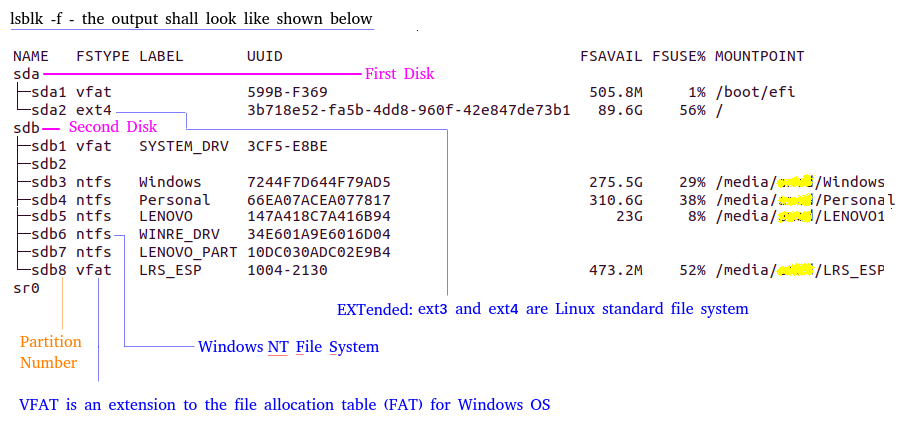Error Message: The backup GPT table is corrupt, but the primary appears OK, so that will be used. GPT = GUID Partition Table is a popular disk partitioning scheme used across most operating systems. Use GPT fdisk = gdisk to verify and update the GPT of hard disk.

##### Utilities related to Files and Folder
Find the files which differ or that may not exist in either directory: diff --brief --recursive --new-file folder1/ folder2/ which is equivalent to diff -qrN folder1/ folder2/. Note that -q is short flag aliase for long option --brief, -r for --recursive and -N for --new-file.

Find files greater or smaller than certain size: find . -type f -size +1M or find . -type f -size -1M where the suffix 'b' refers 512-byte blocks (default), 'c' stands for bytes, 'k' implies Kilobytes, 'M' is for Megabytes and 'G' for Gigabytes. +1M implies file ≥ 1 MB and -1M shall search for files < 1 MB.

##### Edit epub Files
EPUB files are structurally a compressed (ZIP) file containing XHTML text files, images, and other resources. To unzip the epub to the folder 'eBook': "unzip eBbook.epub -d eBook". To zip up an epub, follow the following steps. Note that the option -r stands for recursive operation and -g is needed to add additional files (grow) to interim zip folder.
1. zip -X newEbook.epub eBook/mimetype
2. zip -rg newEbook.epub eBook/META-INF -x \*.DS_Store
3. zip -rg newEbook.epub eBook/OEBPS -x \*.DS_Store
Alternatively, you can also use: zip -rX newEbook.epub eBook/mimetype eBook/META-INF/ eBook/OEBPS/. Note that this process of unzipping and zipping may delete some of the meta data such as Author's name, Published Date and Tags.

Calibre

This package can be used to view, edit and convert ePUB files. The Calibre install provides the command ebook-convert that runs from command line and there's no need to run Calibre. For example: "ebook-convert eBook.epub eBook.pdf --enable-heuristics" can be used to convert a EPUB file to PDF. Mlti-column PDFs are not supported and this command line operation shall not work, only way left is to edit the PDF in GUI mode.

##### Web Technology
 Front-End Back-end Full Stack Topic dealing with user interface, what the end user see and interact with Deals with methods required to create, maintain and provide data needed for Front End Deals with both Front End and the Back End technologies Mainly programmed in HTML, CSS, JavaScript, PHP Deals with databases and server-side scripting: PHP, ASPX, SQL, JSON, Angular, ReactJS In addition to Front End and Back-end, the developer has to deal with servers (ports, networks, web-hosting), data security and database management. MangoDB, NodeJS, PHP, Python

Server Administration, Data Security and Hacking: Social Media Hunting, Data Theft, Tracking Personal Information

There are 52 characters (including lower and upper cases), 10 digits and 32 special characters on computer keyboard. If one does not allow to have passoword starting with special character and minimum size of password is set to be 8 characters, just for passwords having 8 characters, 62 x 947 = 4020 trillion combinations are possible. Note passwords can be any size higher than 8 characters but usually it would limited to 12 ~ 16 characters as users needs to remember them.
The image shown below highlights some of the terms and concepts related to machine learning and serves the purpose to highlight the fact that machine learning in itself is not an entirely new technology but a combination of mathematics, statistics, probability and computer programming. Some of the activities most of us consciously or unconsciously already do in our routine work though on a much smaller scales.##### Reference
• www.cs.huji.ac.il/~shais/UnderstandingMachineLearning
• Videos on YouTube by NPTEL and Andrew NG

The content on CFDyna.com is being constantly refined and improvised with on-the-job experience, testing, and training. Examples might be simplified to improve insight into the physics and basic understanding. Linked pages, articles, references, and examples are constantly reviewed to reduce errors, but we cannot warrant full correctness of all content.